## Kappa tools reference manual (release v4.1-14-g897fd18)

corresponding author: jean.krivine@irif.fr

## Chapter 1Introduction### 1.2 Preamble

This manual describes the Kappa language and details the usage of its tool suite.

Kappa is one member of the growing family of rule-based languages. Rule-based modelling has attracted recent attention in developing biological models that are concise, comprehensible, easily extensible, and allows one to deal with the combinatorial complexity of multi-state and multi-component biological molecules.

From the description of a system by the deﬁnition of a set of entities and the enumeration of their local rule of interraction, Kappa tools provide a framework to study statically and dynamically the system without ever enumerating all its reachable states (unless very explicitely asked to by users).

In Kappa, a mixture of entities is represented as a site graphs and temporal local transformations as rewrites.

First contact with Kappa as well as interactive model developement could occurs in the Kappapp available online and as downloadable software on main platforms. Intensive scientiﬁc usage should occurs by scripting around the command line tools or by using the Python client.

After a small teaser, this manual provides an exhaustive list of what can be done and how with the tools. It is not intended as a tutorial on rule-based modelling.

To get an idea of how Kappa is used in a modelling context, the reader can consult the following note Agile modelling of cellular signalling (SOS’08). A longer article, expounding on causal analysis is also available: Rule-based modelling of cellular signalling (CONCUR’07). See also this tutorial: Modelling epigenetic information maintenance: a Kappa tutorial.

### 1.3 Show me a running example

See it really running in the online user interface by clicking on the try button on https://www.kappalanguage.org/.

A minimal Kappamodel looks like:

1/* Signatures*/
2%agent: A(x, c)             // Declaration of agent A
3%agent: B(x)               // Declaration of agent B
4%agent: C(x1{u p}, x2{u p}) // Declaration of agent C with 2 modifiable sites
5/* Variables */
6%var: on_rate  1.0E-4 // per molecule per second
7%var: off_rate 0.1    // per second
8%var: mod_rate 1      // per second
9/* Rules */
10a.b A(x[.]),B(x[.]) <-> A(x),B(x) @ on_rate, off_rate       //A and B bind and dissociate
11ab.c A(x[_], c[.]),C(x1{u}[.]) -> A(x[_], c),C(x1{u}) @ on_rate //AB binds unphosphorilated C
12modx1 C(x1{u}),A(c) -> C(x1{p}[.]),A(c[.]) @ mod_rate        //ABC modifies x1
13a.c A(x[.],c[.]), C(x1{p}[.], x2{u}[.]) ->
14      A(x[.],c), C(x1{p][.], x2{u}) @ on_rate    //A binds x1_phos C on x2
15modx2 A(x[.], c),C(x1{p}[.], x2{u}) ->
16         A(x[.], c[.]),C(x1{p}[.], x2{p}[.]) @ mod_rate  //AC modifies x2
17/* Observation */
18%obs: AB  |A(x[x.B])|
19%obs: Cuu |C(x1{u}, x2{u})|
20%obs: Cpu |C(x1{p}, x2{u})|
21%obs: Cpp |C(x1{p}, x2{p})|
22/*Initial conditions */
23%init: 1000  A(),B()
24%init: 10000 C(x1{u}, x2{u})

Lines 1-4 of this kappa ﬁle contain signature declarations. Agents of type C have two sites x1 and x2 whose internal state may be u (unphosphorylated) or p (phosphorylated). Line 11, rule 'ab.c' binds an A connected to someone on site x to a C.

There are two main points to notice about this model: A can modify both sites of C once it is bound to them. However, only an A bound to a B can connect on x1 and only a free A can connect on x2. Note also that x2 is available for connection only when x1 is already modiﬁed.

We try ﬁrst a coarse simulation of $100,000$ events (10 times the number of agents in the initial system).

• KaSim ABC.ka -u event -l 100000 -p 1000 -o abc.csv

Plotting the content of the abc.csv ﬁle, one notices that nothing signiﬁcantly interesting happens to the observables after 250s. So we can now specify a meaningful time limit by running:

• KaSim ABC.ka -l 250 -p 0.25 -o abc.out

which produces the data points whose rendering is given in Fig. 1.1.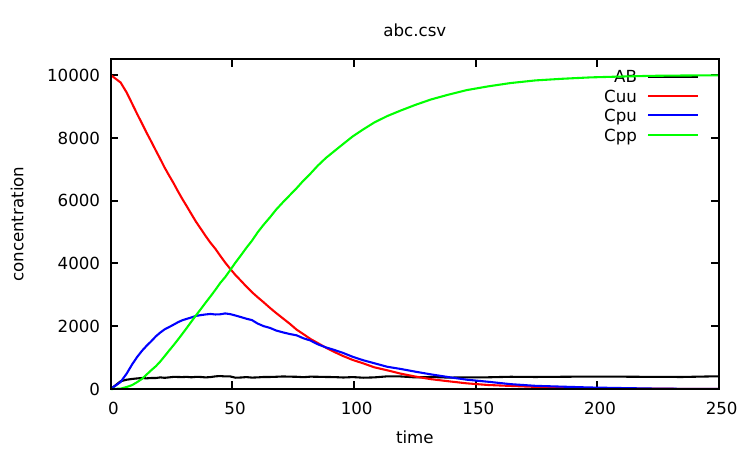Figure 1.1: Simulation of the ABC model: population of unmodiﬁed Cs (in red is the observable Cuu) drops rapidly and is replaced, in a ﬁrst step by simply modiﬁed Cs (in blue is the observable Cpu) which are in turn replaced by doubly modiﬁed Cs (in red is the observable Cpp). Note that, the population of AB complexes (observable AB in black) stabilizes slightly below 400 individuals after about 20s.

We will use variant of this model as a running example for the next chapter.

## Chapter 2The Kappa language

### 2.1 General structure

A model is represented in Kappa by a set of Kappa Files. We use KF to denote the union of the ﬁles that are given as input to a tool.

A KF is composed of a list of declaration. Declarations can be: agent and token signatures (Sec. 2.3), rules (Sec. 2.5), variables (Sec. 2.4), initial conditions (Sec. 2.6), intervention (Sec. 2.7) and parameter conﬁgurations (Sec. 5.4).

The KF’s structure is quite ﬂexible. Neither dividing into several sub-ﬁles nor the order of declarations matters (for the exception of interventions and variable declarations, see respectively Sections 2.7 and 2.4 for details).

Comments works like in the C language. It can be used either by inserting the marker // that tells KaSim to ignore the rest of the line or by putting any text between the delimiters /* and */.

The following sections present formal grammars. Here are hints to read them. Terminal symbols are written in (blue) typed font, and $𝜀$ stands for the empty list. An identiﬁer Id can be any string generated by a regular expression of the type $\text{_}\phantom{\rule{1em}{0ex}}{\left[a-z\phantom{\rule{1em}{0ex}}A-Z\phantom{\rule{1em}{0ex}}0-9\phantom{\rule{1em}{0ex}}\text{_}\phantom{\rule{1em}{0ex}}-\phantom{\rule{1em}{0ex}}+\right]}^{+}|\left[a-z\phantom{\rule{1em}{0ex}}A-Z\right]{\left[a-z\phantom{\rule{1em}{0ex}}A-Z\phantom{\rule{1em}{0ex}}0-9\phantom{\rule{1em}{0ex}}\text{_}\phantom{\rule{1em}{0ex}}-\phantom{\rule{1em}{0ex}}+\right]}^{\ast }$.

### 2.2 Sited-graph pattern: Kappa expression

The state of the system is represented in Kappa as a sited graph: a graph where edges use sites in nodes. One must think sites as resources. At most one edge of the graph can use a site of a node (representing an agent in our case). Moreover, all the sites of an agent must have diﬀerent names.

This leads to the property that an embedding between 2 sited graphs is completely deﬁned by the image of one node. This is absolutely critical for the eﬃciency and we call this concept the rigidity of Kappa.

Table 2.1: Kappa expressions.
 Kappa_expression ::= agent_expression , Kappa_expression $\mid 𝜀$ agent_expression ::= Id(interface) interface ::= Id internal_state link_state , interface $\mid 𝜀$ internal_state ::= $𝜀$ | {.} | {Id} link_state ::= $𝜀$ | [.] | [n] | [_] | [#] | [Id.Id]

#### 2.2.1 Graphs

The ASCII syntax we use to represent sited graphs follows the skeletons (describe formally in Table 2.1):

• We write the type of the agent and then its interface (the space separated list its sites) between parenthesis.
• The state of a site is written after its name. Sites can have 2 kind of states: a linking state and an internal states. The order in which they are speciﬁed does not matter.
• The linking state of a site is written in between squared brackets: []
• The internal state of a site is written in between curly brackets: {}
• When the site is free (i.e. it is not a member of an edge), its linking state is written with a dot: [.]. For example, the following graph: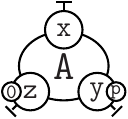is written as A(x[.], y{p}[.], z{e0}[.]).

• When a site is a part of an edge, one assign an arbitrary positive integer identiﬁer $n$ to this edge and one specify the appurtenance of the site to this edge by writing the linking state [n]. The following graph: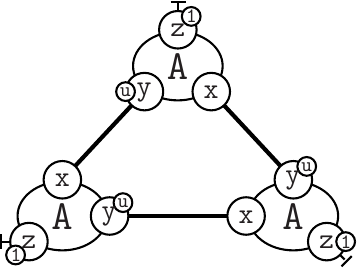can be reprensented as A(x, y{u}, z{e1}[.]), A(x, y{u}, z{e1}[.]), A(x, y{u}, z{e1}[.]).

Remark Each link identiﬁer appears exactly twice.

#### 2.2.2 Patterns

Kappa strength is to describe transformations by only mentioning (and storing) the relevant part of the subgraph required for that transformation to be possible. This is the don’t care, don’t write (DCDW) principle which plays a key role in resisting combinatorial explosion when writing models.

If a transformation occurs independently from the state of a site of an agent, do not mention it in the pattern to match. The pattern A(x[.],z[.]) represents an agent of type A whose sites x and z are free but the sites y and z can be in any internal state and the site y can be linked or not to anything.

If the link state of a site does not matter but the internal state does, just mention it. An agent A whose sites x and z are free, y is in state u and z in state e2 is written as A(x[.],y{u},z{e2}[.]).

A state that is modiﬁed (by a rule that will be presented just below) always matter. For such situation, the symbol # (meaning “whatever” state) has been introduced.

In Kappa, in order to require a site to be bound for an interaction to occur, one may use the semi-link construct [_] which does not specify who the partner of the bond is. For instance, in the following instruction: %var: ab|A(x[_]),B(y[_])|, the variable 'ab' will count the number of As and Bs connected to some agents, including the limit case A(x),B(y). It is sometimes convenient to specify the type of the semi-link, in order to restrict the choice of the binding partner. For instance, in the following instruction: %var: ab|A(x[y.B]),B(y[x.A])|, the variable 'ab' will count the number of As whose site x is connected to a site y of B, plus the number of Bs whose site y is connected to a site x of A. Note that, this still includes the case A(x),B(y).

Remark Transformations on semi-links and links type induce side eﬀects (eﬀect on unmentioned agents/unmentioned site of agent) and can even do not make sense at all. What would mean to remove the link to A but not the link to B in the example above? Be careful when one use them.

### 2.3 Agent signatures

Kappa tools can seek in the KF what agents are used, what sites they have and what states the sites are in but it is error prone: just make a typo once and the tools won’t complain and create a nonsens new agent/site/states...

To avoid that, Agent signatures can be deﬁned and tools will then ensure that agents respect their signature.

Agent signatures list all the agents that will appear in the KF. They enumerate the name of interaction sites an agent has. They provide information about sites binding capabilities. They specify whether a site has internal state and if so give the possibilities.

A signature is declared in the KF by the following line:

• %agent: signature_expression

according to an extention of the grammar given in Table 2.1. Linking states and internal states are space separated lists instead of being singleton. Site binding capabilities are speciﬁed by giving a list typed semi-links.

For instance, the line:

1%agent: A(x[y.A], y{u p}[x.A], z{e0 e1 e2}) // Signature of agent A

will declare an agent A with 3 (interaction) sites x,y and z, the site y possessing two internal states u and p (for instance, for the unphosphorylated and phosphorylated forms of y) and the site z having three possible states $e0$, $e1$ and $e2$, sites x and sites y being able to bind (intra agent or inter agents).

Special case If no agent signature provides any site binding capabilities, constraints are released and any site of any agent is allowed to bind any site of any agent.

### 2.4 Algebraic expressions, variables and observables

Algebraic expressions original purpose was to deﬁne kinetic rates for rules but many components of a KF will now implies algebraic expressions. Their syntax are deﬁned in Table 2.2 (available symbols for variable, constants and operators are given in Table 2.3).

Table 2.2: Algebraic expressions.
 algebraic_expression ::= $x\in ℝ$ $\mid$ variable $\mid$ algebraic_expression binary_op algebraic_expression $\mid$  tcbunary_op (algebraic_expression) $\mid$ boolean_expression [?] algebraic_expression [:] algebraic_expression

The last item of the list is an if-expression. boolean_expression are described in Table 2.7. Think very carefully whether it is the correct thing to do before using it. Mechanistic conditions have to be expressed in rule bodies and not in rule rates!

Table 2.3: Symbols usable in algebraic expressions.
[int]                                                                           the ﬂoor function x  x  +,-,*,/,^                                                                       basic mathematical operators (inﬁx notation)                                                                                    [mod]                                                                           the modulo operator (inﬁx notation)                                                                                                [max]                                                                           the maximum of two values                                                                                                           [min]                                                                           the minimum of two values
 variable Interpretation [E] the total number of (productive) simulation events since the beginning of the simulation [E-] the total number of null events [T] the bio-time of the simulation [Tsim] the cpu-time since the beginning of the simulation 'v' the value of variable 'v' (declared by using the %var: statement) |t| the concentration of token 2.5.5 t |Kappa_expression| number of occurences of the pattern Kappa_expression inf symbol for $\infty$ unary/binary_op Interpretation [$f$] usual mathematical functions and constants with $f\in \left\{\mathtt{\text{log,exp,sin,cos,tan,sqrt,pi}}\right\}$

It is possible to declare variables for later use with the declaration:            %var: 'var_name'  (algebraic_expression) where var_name can be any string. For instance, the declaration 1%var: homodimer |A(x),A(x)| 2%var: aa homodimer/2 deﬁne two variables, the ﬁrst one tracking the number of embeddings of A(x),A(x) in the graph over time, while the second divides this value by 2: the number of automorphisms in A(x),A(x). Note that variables that are used in the expression of another variable must be declared beforehand. More importantly, KaSim may output values of an algebraic expression in the data ﬁle (see option -p in Chapter 4) by using the primitive  1%plot: var_name One may use the shortcut:            %obs: 'var_name'  algebraic_expression to declare a variable and at the same time require it to be outputted in the data ﬁle. 2.5    Rules Dynamics of agents is described in the KF by deﬁning rules. There are two ways of specifying rules:            following the chemical intuition (with the burden of a subtle before/after correspondance),      by giving two Kappa_expressions. The ﬁrst one, called left hand side (LHS), represents                                                                                                                                                                            what one need to apply the rule. The second, the right hand side (RHS), describes      what one obtain once the rule is applied. In Kappa, they are separated by an arrow       .            by giving one Kappa_expression with edition. The Kappa expression still represents      the necessary context for the rule to apply. Modiﬁcations are speciﬁed locally inside      the expression right after tests. Both are allowed in Kappa and are described in both next subsections. In any case, rule speciﬁcation is optionally preﬁxed by a rule name (written between two symbols ') and always followed by a rule rate. Rate expressions (which are syntactically algebraic expressions) are given by the grammars in Table 2.5 and Table 2.2 (respectively) but can be thought at ﬁrst as positive real numbers. A complete rule in the chemical representation looks like:            'rule nameKappa_expression        Kappa_expression rate One may also declare a bi-directional rule in chemichal notation by using the convention:            'bi-rule'Kappa_expression        Kappa_expression @rate+,rate The above declaration is equivalent to write, in addition to the rule named 'bi-ruleand another rule named 'bi-rule_opwhich swaps left and right hand sides, and has rate rate. 2.5.1    Chemical notation rules This is the most intuitive representation. Nevertheless, it induces duplication of the unmodiﬁed context between LHS and RHS which can lead to even more errors when edition a posteriori on the left are not correctly reported on the right.                                                                                                                                                                       A simple rule With the signature of deﬁned in Section 2.3, the line  1Adimerization A(x[.]),A(y{p}[.]) -> A(x),A(y{p}) @ gamma declares a dimerization rule between two instances of agent provided the second agent is phosphorylated on site (this is the meaning of p). Remember that the identiﬁer  of the bound is arbitrary and that following DCDW, the site z of is not mentioned in the expression because it has no inﬂuence on the triggering of this rule. Degradation and synthesis In the RHS of a rule, the k-th agent must correspond to the (transformed) k-th agent of the LHS. If one want to create or delete agent, one must put a ghost agent (written with a dot) at their corresponding place on the left/right hand side of the rule. Sticking with A’s signature, one can express that an unphosphoralated can collapse if not linked to anyone (regardless of the state of z) by writing  1destroyA A(x[.], y{u}[.], z[.]) -> . @ gamma Similarly, the rule  1buildingA A(z[.]), . -> A(z),A(x) @ gamma                                                                                                                                                                       indicates that an agent is free on site z, no matter what its internal state is, may beget a new copy of bound to it via site x. Note that in the RHS, the interface of the new copy is not completely described. Following the DCDW conventionKaSim will assume that the sites that are not mentioned are created in the default state, i.e. they appear free of any bond and their internal state (if any) is the ﬁrst of the list shown in the signature (here state for and for z). Side eﬀects It may happen that the application of a rule has some side eﬀects on agents that are not mentioned explicitly in the rule. Consider for instance the previous rule:  1deletingA A(x), A(z) -> A(x[.]), . @ gamma The in the graph that is matched to the second occurrence of in the LHS will be deleted by the rule. As a consequence, all its sites will disappear together with the bonds that were pointing to them. For instance, when applied to the following graph:            G =A(x,y{p}[.],z{e2}[.]),      A(x, y{u}[.], z{e0}), C(t) the above rule will result in a new graph G = A(x,y{p}[.],z{e2}[.]),C(t[.]) where the site t of C is now free as side eﬀect.

Whatever symbols for link state [#] (for whatever state bound or not), [_] (for bound to some site), may also induce side eﬀects when they are not preserved in the RHS of a rule, as in

1DisconnectA A(x[_]) -> A(x[.]) @ gamma

or

1ForcebindA A(x[#]),C(t[.]) -> A(x),C(t) @ gamma

To avoid mistakes, sites and states mentioned on the left must be exactly the same as sites mentioned on the right. Use the explicit “whatever” [#] state when needed.

#### 2.5.2 Edit notation rules

Near any modiﬁed element, modiﬁcation is speciﬁed. Created agents are postﬁxed by a $+$. Degraded agents are postﬁxed by a $-$. Site modiﬁcations are described by writing the new (linking or internal) state after the symbol $∕$ inside the (curly/squared) bracket. Therefore, $∕.$ (inside squared brackets) means that the site becomes free, $∕9$ means that the site becomes part of link $9$ and $∕zzz$ inside curly brackets means that the new internal state of the site is $zzz$.

Here are all the rules mentioned above (+1 extra) translated in this unambiguous notation:

2destroyA A(x, y{u}, z)- @ gamma
3buildingA A(z[./1]), A(x)+ @ gamma
4deletingA A(x[1/.]), A(z)- @ gamma
5weird A(z)-, A(x)-, A(x[.])+ @ gamma
6DisconnectA A(x[_/.]) @ gamma
7ForcebindA A(x[#/1]), C(t[./1]) @ gamma
8phosC C(x1{u/p}[1/.]),A(c[1/.]) @ modrate

#### 2.5.3 Rates

Kappa rules are equipped with one (or two) kinetic rate(s). A rate is an algebraic expression (often simply a real number) evaluated as such, called the individual-based or stochastic rate constant, it is the rate at which the corresponding rule is applied per instance of the rule. Its dimension is the inverse of a time $\left[{T}^{-1}\right]$.

The stochastic rate is related to the concentration-based rate constant $k$ of the rule of interest by the following relation:

 $k=\gamma {\left(\mathsc{𝒜}\phantom{\rule{3.0235pt}{0ex}}V\right)}^{\left(a-1\right)}$ (2.1)

where $V$ is the volume where the model is considered, $\mathsc{𝒜}=6.022\cdot 1{0}^{23}$ is Avogadro’ s number, $a\ge 0$ is the arity of the rule (i.e. $2$ for a bimolecular rule).

In a modelling context, the constant $k$ is typically expressed using molars $M:=\mathit{m}oles\phantom{\rule{0.3em}{0ex}}{l}^{-1}$ (or variants thereof such as $\mu M$, $nM$), and seconds or minutes. If we choose molars and seconds, $k$’ s unit is ${\mathit{M}}^{1-a}{\mathit{s}}^{-1}$, as follows from the relation 2.1.

Concentration-based rates are usually favoured for measurements and/or deterministic models, so it is useful to know how to convert them into individual-based ones used by KaSim. Here are typical volumes used in modelling:

• Mammalian cell: $V=2.25\phantom{\rule{3.0235pt}{0ex}}1{0}^{-12}l$ ($1l=1{0}^{-3}{m}^{3}$), and $\mathsc{𝒜}V=1.35\phantom{\rule{3.0235pt}{0ex}}1{0}^{12}$.

A concentration of $1M$ in a mammalian cell volume corresponds to $1.35\phantom{\rule{3.0235pt}{0ex}}1{0}^{12}$ molecules; $1nM\approx 1350$ molecules per cell.

• Yeast cell (haploid): $V=4\phantom{\rule{3.0235pt}{0ex}}1{0}^{-14}l$, and $\mathsc{𝒜}V=2.4\phantom{\rule{3.0235pt}{0ex}}1{0}^{10}$.

A concentration of $1M$ in a yeast cell volume corresponds to $2.4\phantom{\rule{3.0235pt}{0ex}}1{0}^{10}$ molecules; $1nM\approx 24$ molecules per cell. The volume is doubled in a diploid cell.

• E. Coli cell: $V=1{0}^{-15}l$, and $\mathsc{𝒜}V=1{0}^{8}$.

A concentration of $1M$ in a yeast cell volume corresponds to $1{0}^{8}$ molecules; $1{0}^{n}M\approx 1$ molecule per cell.

The table 2.4 lists typical ranges for deterministic rate constants and their stochastic counterparts assuming a mammalian cell volume.

Table 2.4: Example of kinetic rates.
 process $k$ $\gamma$ general binding $1{0}^{7}-1{0}^{9}$ $1{0}^{-5}-1{0}^{-3}$ general unbinding $1{0}^{-3}-1{0}^{-1}$ $1{0}^{-3}-1{0}^{-1}$ dephosphorylation 1 1 phosphorylation 0.1 0.1 receptor dimerization $2\phantom{\rule{3.0235pt}{0ex}}1{0}^{6}$ $1.6\phantom{\rule{3.0235pt}{0ex}}1{0}^{-6}$ receptor dissociation $1.6\phantom{\rule{3.0235pt}{0ex}}1{0}^{-1}$ $1.6\phantom{\rule{3.0235pt}{0ex}}1{0}^{-1}$

#### 2.5.4 Ambiguous molecularity

Using a Kappa rule of the form A(x[.]),B(y[.])$\to \dots \phantom{\rule{0.3em}{0ex}}$ @ $\gamma$ is not a good practice, where this rule could be applied in a context where A and B are sometimes already connected and sometimes disconnected. This would lead to an inconsistency in the deﬁnition of the kinetic rate $\gamma$ which should have a volume dependency in the former case and be volume independent in the latter case (e.g. see Section 2.5.3).

This sort of ambiguity should be resolved, if possible, by reﬁning the ambiguous rule into cases that are either exclusively unary or binary. Each reﬁnement having a kinetic rate that is consistent with its molecularity. Note that in practice, for models having a large number of agents, it is suﬃcient to assume that the rule A(x[.]),B(y[.])$\to \dots \phantom{\rule{0.3em}{0ex}}$ @ $\gamma$ will have only binary instances. In this case, it suﬃces to consider the approximate model:

1assumedbinaryAB A(x[.]),B(y[.]) -> ... @ ga_2
2unaryAB A(x[.],c),C(a,b),B(y[.],c) -> ... @ k_1

There exist systems where enumerating unary cases becomes impossible or where the approximation on binary instances is wrong. As an alternative, one should use the Kappa notation for ambiguous rules:

• 'my rule'Kappa_expression $\to$ Kappa_expression @${\gamma }_{2}\left\{{k}_{1}\right\}$

which will tell KaSim to apply the rule named 'my rule' with a rate ${\gamma }_{2}$ for binary instances and a rate ${k}_{1}$ for unary instances.

The obtained model will behave exactly as a model in which the ambiguous rule has been replaced by unambiguous reﬁnements. However the usage of such rule slowdowns simulation in a signiﬁcant manner depending on various parameters (such as the presence of large polymers in the model). We give below an example of a model utilizing binary/unary rates for rules1 .

1%agent: A(b,c)
2%agent: B(a,c)
3%agent: C(b,a)
4//
5%var: V 1
6%var: k1 INF
7%var: k2 1.0E-4/V
8%var: k_off 0.1
9//
10a.b A(b[.]),B(a[.]) -> A(b),B(a) @ k2{k1}
11a.c A(c[.]),C(a[.]) -> A(c),C(a) @ k2{k1}
12b.c B(c[.]),C(b[.]) -> B(c),C(b) @ k2{k1}
13//
14a..b A(b[a.B]) -> A(b[.]) @ k_off
15a..c A(c[a.C]) -> A(c[.]) @ k_off
16b..c B(c[b.C]) -> B(c[.]) @ k_off
17//
18%var: n  1000
19//
20%init: n  A(),B(),C()
21%mod: [E] = 10000 do $STOP "snap.dot"; Notice at lines 10-12 the use of binary/unary notation for rules. As a result binding between freely ﬂoating agents will occur at rate 'k2' while binding between agents that are part of the same complex will occur at rate 'k1'. Line 21 contains a intervention that requires KaSim to stop the simulation after 10,000 events and output the list of molecular species present in the ﬁnal mixture as a dot ﬁle (e.g. see Section 2.7) that we give in Figure 2.1.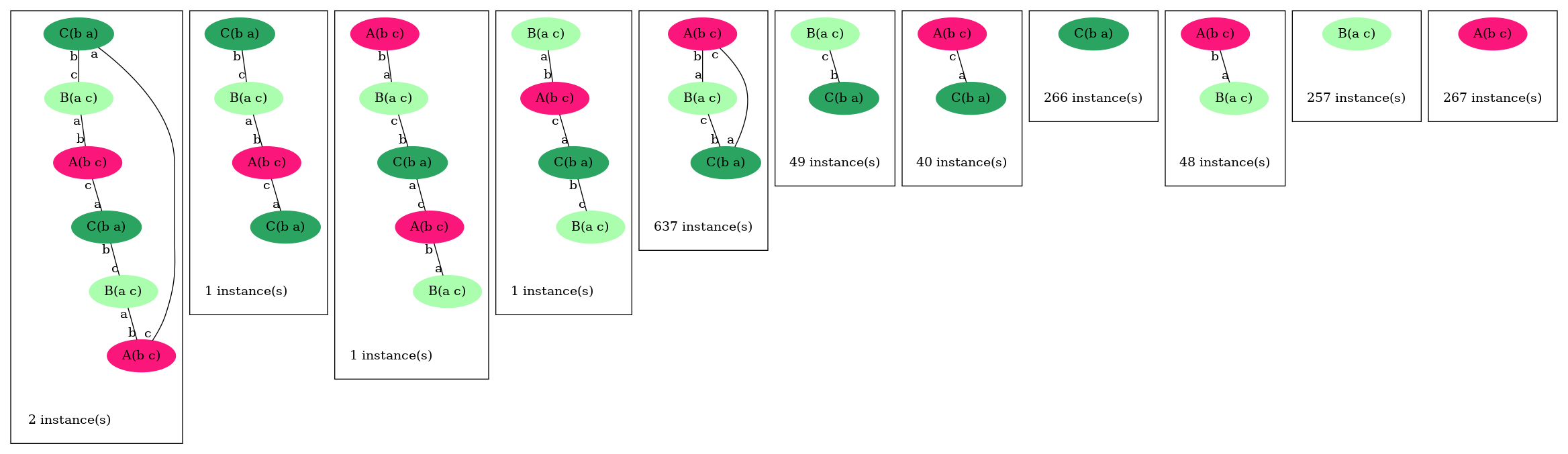Figure 2.1: Final mixture of simulation of the poly.ka model. The inﬁnite rate for cycle closure allows one to obtain a large number of triangles. For rules with unary rates, one can also specify a horizon. For example in the following rule: 1a.b A(b[.]),B(a[.]) -> A(b),B(a) @ k2{k1:5} the unary rate is applied only when the agents $A$ and $B$ are at a horizon $5$ (or closer), of each other. Horizon is an algebraic expression. It is always truncated to a positive integer during simulation. This feature can change in the future. Table 2.5: Rate expressions.  rate_expression ::= algebraic_expression | algebraic_expression {algebraic_expression:algebraic_expression} #### 2.5.5 Hybrid rules In Kappa, there can be a special treatment of entities that cannot bind anything: tokens. Tokens can only appear or disappear, they are typically used to represent small particles such as ions, ATP, etc. Tokens may have a continuous concentration. Token signatures are declared using a statement of the form: 1%token: ca+ # Signature of calcium token It is possible to mix agents and tokens in hybrid rules (which may also be bi-directional). A hybrid rule has the following form: • Kappa_expression | token_expression $\to$ Kappa_expression | token_expression @ rate Token expressions follow the grammar in Table 2.6. Table 2.6: Token expressions.  token_expression ::= algebraic_expression token_name | token_expression , token_expression token_name ::= Id Using Kappa hybrid rules, one may declare that an action has eﬀects on the concentration of some particles of the system. For instance, a rule may consume atp, calcium ions, etc. It would be a waste of memory and time to use discrete agents to represent such particles. Instead one may declare tokens using declarations of the form: 1%token: atp 2%token: adp One may then use these tokens in conjunction with a classical rule using the hybrid format: 1hybridrule S(x{u}),K(y) | 0.2 atp -> S(x{p}[.]),K(y[.]) | 0.1 adp @ k When applied, the above rule will consume 0.2 atp token and produce 0.1 adp token. Note that as speciﬁed by the grammar given in Table 2.6, the number of consumed (and produced) tokens can be given by a sum of the form: • lhs | a${}_{1}$ t${}_{1}$, ..., a${}_{n}$ t${}_{n}$ $\to$ rhs | a${}_{1}^{\prime }$ t${}_{1}^{\prime }$, ..., a${}_{k}^{\prime }$ t${}_{k}^{\prime }$ @ r where each ${a}_{i},{a}_{i}^{\prime }$ is an arbitrary algebraic expression (e.g. see Table 2.2) and each ${t}_{i},{t}_{i}^{\prime }$ is a declared token. In the above hybrid rule, denoting by ${n}_{i},{n}_{i}^{\prime }$ the respective evaluation of ${a}_{i}$ and ${a}_{i}^{\prime }$, the concentration of token ${t}_{i}$ will decrease from ${n}_{i}$ and the concentration of token ${t}_{i}^{\prime }$ will increase from ${n}_{i}^{\prime }$. Importantly, the activity of a hybrid rule is still deﬁned by |lhs|*r, where |lhs| is the number of embeddings of the LHS of the rule in the mixture, and does not take into account the concentration of the tokens it mentions. It is however possible to make its rate explicitly depend on the concentrations of the tokens using a variable rate. Consuming $t$ tokens is strickly equivalent to producing $-t$ tokens. All variations in amount of tokens can be written on the RHS of rules. This is what is done in edit notation when tokens are used: 1hybridrule S(x~u/~p!1/),K(y!1/) | (-0.1) atp @ k The variations make clear that the simulator does not check that the consumed amount of token is available. It consumes tokens even if the quantity becomes then negative! ### 2.6 Initial conditions The initial mixture to which rules in the KF will be applied are declared as follows: • %init: algebraic_expression Kappa_expression or: • %init: algebraic_expression token_name where algebraic_expression is evaluated before initialization of the simulation (hence all token and Kappa expression values in the expression are evaluated to 0). This will add to the initial state of the model multiple copies of the graph described by the Kappa expression. The DCDW convention allows us not to write the complete interface of added agents (the remaining sites will be completed according to the agent’s signature). For instance: 1%var: n 1000 2%init: n A(),A(y{p}) 3%init: 0.39 ca2+ //mM will add 1000 instances of A in its default state A(x[.],y{u}[.],z{e0}[.]), 1000 instances of A in state A(x[.],y{p}[.],z{e0}[.]) and a concentration of 0.39 mM of calcium ions. Recall that the concentration of calcium can be observed during simulation by using the expression |ca2+|. As any other declaration, %init can be used multiple times, and agents will add up to the initial state. ### 2.7 Intervention language Getting something out of a model is done like in lab experiment through intervention. Each intervention directive is splitted in 4 parts: clock When trying to intervene should be considered condition what is the condition under which the intervention is triggered intervention what are the interventions repeatition if the intervention is triggered, under what condition should it be still tried afterward There are 3 categories of intervention: modiﬁcation where the model is changed at the time of trigger (a special rule applied, a variable changed, the simulation stopped) immediate measurement where a measure is taken at the time of trigger (value of %plot printed, the current status of the mixture output, ...) continuous measurement switch where you start or stop a measurement at time of trigger They are all described in detail below. #### 2.7.1 directive syntax The general syntax is • %mod: alarm ﬂoat boolean_expression do eﬀect_list repeat boolean_expression for • %mod: clock condition do intervention repeat repeatition and syntactic sugar detailed below is provided. Boolean_expression and eﬀect_list are deﬁned by the grammar given in Table 2.7 (the operator rel can be any usual binary relation in $\left\{<,=,>\right\}$ and algebraic expressions are deﬁned in Table 2.2). Table 2.7: Intervention expressions.  boolean_expression ::= algebraic_expression rel algebraic_expression | (boolean_expression || boolean_expression) | (boolean_expression && boolean_expression) | [not] boolean_expression | [true] | [false] eﬀect_list ::= eﬀect ; eﬀect_list | eﬀect eﬀect ::=$ADD algebraic_expression agent_expression | $DEL algebraic_expression agent_expression | token_name <- algebraic_expression |$SNAPSHOT string_expression | $STOP string_expression |$DIN string_expression boolean | $TRACK 'var_name' boolean |$UPDATE 'var_name' algebraic_expression | $PLOTENTRY |$PRINT string_expression | $SPECIES_OFF string_expression Kappa_expression boolean string_expression ::= $𝜀$ | "string" . string_expression | algebraic_expression . string_expression boolean ::= [true] | [false] There are 2 kind of clocks, an event based one and simulation time ones. The event based one has the empty string for syntax: if nothing is written for clock, it uses the event based clock. It ﬁres at the beginning of the simulation and every time a rule has just ﬁred. The time based one syntax is alarm ﬂoat, it ﬁres every amount of time unit given by the ﬂoat (including at [T]=0). For example %mod: alarm 2.3 [true] do$PLOTENTRY; repeat [true] will print a line in the data ﬁle every 2.3 time unit of simulation whereas %mod: |A()| > 1000 do $PLOTENTRY; repeat |B(x[_])| < |B(x[.])| will do it every event where there is more than 1000 A up to the ﬁrst event where (there is more than 1000 A) and the number of |B(x[_])| becomes bigger than the number of |B(x[.])|. When the conditions of several interventions that are tested at the same moment are satisﬁed simultanously, interventions are triggered in the order in which they have been declared in the KF. A intervention can only be ﬁred once per event loop. #### 2.7.2 shortcuts If the repeat keyword and the repeat condition are ommited, it is assumed that it is repeat [false] aka a one-shot intervention. If the (pre)condition is ommited, it is considerated to be [true] unless both conditions are ommited and a clock is provided. In this case the implicit condition is [T] > 0 so that %mod: alarm 5.8 do effects is somehow a ’at’ operator. It means “do effects at [T] = 5.8”. #### 2.7.3 Model modiﬁcation ##### Applying a rule during a simulation Special cases: simply adding or deleting agents Continuing with the ABC model, the intervention eﬀect:$ADD n C(x1~p) will add $n\ge 0$ instances of C with x1 already in state p (and the rest of its interface in the default state as speciﬁed line 4 of ABC.ka). Also the intervention eﬀect: $DEL |B(x!_)| B(x!_) will remove all Bs connected to some agent from the mixture. There are various ways one can use interventions to study more deeply a given Kappa model. A basic illustration is the use of a simple intervention to let a system equilibrate before starting a real simulation. For instance, as can be seen from the curve given in Fig. 1.1, the number of AB complexes is arbitrarily set to 0 in the initial state (all As are disconnected from Bs in the initial mixture). In order to avoid this, one can modify the Kappa ﬁle in the following way: one sets the initial concentration of C to 0 by deleting line 22. Now one introduces Cs after 25 t.u using the intervention: %mod: [T]=25 do$ADD 10000 C();.

The modiﬁed Kappa ﬁle is available in the source repository, in the model/ directory (ﬁle abc-pert.ka). Run a simulation again (a bit longer) by entering in the command line:

• KaSim ABC-pert.ka -l 300 -p 0.3 -o abc2.out

one obtains the curve given in Fig. 2.2.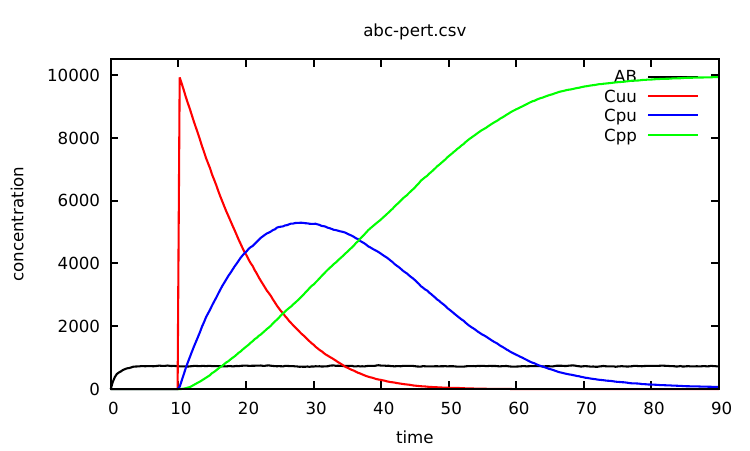Figure 2.2: Simulation of the ABC model with a intervention: for t<25s, only 'a.b' and 'a..b' rules may apply. This enables the concentration of 'AB' complexes to go to steady state, before introducing fresh Cs at t=25s.

The intervention $STOP "final_state.ka" will in addition produce a snapshot of the last mixture. #### 2.7.4 Model immediate examination Advanced and/or experimental examinations are listed in chapter 5. ##### Get a snapshot of the mixture A snapshot is an instant photography of the current state of the mixture (a dump of the state at a given moment in the simulation). A snapshot is suitable as an initial condition for a model. In the previous example, we let the system evolve for some time without its main reactant C in order to let other reactants go to a less arbitrary initial state. One may object that this way of proceeding is CPU-time consuming if one has to do this at each simulation. An alternative is to use the$SNAPSHOT primitive that allows a user to export a snapshot of the mixture at a given time point as a new (piece of) Kappa ﬁle. For instance, the declaration: %mod: [E-]/([E]+[E-])>0.9 do $SNAPSHOT "prefix"; will ask KaSim to export the mixture the ﬁrst time the percentage of null events reaches 90%. The exported ﬁle will be named prefix_$n$.ka where $n$ is the event number at which the snapshot was taken. One may also use a string_expression to construct any preﬁx using local variables. One may omit to deﬁne a preﬁx and simply type: %mod: [E-]/([E]+[E-])>0.9 do$SNAPSHOT; in which case the default preﬁx snap.ka will be used for naming snapshots.

If the name already exists, a counter will be appended at the end of the ﬁle to prevent overwriting. Snapshots can be performed multiple times, for instance every 1,000 events, using the declaration:

1%mod: ([E] [mod] 1000)=0 do $SNAPSHOT "abc.ka"; repeat [true] which results in KaSim producing a snapshot every 1000 (productive) events until the simulation ends. Note that instead of producing Kappa ﬁles, one may use snapshot interventions to produce an image of the mixture in the dot/html format using the parameter by specifying the extention in the name skeleton (%mod: [E-]/([E]+[E-])>0.9 do$SNAPSHOT "snap.dot";).

##### Printing values during a simulation

The eﬀect $PRINT string_expression >string_expression enables one to output values during a computation to: • standard output if the second string_expression and the > are ommited, • to the ﬁle speciﬁed by the second string_expression otherwise. For instance: 1%mod: |A|<0 do 2$PRINT ("Token A is: " . |A| . " at time=". [T]) > ("token_".[E].".dat");
3    repeat [true]

will ask KaSim to output the value of token A in a ﬁle "token_$n$.dat" which changes at each new productive event, each time its value gets below 0.

##### Add an entry in the output data

The eﬀect $PLOTENTRY outputs a line with the current value of observables in the data ﬁle. For example, %mod: repeat [E] [mod] 10 = 0 do$PLOTENTRY; until [false] will store the value of observables every 10 productive events.

## Chapter 3The Kappa tools

### 3.1 The KaSim engine

KaSim is a stochastic simulator of rule-based models  written in Kappa. KaSim takes one or several Kappa ﬁles as input and generates stochastic trajectories of various observables. KaSim implements Danos et al’s implicit state simulation algorithm  which adapts Gillespie’s algorithm  to rule-based models.

A simulation event corresponds to the application of a rewriting rule, contained in the Kappa ﬁles, to the current graph (also called a mixture). At each step, the next event is selected with a probability which is proportional to the rate of the rule it is an event of. If there are no events, i.e. if none of the rules apply to the current state of the system, one has a deadlock. Note that a given rule will in general apply in many diﬀerent ways; one says it has many instances. The activity of a rule is the number of its instances in the current mixture multiplied by its rate. The probability that the next event is associated to a given rule is therefore proportional to the activity of the rule. Rule activities are updated at each step (see Fig. 3.1). Importantly, the cost of a simulation event is bounded by a constant that is independent of the size of the graph it is applied to .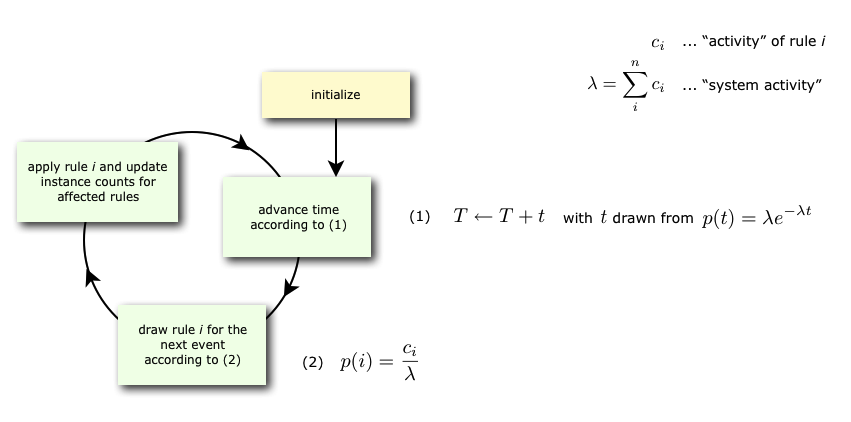Figure 3.1: The event loop.

### 3.2 The KaSa static analyser

KaSa is a static analyser tool of rule-based models  written in Kappa. KaSa takes one or several Kappa ﬁles as input and some command line options to toggle on/oﬀ some speciﬁc static analysis. Currently, KaSa can compute the contact map and the inﬂuence map. It can perform reachability analysis  as well.

A graphical interface is proposed to navigate through the various options and utilities of KaSa. The compilation of this interface requires labltk and, in particular, tk-dev.

### 3.3 The KaDE ODEs generator

KaDE is a tool to compile rule-based models  written in Kappa into systems of ordinary diﬀerential equations, or equivalently into reaction networks. It also supports some model reduction techniques, that may reduce the dimension of the ODEs (or the number of diﬀerent bio-molecular species in reaction networks).

KaDE takes one or several Kappa ﬁles and uses some command line options in order to select a backend format, tune the semantics, and call some model reduction methods.

A graphical interface is proposed to navigate through the various options and utilities of KaDE. The compilation of this interface requires labltk and, in particular, tk-dev.

## Chapter 4The stochastic simulator: KaSim

### 4.1 General usage

From a terminal window, KaSim can be invoked by typing:

• KaSim file_1 ... file_n [option]

where file_i are the input Kappa ﬁles containing the rules, initial conditions and observables (e.g. see Chapter 2). A simulation can generate several ﬁles that are described in the present chapter. One should really take advantage of the option -d so that these ﬁles all ends in a distinct directory.

In any case, a log called inputs.ka is generated. This is a valid Kappa ﬁle such that KaSim inputs.ka reruns exactly the simulation just ran outputing the exact same outputs (using the same pseudo random numbers!). First line of this ﬁle contains an uuid that is also present in any ﬁle output during the same run.

Tables 4.1 and 4.2 summarize all the options that can be given to the simulator.

Basically, one can specify an upper bound and a plot period either in simulated or bio-time (arbitrary time unit), or in number of events. Note that bio-time is computed using Gillespie's formula for time advance (see Fig. 3.1) and should not be confused with CPU-time (it is not even proportional).

### 4.2 Main options

Table 4.1 summarizes the main options that are accessible through the command line. Options that expect an argument are preceded by a single dash, options that do not need any argument start with a double dash.

Two key options are the plot period -p (how often you want a line in the data ﬁle) and the limit -l of simulation. These quantities can expressed in simulated time (the default) or in number of event (using -u event).

Table 4.1: Command line: main options.
 Argument Description -u $unit$ Unit of options (time/event) -l $max$ Terminates simulation after $max\ge 0$ $unit$ -initial $min$ Starts the simulation at $min$ $unit$ (data outputs convienience only) -p $x$ Plot a line in the data ﬁle every $x$ $unit$ -o ﬁle Set the name of data ﬁle to ﬁle Use the extension to determine format (’.tsv’, ’.svg’ or csv else) -i ﬁle Interpret ﬁle as an input ﬁle name (for compatibility with KaSim<= 3 and ﬁle names starting by -) -d dir Output any produced ﬁle to the directory dir

Table 4.2 summarizes the advanced options that are accessible through the command line.

Table 4.2: Command line: advanced options.
 Argument Description -rescale $r$ Multiply each initial quantity by $r$ --no-log Do not generate a reproductability ﬁle -log file Specify the name of the reproductability ﬁle (default “inputs”) -seed $n$ Seeds the pseudo-random number generator $n>0$ -make-sim sim_ﬁle Makes a simulation package out of the input KF -load-sim sim_ﬁle Use simulation package sim_ﬁle as input --gluttony Simulation mode that is memory intensive but that speeds up simulation time -mode batch Set non interactive mode (never halt waiting for an user action but assume default (data loosing) answer) -mode interactive Launch the toplevel just after model initialisation

### 4.4 Example

The command:

• KaSim model.ka -u event -l 1000000 -p 1000 -o model.out

will generate a ﬁle model.out containing the trajectories of the observables deﬁned in the Kappa ﬁle model.ka. A measure will be taken every 1000 events in ﬁle model.out. The command:

• KaSim init.ka rules.ka obs.ka mod.ka -l 1.5 -p 0.0015

will generate a ﬁle data.csv (default name) containing 1,000 data points of a simulation of 1.5 (arbitrary) time units of the model. The input Kappa ﬁle is split into four ﬁles containing, for instance, the initial conditions, init.ka, the rule set, rules.ka, the observables, obs.ka, and the interventions, pert.ka (e.g. see Chapter 2).

### 4.5 Interactivity

Simulations are interruptible by sending a SIGINT to the simulator. (The easiest way to send a SIGINT to a process is to press Ctrl-c in the terminal window it runs into.)

In batch mode, this stops the simulation. In other circumstances, it launches a toplevel in which one can either type:

• $RUN (optionally followed by a pause condition) to resume simulation or • any of the eﬀects described in Section 2.7 to trigger it imediately. A pause condition is a boolean expression (e.g. Section 2.7) under which the simulator will stop and fall back in the toplevel in order to allow a new interactive session. The option -mode interactive interrupts automatically the simulation (and launches the toplevel) just after the initialization of the simulation. ## Chapter 5Advanced concepts ### 5.1 Experimental continuous examination #### 5.1.1 Causality analysis In our ABC example, adding the instruction: %mod: [true] do$TRACK Cpp[true]; will ask KaSim to turn on causality analysis for the observable 'Cpp' since the beginning of the simulation, and display the causal explanation of every new occurrence of 'Cpp', until the end of the simulation. The explanation, that we call a causal ﬂow, is a set of rule applications ordered by causality and displayed as a graph using dot format. In this graph, an edge r$\to$ r' between two rule applications r and r' indicates that the ﬁrst rule application has used, in the simulation, some sites that were modiﬁed by the application of the former. We show in Fig. 5.1 an example of such causal ﬂow.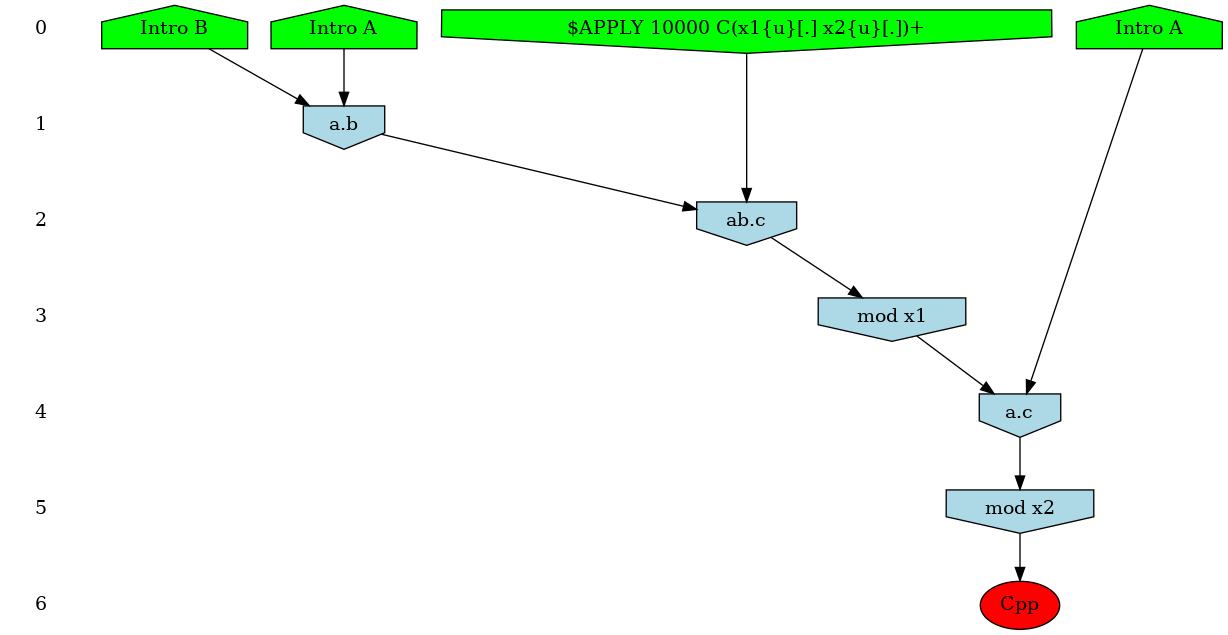Figure 5.1: Causal ﬂow for the observable 'Cpp' of the ABC model. Plain arrows represent causal dependency, dotted arrows show asymmetric conﬂicts between rule occurrences. Here the 'ab.c' rule has to occur before the 'a.b' rule. The red observable indicates that the last rule allowed one to observe a new instance of 'Cpp'.

Causality analysis of the observable Cpp can be turned oﬀ at any time by using a declaration of the form: %mod: [T]>25 do $TRACK Cpp[false]; Each time KaSim detects a new occurrence of the observable that is being tracked, it will dump its causal past as a graph using the dot format (see Fig. 5.1 above). The name of the ﬁle in which the causal ﬂow is stored can be set by using the %def instruction (see Section 5.4). ##### Compressing causal ﬂows. In general, pure causal ﬂows will contain a lot of information that modelers may not wish to consider. Indeed in classical ﬂows, causality (represented by an edge between to rule applications in the graph) is purely local. Therefore a sequence $a\to b\to c$ only implies that an instance of rule $a$ caused an instance of rule $b$ which in turn created an instance of the observable $c$. However, it does not imply that $a$ was "necessary" for $c$ to occur (for instance, $c$ might have been possible before $a$ but not after, and $b$ would be simply re-enabling $c$). It is possible to tell KaSim to retain only events that are more strongly related to the observable using two compression techniques (see Ref.  for formal details). Intuitively, in a weakly compressed causal ﬂow one has the additional property that if an event $e$ is a (possibly indirect) cause of the observable, then preventing $e$ from occurring would have prevented the rest of the causal ﬂow to occur (i.e. it is not possible to reconstruct a computation trace containing the observable with the events that remain in the causal ﬂow). A strongly compressed causal ﬂow enjoys the same property with an additional level of compression obtained by considering diﬀerent instances of the same rule to be indistinguishable. Note that, causal ﬂow compressions may be memory and computation demanding. For large systems it may be safer to start with weak compressions only. The type of compression can be set using the %def instruction (see Section 5.4). For instance: %def: "displayCompression" "none" "weak" "strong" will ask KaSim to output 3 versions of each computed causal ﬂow, with all possible degrees of compressions. Each causal ﬂow is outputted into a ﬁle [filename][Type]_$n$.dot where filename is the default name for causal ﬂows which can be redeﬁned using the parameter cflowFileName, Type is the type of compression (either nothing or Strongly, or Weakly) and $n$ is the identiﬁer of the causal ﬂow. For each compression type a summary ﬁle, named [filename][Type]Summary.dat, is also produced. It allows to map each compressed causal ﬂow to the identiﬁer of its uncompressed version (row #id), together with the production time $T$ and event number $E$ at which the observable was produced. It also contains information about the size of the causal ﬂow. ##### Limit the number of ﬂows As an example, consider the computation of causal ﬂows between $t=10$ and $t=20$ using the declarations: 1%mod: [T]>10 do$TRACK Cpp [true];
2%mod: [T]>20 do $TRACK Cpp [false]; The above declaration will ask KaSim to analyze each new occurrence of 'Cpp' in that time interval. If $n$ new instances took place, then KaSim will have to compute $n$ causal ﬂows. One may want to bound the number of computed ﬂows to a certain value, say 10. One may do so using the combination of interventions and variables given below: 1%var: x 0 2%mod: [T]>10 do ($TRACK Cpp [true] ; $UPDATE x Cpp) 3%mod: [T]>20 || (x > 0 && Cpp - x > 9) do$TRACK Cpp [false];

The ﬁrst line is a declaration of an $x$ variable that is initially set to 0. Note that, the second line is a intervention that contains two simultaneous eﬀects, the ﬁrst one triggering causality analysis and the second one updating the value of variable $x$ to the current value of variable 'Cpp'. The last line stops causality analysis whenever time is greater than 20 or when 10 new observables have been found (the diﬀerence between the current value of 'Cpp' and $x$).

#### 5.1.2 Dynamic inﬂuence network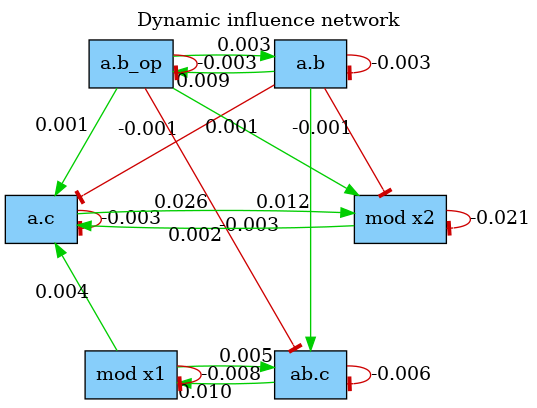Figure 5.2: Dynamic inﬂuence network of the abc.ka model, taken from t=0 to t=20 time units. The A releasing rules a..b and mod x2 are contributing very little to the activity of a.c which is a sign of an excess of free As in the system at this time interval.

The dynamic inﬂuence network (DIN) is a powerful observation that tracks, on the ﬂy, the inﬂuence that rule applications have on each others. It is dynamically generated and tracks eﬀective impacts (positive or negative) at every rule application. The DIN can be computed using declarations of the form:

1%mod: [true] do $DIN "flux.dot" [true]; 2%mod: [T]>20 do$DIN "flux.dot" [false];

The result is a graph where a positive edge between rules $r$ and $s$ (in green) indicates an overall positive contribution of $r$ over $s$. Otherwise, the sum of $r$ applications increased the activity of $s$. Conversely, a negative edge (in red) will indicate that $r$ had an overall negative impact on the activity of $s$. Note that, the importance of the inﬂuence between two rules can be observed by looking at the label on the edges that indicate the overall activity transfer (positive or negative) between the rules. The above declaration produces the network shown in Fig. 5.2. Note that, inﬂuence may vary during time, therefore the time or event limit of the simulation is of importance and will likely change the aspect of the produced map.

#### 5.1.3 Print species of an observable

The eﬀect $SPECIES_OFF tracks the occurrence of an observable. Each time a new instance of the observable appears, the species in which it occured is printed in a ﬁle. For example: 1%mod:$SPECIES_OF "species.ka" A(a!1), B(a!1) [true];

prints in the ﬁle "species.ka" a new line for each new occurrence of A(a!1), B(a!1) with the time of occurrence and the species in which it occurred.

Note that, this intervention can only be applied if the observable is a connected component.

### 5.2 Implicit signature

KaSim permits users in a hurry to avoid writing agent signatures explicitly using the option --implicit-signature of the command line. The signature is then deduced using information gathered in the KF. Note that, it is not recommended to use the DCDW convention for introduced agents in conjunction with the --implicit-signature option unless the default state of all sites is mentioned in the %init declarations or in the rules that create agents.

### 5.3 Simulation packages

The simulation algorithm that is implemented in KaSim requires an initialization phase whose complexity is proportional to $R\ast G$ where $R$ is the cardinal of the rule set and $G$ is the size of the initial mixture. Thus for large systems, initialization may take a while. Whenever a user wishes to run several simulations of the same Kappa model, it is possible to skip this initialization phase by creating a simulation package. For instance:

• KaSim abc.ka -l $n$ -make-sim abc.kasim

will generate a standard simulation of the abc.ka model, but in addition, will create the simulation package abc.kasim (.kasim extension is not mandatory). This package is a binary ﬁle, i.e. not human readable, that can be used as input of a new simulation using the command:

• KaSim -load-sim abc.kasim -l $k$

Note that this simulation is now run for $k$ time units instead of $n$. Importantly, simulation packages can only be given as input to the same KaSim that produced it. As a consequence, recompiling the code, or obtaining diﬀerent binaries, will cause the simulation package to become useless.

### 5.4 Simulation parameters conﬁguration

In the KF (usually in a dedicated ﬁle) one may use expressions of the form:

%def: "parameter_name" "parameter_value"

where tunable parameters are described in table 5.1 (default values are given ﬁrst in the possible values column).

Table 5.1: User deﬁned parameters.
 parameter possible values description Simulation "maxConsecutiveClash" "2" or any integer number of consecutive clashes before giving up square approximation "T0" ﬂoat simulation starting time (outputs convienience only) "seed" any positive integer pseudo-random number generator seed Outputs "traceFileName" string outputs simulation trace in the given ﬁle "outputFileName" string data ﬁle name "plotPeriod" number then optionally interval between "events" plot lines Causality analysis deprecated please use KaStor "displayCompression" any combination of type of compression "none", "strong", "weak" "cflowFileName" "cflow", any string ﬁle name preﬁx for causal ﬂows "dotCflows" "no", "html" generate causal ﬂows in html "yes", "dot" generate causal ﬂows in dot "json" generate causal ﬂows in json Pretty printing "dumpIfDeadlocked" "no","yes" snapshot when simulation is stalled "colorDot" "no", "yes" use colors in dot format ﬁles "progressBarSymbol" "#" or any character symbol for the progress bar "progressBarSize" "60" or any integer length of the progress bar

### 5.5 Counters

An agent can have counters, which are sites storing a positive integer. When declaring an agent, each counter has to be assigned a min and a max value. For instance, the agent %agent: A(x,c:1 += 4,d:0 +=2) has two counters, named $c$ and $d$, which range from $1$ to $4$, and from $0$ to $2$, respectively.

Table 5.2: Agent signature with counters extends in Table ??
 signature_expression ::= Id(sig) sig ::= Id internal_state_list, sig $\mid$ Id counter_test counter_modif , sig $\mid 𝜀$

At initialisation, an initial value for the counter has to be speciﬁed.

In rules, all counter tests have to be in the LHS, while the counter modiﬁcations in the RHS. A counter test can do three things: (i) check that the counter value is equal to a positive integer; (ii) check that the counter’s value is greater than a positive integer or (iii) declare a variable, to which the counter’s value is assigned. The variable can then be used in the rate constant.

A counter modiﬁcation increments or decrements the original counter value. In the following table, $n\le 0$ and both $n$ and $i$ are integers.

Table 5.3: Counter expressions
 counter_expression ::= Id counter_test counter_modif $\mid 𝜀$ counter_test ::= : n $\mid$ :> n $\mid$ :> variable $\mid 𝜀$ counter_modif ::= : i $\mid 𝜀$

If the counter goes negative, the compilation stops with an error. If the counter goes up beyond its declared maximum value, the simulation stops with an error.

## Chapter 6The KaSa static analyser

### 6.1 General usage

From a terminal window, KaSa can be invoked by typing the following command line:

• KaSa file_1 ... file_n [option]

where file_i are the input Kappa ﬁles containing the rules, initial conditions and observables (see Chapter 2).

All the options are summarised as follows:

General options
--help            Verbose help
-h               Short help
--version         Show version number
--gui             GUI to select
--(no-)expert     Expert mode (more options)

Actions
--do-all
launch everything
--reset-all
launch nothing
--(no-)compute-contact-map    (default: enabled)
compute the contact map
--(no-)compute-influence-map    (default: enabled)
compute the influence map
--(no-)compute-ODE-flow-of-information    (default: disabled)
Compute an approximation of the flow of information in the ODE
semantics
--(no-)compute-potential-cycles    (default: disabled)
Compute the bonds that may be involved in polymerisation
--(no-)compute-stochastic-flow-of-information    (default: disabled)
Compute an approximation of the flow of information in the stochastic
semantics
--(no-)compute-reachability-analysis    (default: enabled)
Compute an approximation of the states of agent sites
--(no-)compute-symmetries    (default: disabled)
Look up for pairs of symmetric sites
--(no-)compute-local-traces    (default: disabled)
Compute the local traces of interesting parts of agent interfaces
--(no-)compute-separating-transitions    (default: disabled)
Compute the transitions that separates strongly connected set of
configurations
-syntax V3 | V4
(default: V4)
Version of the lexer/parser

Syntax
-syntax V3 | V4
(default: V4)
Version of the lexer/parser

Output
--output-directory <value>
Default repository for outputs
--output-contact-map-directory <name>   (default: output)
put the contact map file in this directory
--output-contact-map <name>   (default: contact)
file name for the contact map output
--contact-map-format DOT | GEPHI
(default: DOT)
Tune the output format for the contact map
--output-influence-map-directory <name>   (default: output)
put the influence map file in this directory
--output-influence-map <name>   (default: influence)
file name for the influence map
--influence-map-format DOT | DIM | HTML
(default: DOT)
Tune the output format for the influence map
--output-local-traces-directory <name>   (default: output)
put the files about local traces in this directory
--local-traces-format DOT | HTML
(default: DOT)
Tune the output format for the local transition systems
--output-log-directory <name>   (default: output)
put the log files in this directory

Reachability analysis
--(no-)compute-reachability-analysis    (default: enabled)
Compute an approximation of the states of agent sites
--enable-every-domain
enable every abstract domain
--disable-every-domain
disable every abstract domain
--contact-map-domain static | dynamic
(default: dynamic)
contact map domain is used to over-approximate side-effects
--(no-)views-domain    (default: enabled)
enable local views analysis
--(no-)counters-domain    (default: enabled)
enable counter analysis
--counters-accuracy non-relational | octagons | mi
(default: mi)
Abstract domain for counter analysis
--(no-)double-bonds-domain    (default: enabled)
enable double bonds analysis
--(no-)sites-across-bonds-domain    (default: enabled)
enable the analysis of the relation among the states of sites in
connected agents
--verbosity-level-for-reachability-analysis Mute | Low | Medium | High |
Full
(default: Low)
Tune the verbosity level for the reachability analysis
--output-mode-for-reachability-analysis raw | kappa | english
(default: kappa)
post-process relation and output the result in the chosen format

Trace analysis
--(no-)compute-local-traces    (default: disabled)
Compute the local traces of interesting parts of agent interfaces
--(no-)show-rule-names-in-local-traces    (default: enabled)
Annotate each transition with the name of the rules in trace
abstraction
--(no-)use-macrotransitions-in-local-traces    (default: disabled)
Use macrotransitions to get a compact trace up to change of the
interleaving order of commuting microtransitions
--(no-)ignore-trivial-losanges    (default: disabled)
Do not use macrotransitions for simplifying trivial losanges
--(no-)compute-separating-transitions    (default: disabled)
Compute the transitions that separates strongly connected set of
configurations
--output-local-traces-directory <name>   (default: output)
put the files about local traces in this directory
--local-traces-format DOT | HTML
(default: DOT)
Tune the output format for the local transition systems

Contact map
--(no-)compute-contact-map    (default: enabled)
compute the contact map
--(no-)compute-potential-cycles    (default: disabled)
Compute the bonds that may be involved in polymerisation
--output-contact-map-directory <name>   (default: output)
put the contact map file in this directory
--output-contact-map <name>   (default: contact)
file name for the contact map output
--contact-map-format DOT | GEPHI
(default: DOT)
Tune the output format for the contact map
--contact-map-accuracy-level Low | High
(default: Low)
Tune the accuracy level of the contact map
--polymer-detection-accuracy-level Low | High
(default: High)
Tune the accuracy level of the detection of polymers
--(no-)pure-contact    (default: disabled)
show in the contact map only the sites with a binding state

Influence map
--(no-)compute-influence-map    (default: enabled)
compute the influence map
--influence-map-accuracy-level Indirect | Direct | Realisable
(default: Direct)
Tune the accuracy level of the influence map
--output-influence-map-directory <name>   (default: output)
put the influence map file in this directory
--output-influence-map <name>   (default: influence)
file name for the influence map
--influence-map-format DOT | DIM | HTML
(default: DOT)
Tune the output format for the influence map

Flow of information
--(no-)compute-ODE-flow-of-information    (default: disabled)
Compute an approximation of the flow of information in the ODE
semantics
--(no-)compute-stochastic-flow-of-information    (default: disabled)
Compute an approximation of the flow of information in the stochastic
semantics

Debugging information
--output-log-directory <name>   (default: output)
put the log files in this directory
--(no-)debug    (default: disabled)
dump debugging information
--(no-)unsafe-mode    (default: enabled)
exceptions are gathered at the end of the computation, instead of
halting it
--(no-)print-efficiency    (default: disabled)
prompt CPU time and various datas

(66 options)

Order in options matters, since they can be used to toggle on/oﬀ some functionalities or to assign a value to some environment variables. The options are interpreted from left to right.

More options are available in the OCaml ﬁle KaSa_rep/config/config.ml and can be tuned before compilation.

### 6.2 Graphical interface

#### 6.2.1 Launching the interface

The graphical interface can be launched by typing the following command line:

• KaSa

without any option.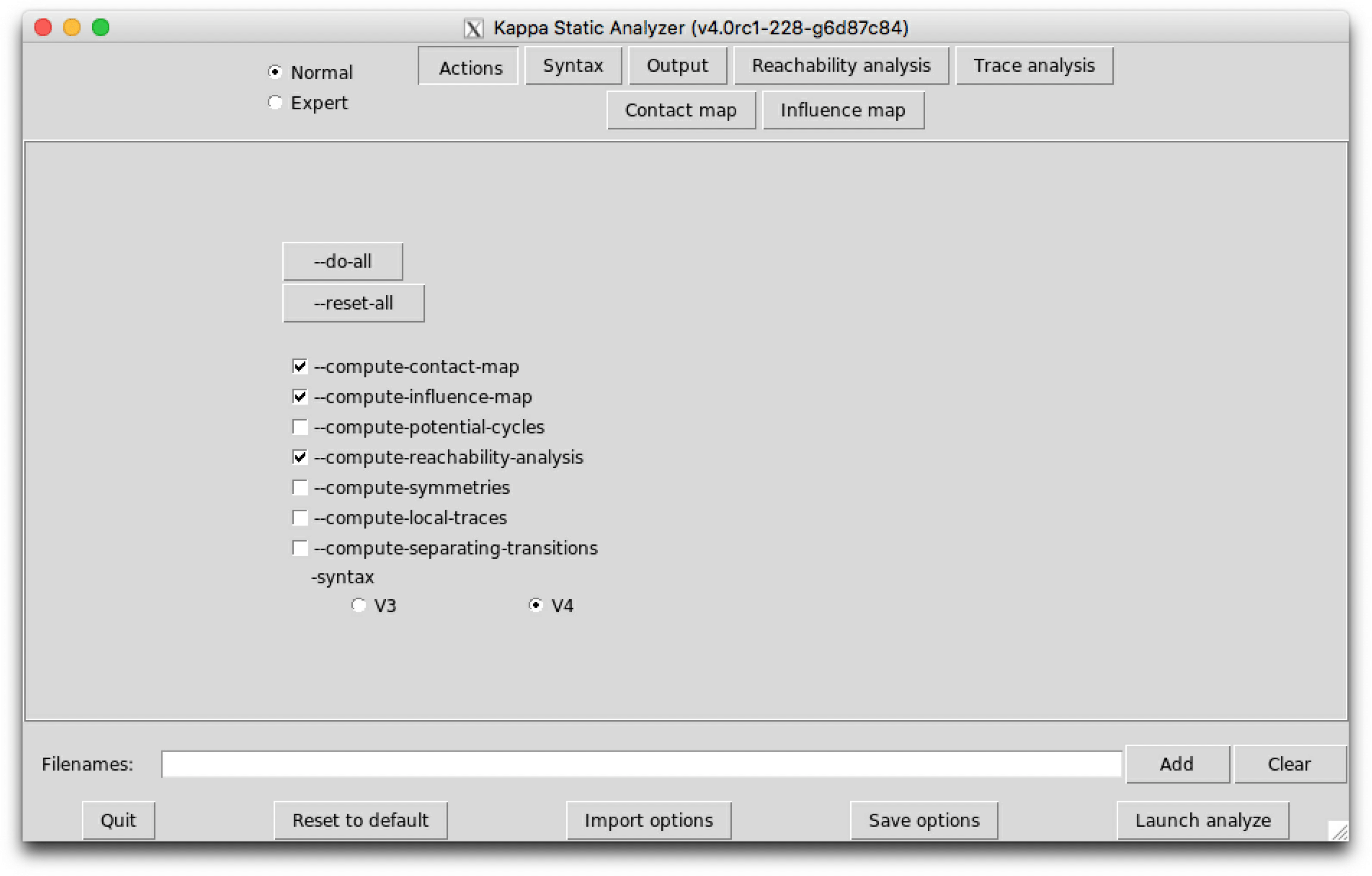Figure 6.1: KaSa  graphical interface - sub-tab Actions.

#### 6.2.2 The areas of interest

There are ﬁve diﬀerent areas of importance in the graphical interface:

1. On the top left of the window, a button allows for the selection between the Normal and the Expert mode (other modes may be available if activated at compilation). In expert mode, more options are available in the graphical interface.
2. On the top center/right, some button allows for the selection of the tab. There are currently six sub-tabs available: Actions, Syntax, Output, Reachability analysis, Trace analysis, Contact map, Influence map.
3. In the center, the options of the selected sub-tab are displayed and can be tuned.

Contextual help is provided when the mouse is hovered over an element.

The interface will store the options that are checked or ﬁlled and the order in which they have been selected. When launched, the analysis interprets these options in the order they have been entered.

Some options appear in several sub-tabs. They denote the same option and share the same value.

4. File selector: The ﬁle selector can be used to upload as many Kappa ﬁles as desired. The button ’Clear’ can be used to reset the selection of ﬁles.
5. Bottom: Some buttons are available. The button ’Quit’ can be used to leave the interface. The button ’Reset to default’ tunes all the options to their default value. The button ’Import options’ can be used to restore the value of the options as saved during a previous session of the graphical interfaces. The button ’Save options’ can be used to save the value of the options for a further session. The button ’Launch analyze’ launches KaSa with the current options.

Importantly, options are saved automatically under various occasions. Thus, it is possible to restore the value of the options before the last reset, before the last quit, or before the last analysis.

#### 6.2.3 The sub-tab Actions

The sub-tab Actions (see Fig. 6.1) contains the main actions which can be performed.

The following options are available:

• The button --do-all activates all the functionalities.
• The button --reset-all inactivates all the functionalities.
• The option --compute-contact-map can be used to (des)activate the computation of the contact map.
• The option --compute-influence-map can be used to (des)activate the computation of the inﬂuence map.
• The option --compute-potential-cycles can be used to (des)activate the computation of the potential polymers.
• The option --compute-reachability-analysis can be used to (des)activate the computation of the reachability analysis.
• The option --compute-symmetries can be used to (des)activate the computation of pairs of equivalent sites. Equivalent sites may be used to reduce the number of variables in the system of ordinary diﬀerential equations that is associated to a Kappa model (e.g. see Sect. 7.3.2).
• The option --compute-local-traces can be used to (des)activate the computation of the trace analysis.
• The option --compute-separating-transitions can be used to (des)activate the computation of the transitions that are non weakly reversible. These are the transitions between two conﬁgurations of an agent, that are impossible to revert (even in several computation steps).

Lastly, a switch allows to select the version for Kappa syntax for the input ﬁle, among version $3$, V3, and version $4$, V4.

#### 6.2.4 The sub-tab Syntax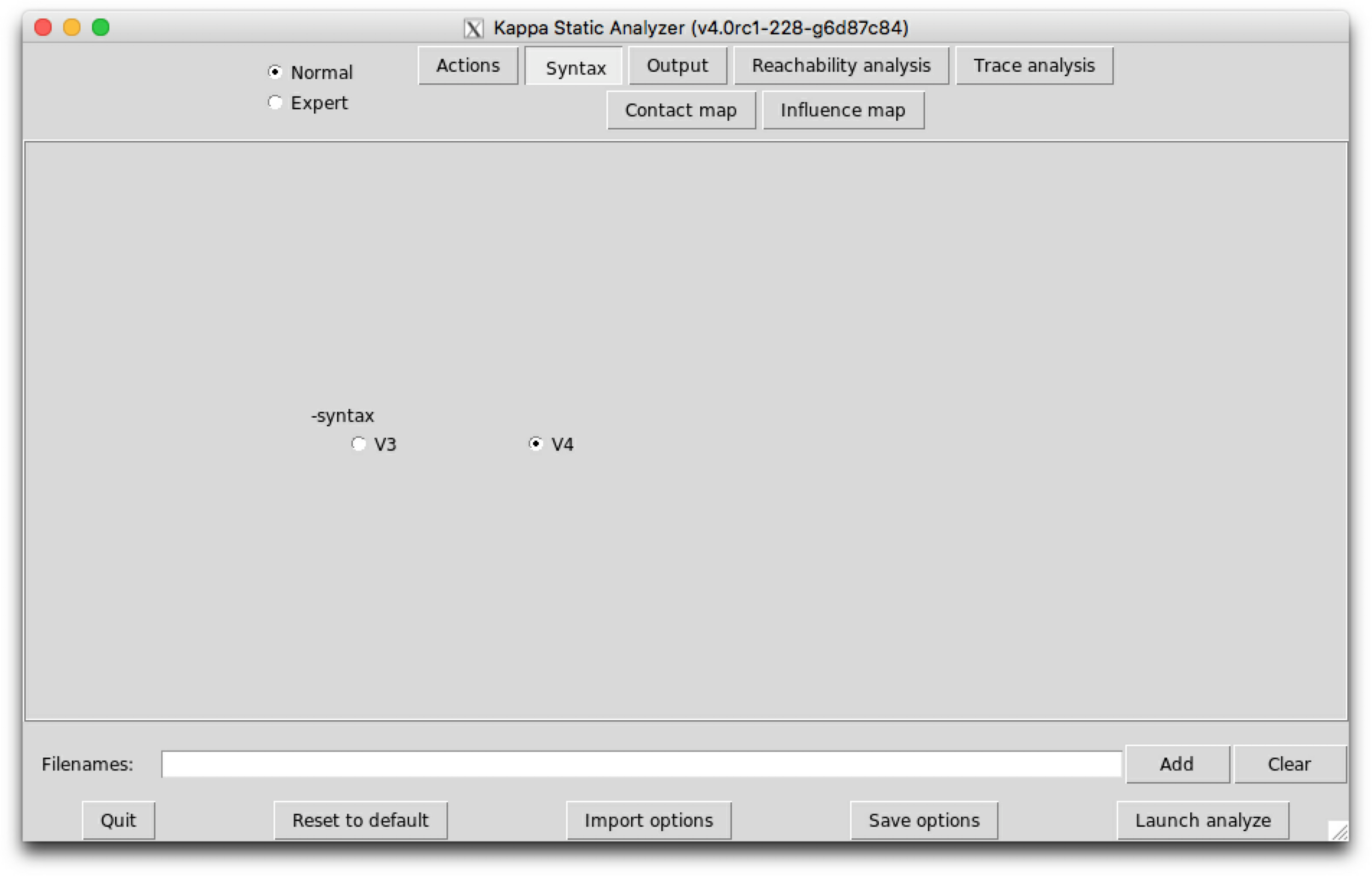Figure 6.2: KaSa  graphical interface - sub-tab Syntax.

The sub-tab Syntax (see Fig. 6.2) contains the switch to select between the version 3, V3, and the version 4, V4, of Kappa syntax.

#### 6.2.5 The sub-tab Output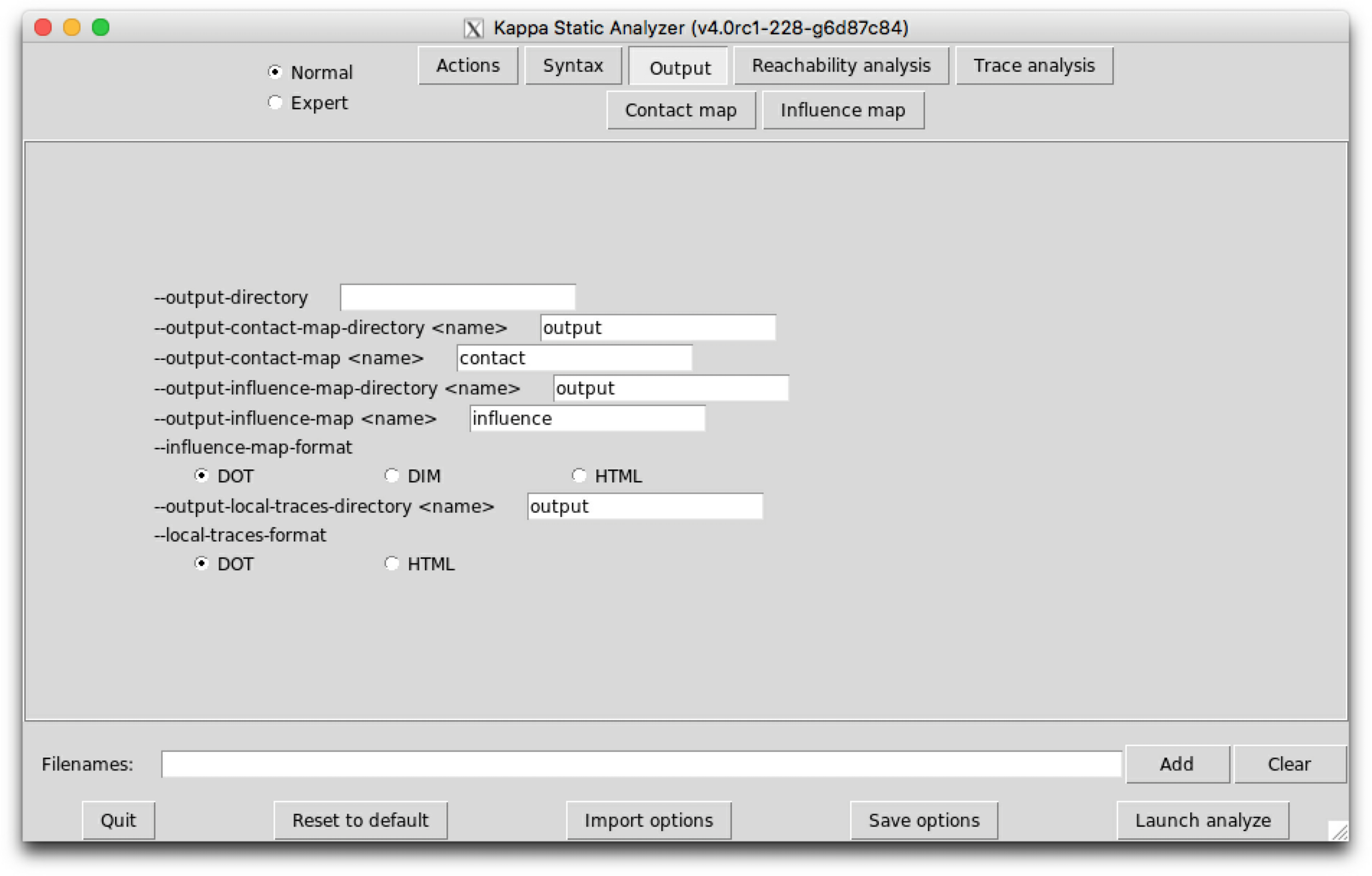Figure 6.3: KaSa  graphical interface - sub-tab output.

The sub-tab Ouput (see Fig. 6.3) contains the names of the output ﬁles and their format.

The following options are available:

• The ﬁeld --output-directory can be used to set the repository where output ﬁle are written. KaSa will create this repository, if it does not exist.
• The ﬁeld --output-contact-map-directory can be used to set the repository where the output ﬁle for the contact map is written, if a contact map is requested. KaSa will create this repository, if it does not exist.
• The ﬁeld --output-contact-map contains the name of the ﬁle for the contact map. If the ﬁle name does not end by it, the proper extension will be added to the ﬁle name.
• The ﬁeld --output-influence-map-directory can be used to set the repository where the output ﬁle for the inﬂuence map is written, if an inﬂuence map is requested. KaSa will create this repository, if it does not exist.
• The ﬁeld --output-influence-map contains the name of the ﬁle for the inﬂuence map. If the ﬁle name does not end by it, the proper extension will be added to the ﬁle name.
• The format for the inﬂuence map can be chosen among DOT, DIM, and HTML thanks to the option --influence-map-format. In format DIM, the output of the inﬂuence map is a json ﬁle containing the support of the dynamic inﬂuence map that has been introduced in Sect. 5.1.2.
• The ﬁeld --output-local-traces-directory can be used to set the repository where the output ﬁle for the result of trace analysis is written, if this analysis is requested. KaSa will create this repository, if it does not exist.
• The format for the local traces can be chosen among DOT and HTML thanks to the option --local-traces-format.

When a ﬁle already exists, it is overwritten without any warning.

### 6.3 Reachability analysis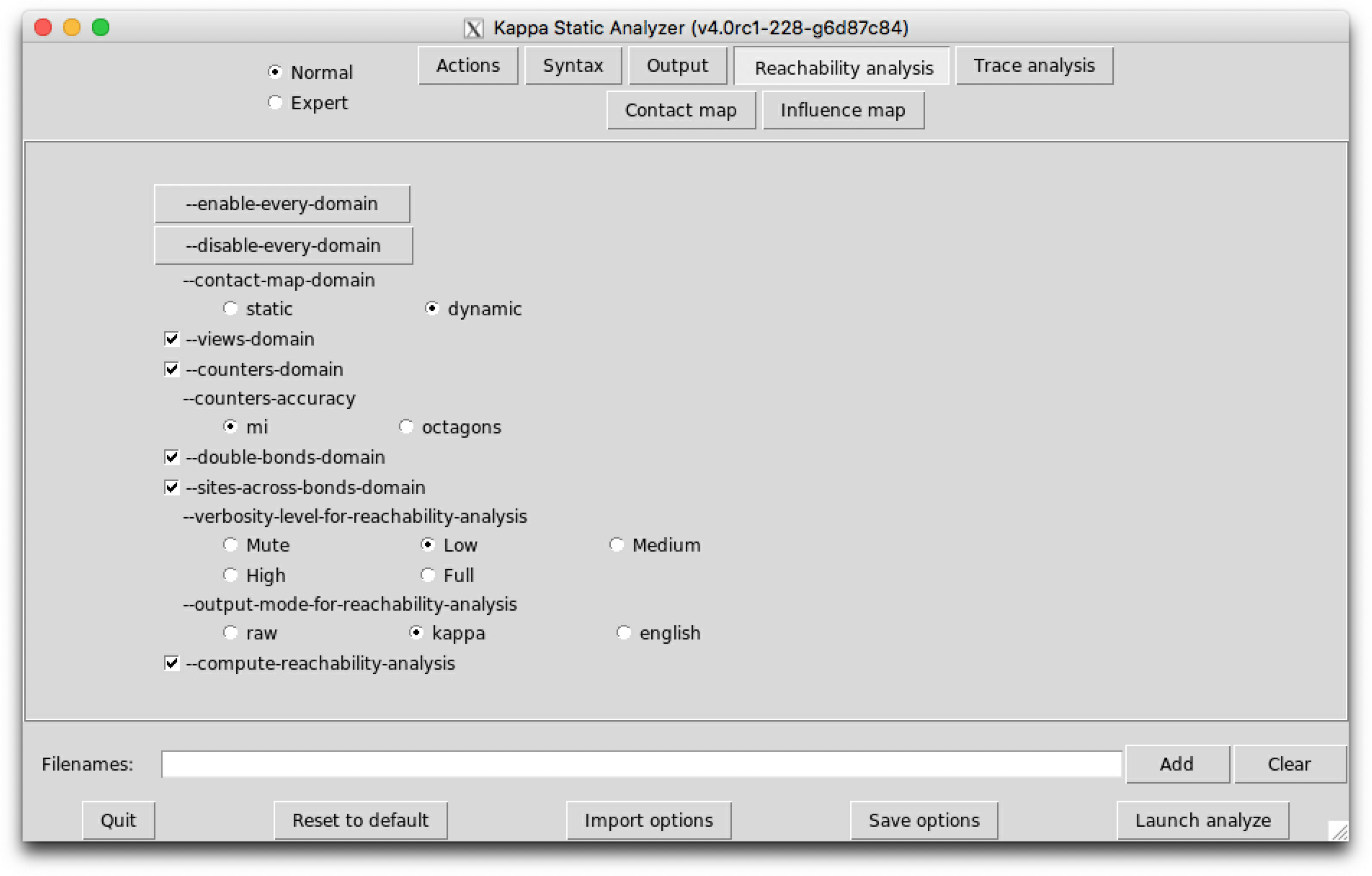Figure 6.4: KaSa  graphical interface - sub-tab Reachability_analysis.

Reachability analysis aimed at detecting statically properties about the bio-molecular species that may be formed in a model. Knowing whether, or not, a given bio-molecular species may be formed in a model is an undecidable problem . Thus, our analysis is approximate. Indeed, it computes an over-approximation of the set of the bio-molecular species that can be reached from the initial state of the model, by applying an unbounded number of computation steps. As formalized in , the abstraction consists in:

1. ﬁrstly, ignoring the number of occurrences of bio-molecular species (we assume that whenever a bio-molecular species may be formed, then it may be formed as many time as it could be necessary),
2. secondly, abstracting a bio-molecular species by the set of its properties.

The analysis takes into account also the chemical species that may be introduced in a intervention.

The classes of properties of interest are encoded in so called abstract domains, which can be independently enabled/disabled. The whole analysis can be understood as a mutual recursion between smaller analyses (one per abstract domain), that communicate information between each other at each step of the analysis. We took the same scheme of collaboration between abstract domains as in .

As an example, we consider the following model:

1%agent: E(x)
2%agent: R(x,c,cr,n)
3
4%init: 1 E()
5%init: 1 R()
6
7E.R E(x[.]),R(x[.]) -> E(x),R(x) @1
8E/R E(x),R(x,c[.]) -> E(x[.]),R(x[.],c[.]) @1
9R.R R(x[_],c[.]),R(x[_],c[.]) -> R(x[_],c),R(x[_],c) @1
10R/R R(c,cr[.],n[.]),R(c,cr[.],n[.]) -> R(c[.],cr[.],n[.]),R(c[.],cr[.],n[.]) @1
11R.int R(c,cr[.],n[.]),R(c,cr[.],n[.]) -> R(c,cr,n[.]),R(c,cr[.],n) @1
12R/int R(cr),R(n) -> R(cr[.]),R(n[.]) @1
13obs R(x[.],c[.],cr[_],n[_]) -> R(x[.],c[.],cr[.],n[.]) @1

Typing the following command line:

KaSa reachability.ka --reset-all --compute-reachability-analysis

will perform the reachability analysis on the model reachability.ka.

We obtain the following result:

Kappa Static Analyzer (v4.1-14-g897fd18) (without Tk
interface)
Analysis launched at 2021/05/07 13:01:44 (GMT+0) on
travis-job-f7a48165-7d3f-4cbd-affb-4526961426f7
Parsing ../kappa/reachability.ka...
done
Compiling...
Reachability analysis...

------------------------------------------------------------
* There are some non applyable rules
------------------------------------------------------------
rule obs (File "../kappa/reachability.ka", line 13,
characters 6-59:) will never be applied.
------------------------------------------------------------
every agent may occur in the model

------------------------------------------------------------
* Non relational properties:
------------------------------------------------------------
E(x) => [ E(x[.]) v E(x[x.R]) ]
R(c) => [ R(c[.]) v R(c[c.R]) ]
R(cr) => [ R(cr[.]) v R(cr[n.R]) ]
R(n) => [ R(n[.]) v R(n[cr.R]) ]
R(x) => [ R(x[.]) v R(x[x.E]) ]

------------------------------------------------------------
* Relational properties:
------------------------------------------------------------
R() =>
[
R(c[.],cr[.],n[.],x[x.E])
v R(c[c.R],cr[n.R],n[.],x[x.E])
v R(c[c.R],cr[.],n[.],x[x.E])
v R(c[c.R],cr[.],n[cr.R],x[x.E])
v R(c[.],cr[.],n[.],x[.])
]
------------------------------------------------------------
* Properties in connected agents
------------------------------------------------------------
R(c),R(c) =>
[
R(c,cr[n.R]),R(c,cr[.])
v R(c,cr[.]),R(c,cr[.])
v R(c,cr[.]),R(c,cr[n.R])
]
R(c),R(c) =>
[
R(c,n[cr.R]),R(c,n[.])
v R(c,n[.]),R(c,n[.])
v R(c,n[.]),R(c,n[cr.R])
]
------------------------------------------------------------
* Properties of pairs of bonds
------------------------------------------------------------
R(c[c.R],cr[n.R]) => R(c,cr),R(c,n)
R(c[c.R],n[cr.R]) => R(c,n),R(c,cr)
------------------------------------------------------------
* Properties of counters
------------------------------------------------------------
execution finished without any exception

This result is displayed in the standard output, and it is made of six parts.

The ﬁrst two parts provide an enumeration of dead rules and dead agents. The next parts display what we call reﬁnement lemmas. A reﬁnement lemma is made of a precondition (on the left of the implication symbol) that is a site graph, and a postcondition (on the right of the implication symbol) that is a list of site graphs. Each site graph in the post-condition is a reﬁnement of the precondition (the position of agent matters: the $n$-th agent in the precondition corresponds to the $n$-th agent in each site graph in the postcondition, but site graphs in a postcondition may have more agents than the site graph in the corresponding precondition). The meaning of a reﬁnement lemma is that every embedding between its precondition into a reachable state can be reﬁned/extended into an embedding from one site graph in its postcondition into the same reachable state. This way, a reﬁnement lemma provides an enumeration of all the potential contexts for the precondition.

We now detail the seven diﬀerent parts:

• Detection of dead rules. A rule is called dead, if there is no trace starting from the initial state in which this rule is applied. The analysis reports the list of the rules it has detected to be dead. Due to the over-approximation, it may happen that a dead rule is not discovered by the analysis. Yet, every rule that is reported as dead, is dead indeed.

In our example, we notice that the rule ‘obs’ can never be trigered.

• Detection of dead agents. An agent is called dead, if there is no trace starting from the initial state with at least one state in which this agent occurs. The analysis reports the list of the agents it has detected to be dead. Due to the over-approximation, it may happen that a dead agent is not discovered by the analysis. Yet, every agent that is reported as dead, is dead indeed.

In our example, there are no dead agent.

• Non-relational properties. The analysis detects for each kind of site, the set of states this site can take. Due to the over-approximation, the analysis reports a super-set of the set of the potential states. Yet, we are sure that a given site only take states within this set.

In our example, the site cr of R may be free, or bound to the site n of an agent R.

• Relational properties. The analysis detects some relationships among the states of packs of sites within each agent, hence capturing potential valuations for local views . Due to the over-approximation of the analysis, the analysis may fail in discovering a relationship. But each relationship that is found by the analysis is satisﬁed.

In our example, the states of the sites c, cr, n, and x of R are entangled with a relational property (othewise, we would have ${5}^{2}$ elements in the post-condition).

• Properties in connected agents. When two agents are connected, there may be a relation among the states of theirs respective sites. This abstraction  collects for each kind of bonds, the relation between the state of one site in the ﬁrst agent and the state of one site in the second agent. Due to the over-approximation, the analysis reports a super-set of the set of the potential pairs of states.

This abstraction aimed at capturing information about protein transportation. It is quite common to model the location of a protein as the internal state of a ﬁctitious site. With such an encoding, it might be important to ensure that two connected proteins are always located in the same location. This abstraction focuses on this kind of properties.

• Properties of pairs of bonds.

It might be interesting to know whether a protein can be bound to another protein twice simultaneously, and whether a protein can be bound to two instances of a same protein simultaneously. This abstraction  captures this kind of constraint. It can be used to prove that some proteins do not polymerize.

In our example, when a R has its sites cr and c bound, they are necessarily bound to the same instance of R. The same statement holds for the sites cr and n.

• Properties of counters.

KaSa is inferring the range of counters.

There are no counter in our previous example. Let us consider the following one:

1%agent:A(x1{u p},x2{u p q},x3{u p},c{=0},d{=0})
2%agent: B(x)
3
4A(x1{u}) -> A(x1{p},c{+=3},d{+=2}) @1
5A(x1{p}) -> A(x1{u},c{-=3},d{-=1}) @1
6A(x2{u}) -> A(x2{p},c{+=2},d{+=1}) @1
7A(x2{p}) -> A(x2{u},c{-=2},d{-=2}) @1
8A(x2{p}) -> A(x2{q},c{+=1}) @1
9A(x2{q}) -> A(x2{p},c{-=1}) @1
10A(x3{u}) -> A(x3{p},c{+=3}) @1
11A(x3{p}) -> A(x3{u},c{-=3}) @1
12A(x1[.]),B(x[.]) -> A(x1,c{+=1}),B(x) @1
13A(x1),B(x) -> A(x1[.],c{-=1}),B(x[.]) @1
14A(x2[.]),B(x[.]) -> A(x2,c{+=1}),B(x) @1
15A(x2),B(x) -> A(x2[.],c{-=1}),B(x[.]) @1
16A(x3[.]),B(x[.]) -> A(x3,c{+=1}),B(x) @1
17A(x3),B(x) -> A(x3[.],c{-=1}),B(x[.]) @1
18
19%init:10    A()
20%init: 10 B()

Typing the following command line:

KaSa reachability.ka --reset-all --compute-reachability-analysis

we get in the section ‘Properties of counters’ of the output what is prompted in Fig. 6.11. KaSa has inferred that the counter c is ranging from $0$ to $12$ . There is no information on counter d since its value has no lower bound and no upper bound.

By default, KaSa is using a relational numerical analysis based on a product between interval analysis  and aﬃne relationship analysis . The product is approximate. It used a decision procedure described in .

Other abstractions for counters are available for pedagogical purpose. For instance, the following command line:

KaSa reachability.ka --reset-all --compute-reachability-analysis
--counters-accuract octagon

will provide no property for counters (e.g. see Fig. 6.12). KaSa has failed in bounding the range of the counter c

Kappa Static Analyzer (v4.1-14-g897fd18) (without Tk
interface)
Analysis launched at 2021/05/07 13:01:44 (GMT+0) on
travis-job-f7a48165-7d3f-4cbd-affb-4526961426f7
Parsing ../kappa/reachability.ka...
done
Compiling...
Reachability analysis...
execution finished without any exception

Figure 6.5: Reachability analysis of the model reachbility.ka with verbosity level “Mute”.

Applying rule obs (File "../kappa/reachability.ka", line 13, characters
6-59:):
the precondition is not satisfied yet

Figure 6.6: Reachability analysis: one rule that cannot be applied yet, according to the bio-molecular species already constructed.

Applying rule E.R (File "../kappa/reachability.ka", line 7, characters
6-43:):
the precondition is satisfied

Figure 6.7: Reachability analysis: one rule successfully applied.

Views in initial state:

E(x[.])
--
Views in initial state:

R(x[.],c[.],cr[.],n[.])

Figure 6.8: Reachability analysis: extensional description of initial states.

Applying rule E.R (File "../kappa/reachability.ka", line 7, characters
6-43:):
the precondition is satisfied

rule E.R (File "../kappa/reachability.ka", line 7, characters
6-43:) is applied for the first time

Updating the views for E(x[])

E(x[x.R])

Updating the views for R(x[],c[],cr[],n[])

R(x[x.E],c[.],cr[.],n[.])

Figure 6.9: Reachability analysis: extensional description of the new patterns created when applying a rule.

Applying rule E.R (File "../kappa/reachability.ka", line 7, characters
6-43:):
the precondition is satisfied

rule E.R (File "../kappa/reachability.ka", line 7, characters
6-43:) is applied for the first time
(rule E/R (File "../kappa/reachability.ka", line
8, characters 6-53:)) should be investigated

(rule E/R (File "../kappa/reachability.ka", line
8, characters 6-53:)) should be investigated

Updating the views for E(x[])

E(x[x.R])

Updating the views for R(x[],c[],cr[],n[])

R(x[x.E],c[.],cr[.],n[.])

(rule E.R (File "../kappa/reachability.ka", line 7,
characters 6-43:)) should be investigated

Applying rule E/R (File "../kappa/reachability.ka", line 8, characters
6-53:):
the precondition is satisfied

Figure 6.10: Reachability analysis: discovering new patterns force the analysis to apply some rules again, until reaching a ﬁx-point.

Figure 6.11: Reachability analysis: KaSa infers the range of counters.

Figure 6.12: Reachability analysis: KaSa infers the range of counters.

Now we describe the options that are available on this sub-tab.

The option --compute-reachability-analysis can be used to switch on/oﬀ then reachability analysis.

The option --enable-every-domain can be used to switch on every abstract domain, whereas the option --disable-every-domain can be used to switch oﬀ every abstract domain.

The option --contact-map-domain impacts the way side-eﬀects are handled with during the analysis. In static mode, we consider that every bond that occurs syntactically in the initial state, in the RHS of a rule, or in an introduction directive of a intervention, may be released by side-eﬀects. In dynamic mode, only the bond that has been encountered so far during the analysis are considered.

The option --views-domain can be used to switch on/oﬀ the views domains that combine the non-relational analysis and the relational analysis.

The option --counters-domain can be used to switch on/oﬀ the analysis of the potential range of counters.

The option --counter-accuracy can be used to select the abstraction for the potential range of counters. The domain mi consists in an approximate reduced product between interval analysis  and aﬃne relationships . The reduction between these two domains, is obtained by applying the decision procedure that is described in . The domain octagons infer a range for each variable, each sum and each diﬀerence among two variables. Although both domains have the same complexity, the product between intervals and aﬃne constraints is more appropriate in the case of counters, since usually the state of more than two variables have to be related.

The option --double-bonds-domain can be used to switch on/oﬀ the analysis of potential double bonds between proteins.

The option --site-across-bonds-domain can be used to switch on/oﬀ the analysis of the relations among the states of the sites in connected proteins.

It is possible to get more details about the computation of the analysis by tuning the verbosity level of the view analysis:

• With the option --verbosity-level-for-reachability-analysis Mute, nothing is displayed. Even the result of the analysis is omitted (eg. see Fig. 6.5).
• With the option --verbosity-level-for-reachability-analysis Low, only the result of the analysis is displayed (by default).
• With the option --verbosity-level-for-reachability-analysis Medium, the analysis also describes which rules are applied and in which order.

When trying to apply a rule, the analysis may detect that the rule cannot be applied yet because the precondition is not satisﬁed at the current state of the iteration (eg. see Fig. 6.6). Otherwise, the analysis can apply the rule and update the state of the iteration accordingly (eg. see Fig. 6.7).

• With the option --verbosity-level-for-reachability-analysis High, the analysis also describes which patterns are discovered.

In particular, at the beginning of the iteration, the analysis prompts the patterns of interest that occur in the initial state (eg. see Fig. 6.8). Then, each time a rule is applied successfully, the analysis shows which new patterns have been discovered (eg. see Fig. 6.9).

• When new patterns are discovered, then, it is necessary to apply again any rule that may operate over these patterns. With the following option:
--verbosity-level-for-reachability-analysis Full,
the analysis also describes which rules are awaken by the discovery of a new pattern (see Fig. 6.10).

The option --output-mode-for-reachability-analysis can be used to tune the output of the analysis. The default mode is kappa. In mode raw, patterns of interest are displayed extensionally. In mode english, properties of interest are explained in English. The option --use-natural-language can be used to switch on/oﬀ the translation of properties in natural language: when the option is disabled, each relationship is described in extension.

### 6.4 Local traces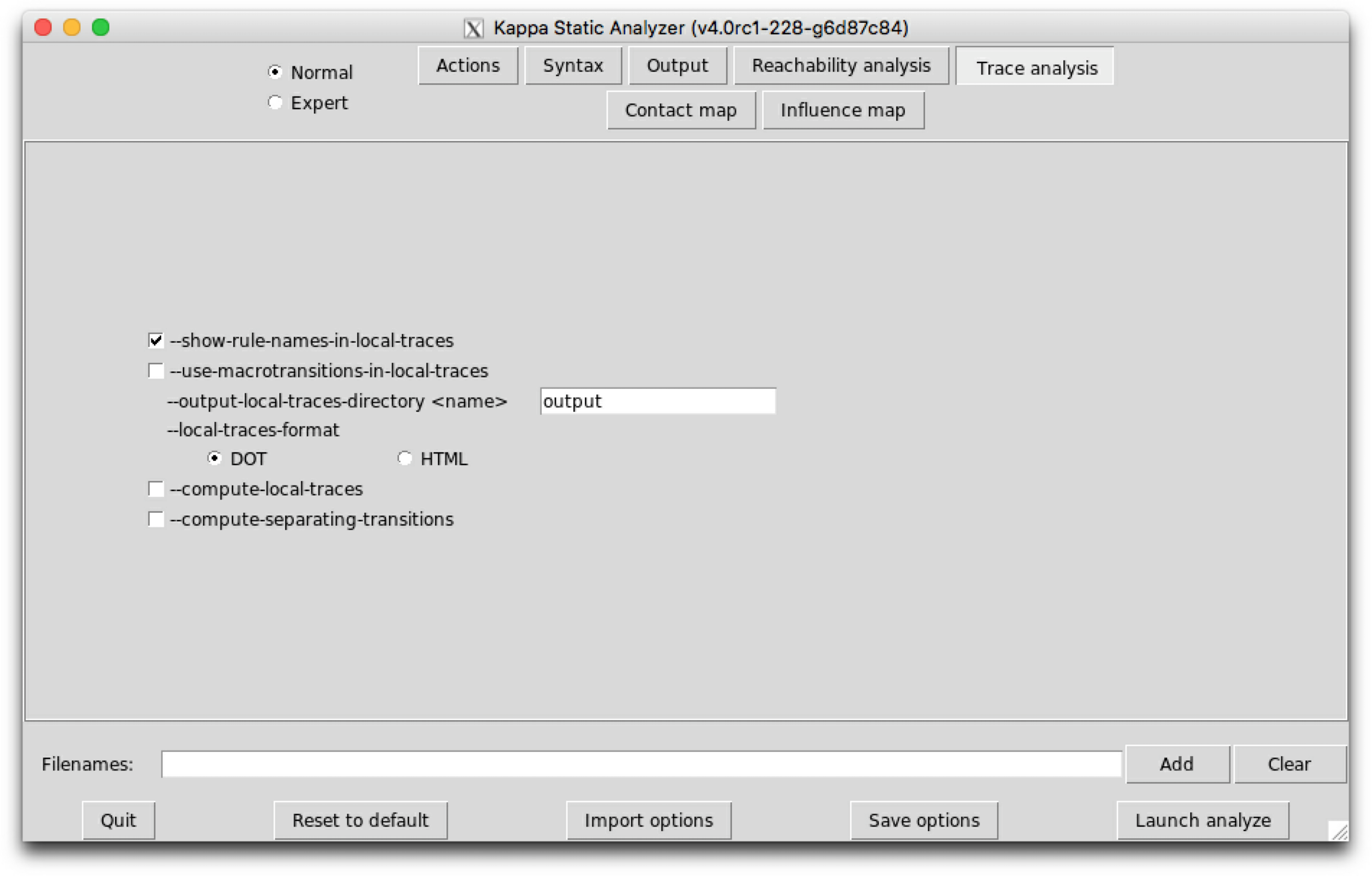Figure 6.13: KaSa  graphical interface - sub-tab Trace analysis.

Trace analysis is a reﬁnement of reachability analysis that additionaly explains how one agent can go from a given view to another one, following a path that we call a local trace. Thus the set of the local traces for a given agent can be described as a transition system among the views for a given agent: in this transition system, the nodes are local views; introduction arrows correspond to either initial states, or creation rules; transitions denote a potential conformation change of an agent, from one local views to another one, due to the application of a given rule.

We consider the following example:

1%agent: P(a1{u,p},a2{u,p},b1{u,p},b2{u,p},g)
2%agent: K(x)
3
4%init: 1 P()
5%init: 1 K()
6
7a1+ P(a1{u}) -> P(a1{p}) @1
8b1+ P(a1{p},b1{u}) -> P(a1{p},b1{p}) @1
9a1- P(a1{p},b1{u}) -> P(a1{u},b1{u}) @1
10b1- P(b1{p},g[.]) -> P(b1{u},g) @1
11a2+ P(a2{u}) -> P(a2{p}) @1
12a2- P(a2{p},g) -> P(a2{u},g) @1
13b2+ P(a2{p},b2{u}) -> P(a2{p},b2{p}) @1
14b2- P(b2{p},g) -> P(b2{u},g) @1
15P.K P(a1{p},a2{p},b1{p},b2{p},g[.]),K(x[.]) -> P(a1{p},a2{p},b1{p},b2{p},g),K(x) @1
16P/K P(a1{p},a2{p},b1{p},b2{p},g),K(x) -> P(a1{p},a2{p},b1{p},b2{p},g),K(x[.]) @1

Typing the following command line:

KaSa protein2x2.ka --reset-all --compute-local-traces

will perform the trace analysis on the model protein2x2.ka, and produce two dot format ﬁles Agent_trace_K_x^.dot and Agent_trace.P.a1_.a2_.b1_.b2_.g^.dot. The output repository can be changed thanks to the command line options --output-directory and --output-local-trace-directory. Moreover, ﬁle names are made of the preﬁx Agent_trace, followed by the kind of protein and the list of the sites of interest (the symbol ‘^’ denotes a binding state, and the symbol ‘_’ an internal state).

The transition system that describes the local traces for the agents of kind $P$ is descrided in Figure 6.14. We notice that the nodes of this transition system are labelled with the states of the sites of $P$. The internal state of a site $x$ is denoted as $x\left\{u\right\}$ (meaning that the site $x$ has state $u$, whereas the binding state of a site $x$ is denoted as $x\left[.\right]$, when the site is free, and as $x\left[x.K\right]$ when the site $x$ is bound to the site $x$ of a given agent of kind $K$.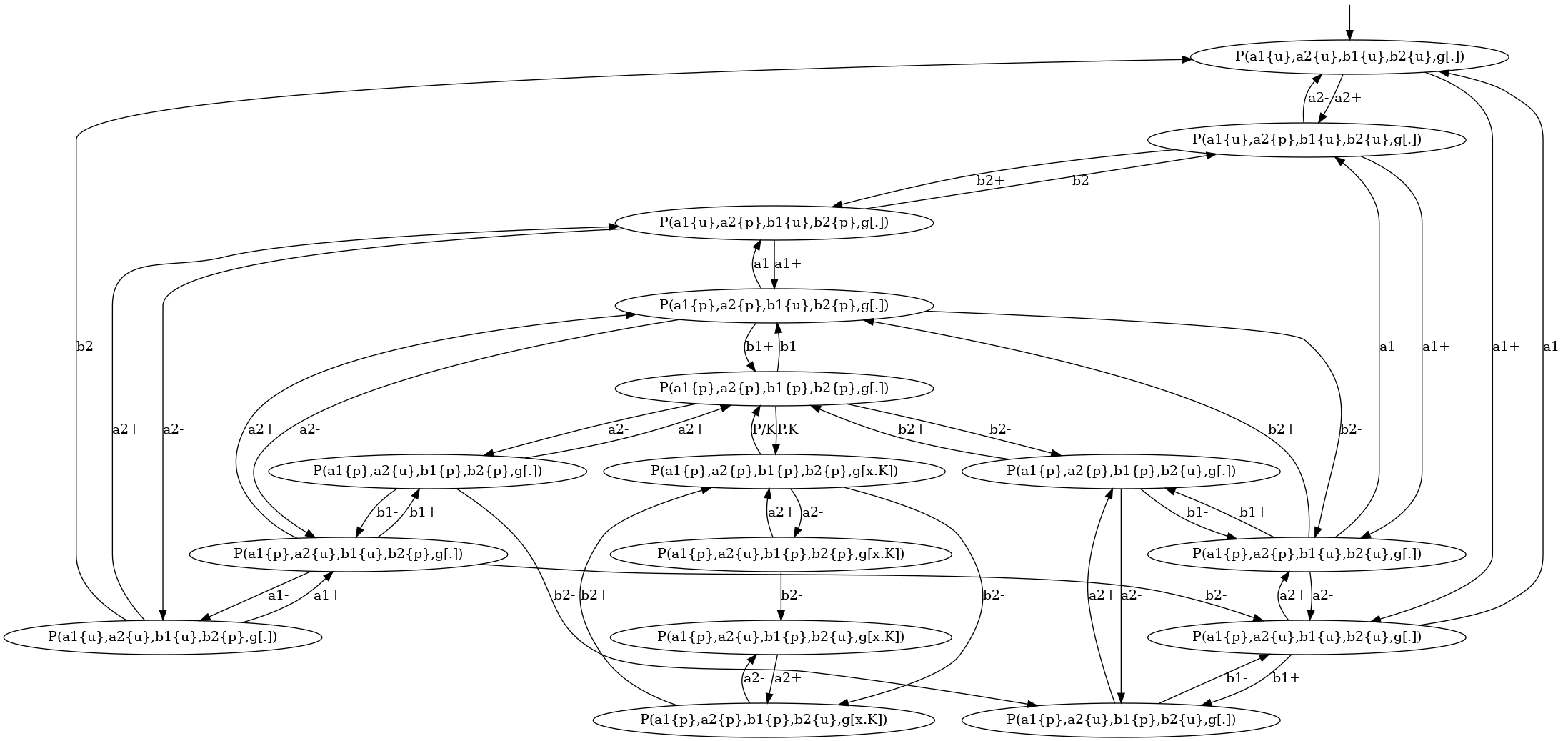Figure 6.14: Local traces for the protein2x2.ka model deﬁned in Section 6.4.

We notice that the transition system that is given in Fig. 6.14 contains too many nodes. We can coarse-grain this transition system thanks to the following option:

--use-macrotransitions-in-local-traces.

Typing the following command line:

KaSa protein2x2.ka --reset-all --compute-local-traces
--use-macrotransitions-in-local-traces

will perform the trace analysis on the model protein2x2.ka, and produce two dot format ﬁles Agent_trace_K_x^.dot and Agent_trace.P.a1_.a2_.b1_.b2_.g^.dot. The name of the output repository can be changed thanks to the command line options --output-directory and --output-local-trace-directory. This time, the ﬁles describe a coarse-graining of the corresponding transition systems.

For instance, the coarse-grained transition system for the local traces of the proteins of kind $P$ is given in Figure 6.15. This coarse-grained transition system is a compact implicit encoding of the transition system in Figure 6.14. It is obtained by exploiting the fact that locally, the behavior of the pair of states ${a}_{1}$ and ${b}_{1}$ is independent from the behavior of the pair of states ${a}_{2}$ and ${b}_{2}$, until these four sites are phosphorylated, so that the site $g$ can get bound.

More formally, in that transition system, some states are microstates (in a microstate, the state of each site is documented); some others are macrostates: (in a macrostate, the states of only a subset of site is documented). Thus a macrostate ${v}^{♯}$ can be seen intensionally as a part of a local view, but also extensionnaly as the set $\gamma \left({v}^{♯}\right)$ of the local views they are a subpart of. A microstate $v$ can be described by any sequence $\left({v}_{i}^{♯}\right)$ of macrostates providing that the intersection $\bigcap \gamma \left({v}_{i}^{♯}\right)$ of the extensional denotation $\gamma \left({v}_{i}^{♯}\right)$ of these macrostates ${v}_{i}^{♯}$ is equal to the singleton $\left\{v\right\}$; moreover a transition between two microstates $v$ and ${v}^{\prime }$ can be described by any transition between one macro state ${v}^{♯}$ and another one ${v{}^{\prime }}^{♯}$, provided that there exists a sequence of macrostate $\left({v}_{i}^{♯}\right)$ such that the sequence $\left({v}^{♯},\left({v}_{i}^{♯}\right)\right)$ denotes the microstate $v$ and the sequence $\left({v{}^{\prime }}^{♯},\left({v}_{i}^{♯}\right)\right)$ denotes the microstate ${v}^{\prime }$.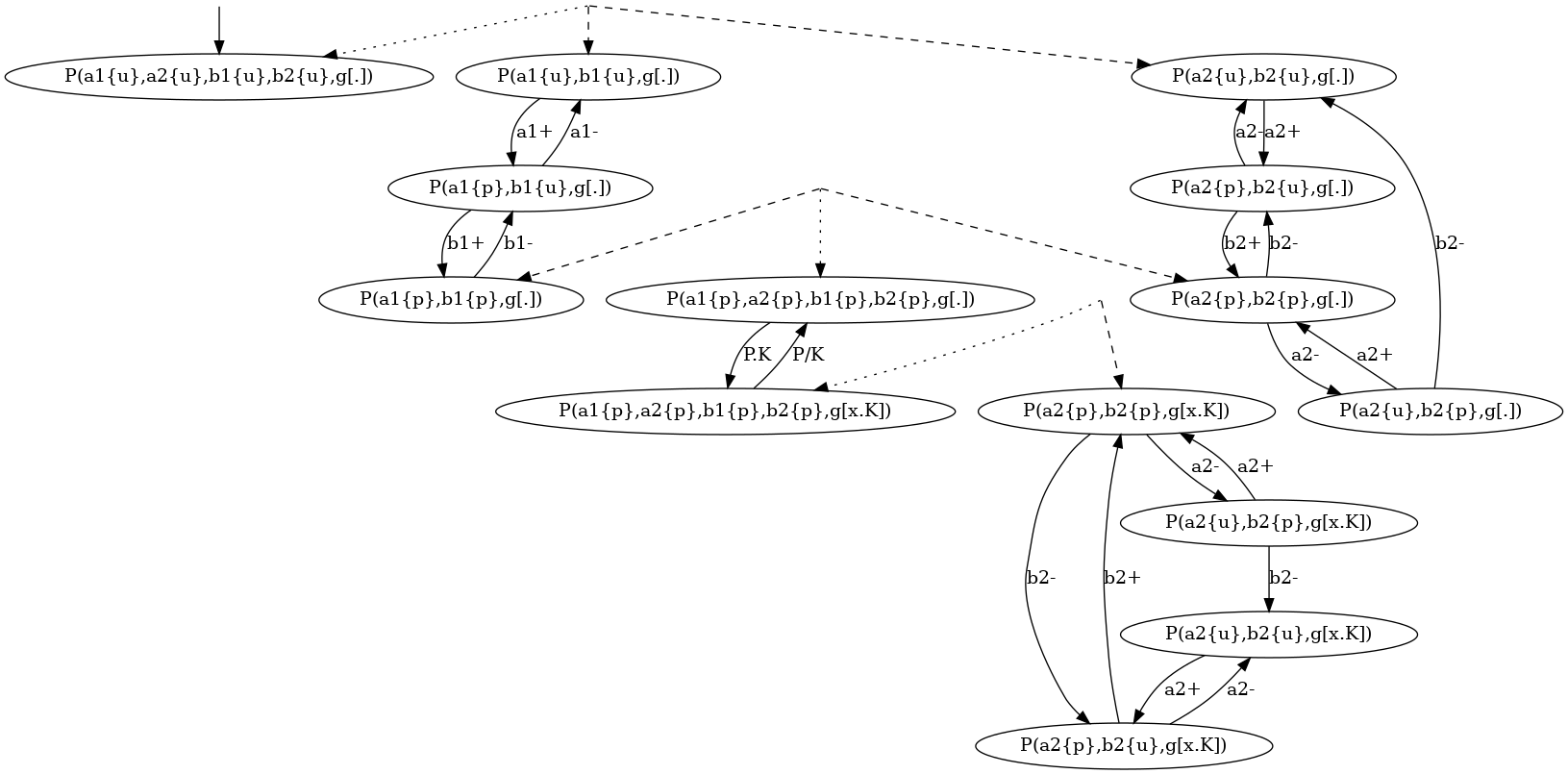Figure 6.15: Local traces for the protein2x2.ka model deﬁned in Section 6.4.

Such coarse-grained transition system can be geometrically interpreted as a simplicial complex .

As a microstate could be decomposed into several sequences of macrostates (including the trivial sequence containing only the microstate itself), the system may jump spontaneously (by using a $𝜀$ transition) from one representation to another representation. This corresponds to the intersection between several simplexes in the corresponding simpliﬁcial complex.

Although the semantics of a coarse-grainged transition system is fully deﬁned by its labelled transitions, it is useful to annotate the graph by some information about the relation between the denotation of each macrostate. By default, we use hypertlinks to relate each macrostate $v$ (including each microstate) to the set of its immediate subparts ${v}^{\prime }$. In such a hyperlink, $v$ is connected via a dotted arrow, whereas each immediate subpart is connected via a dashed arrow.

More options are available in expert mode, but they are not documented yet.

### 6.5 Contact map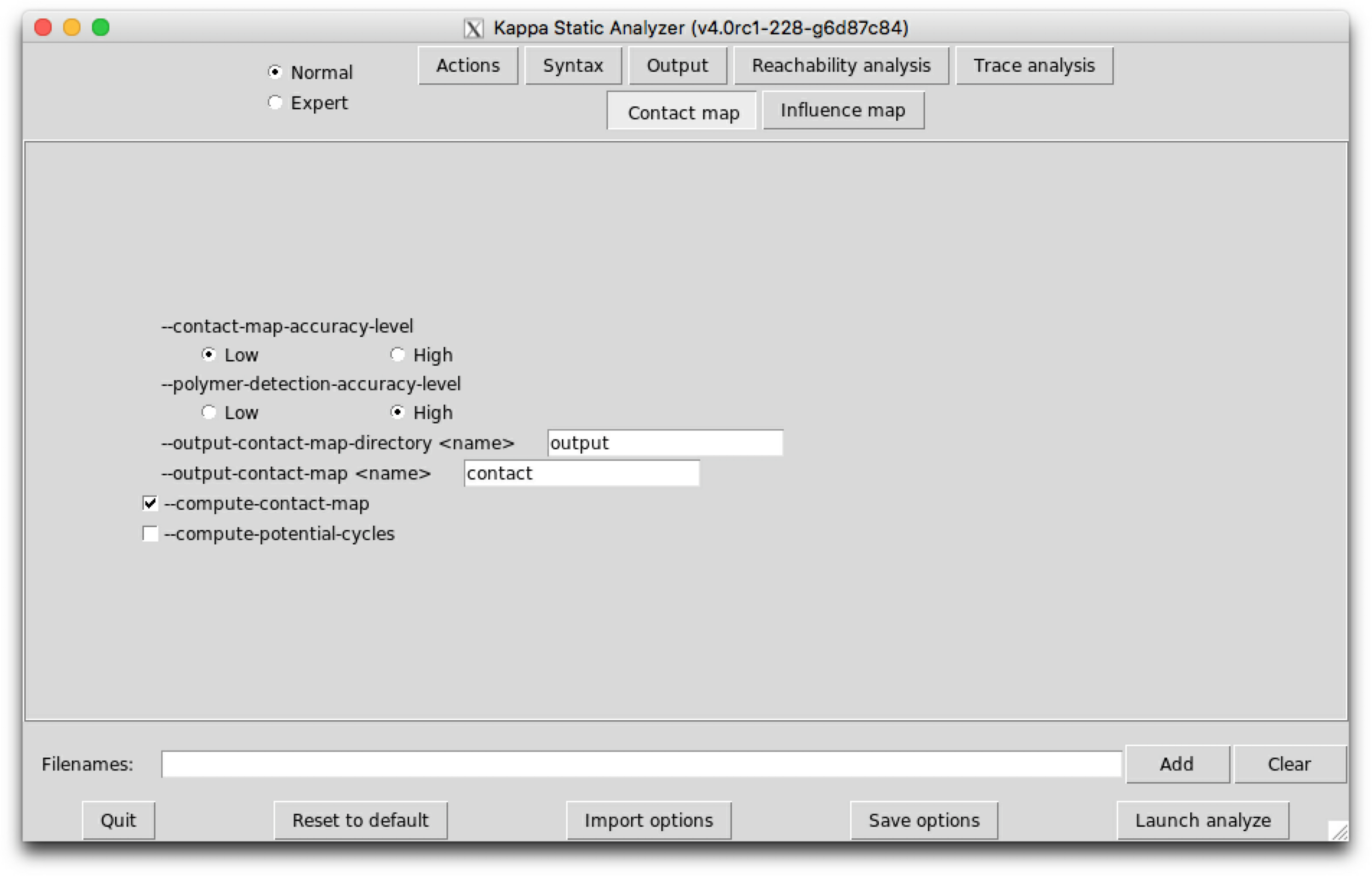Figure 6.16: KaSa  graphical interface - sub-tab Contact map.

The contact map of a model is an object that may help modelers checking the consistency of the rule set they use. The contact map is statically computed and does not depend on kinetic rates, nor the concentration of the bio-molecular species in initial state.

Typing the following command line:

KaSa abc.ka –reset-all –compute-contact-map

will produce a dot format ﬁle named contact.dot. The name of the output ﬁle and the directory can be changed thanks to the command line options --output-contact-map and --output-directory. The directory is assumed to exist. The ﬁle will be overwritten if it exists. All the options related to the computation of the contact map can be accessed on the sub-tab Contact map of the graphical interface (see Fig. 6.16).

The contact map summarises the diﬀerent types of agent, their interface and the potential bindings between sites. It is an over approximation, thus if the contact map indicates a potential bond, it does not mean that it is always possible to reach a state in which two sites of these kinds are bound, but if the contact map indicates no bond between two sites, it means that it is NOT possible to reach a state in which two sites of these kinds are bound together.

The contact map for the abc.ka model deﬁned in Chapter 1.3 is given in Figure 6.17. On this map, we notice that there are three kinds of agent, namely $A$, $B$, and $C$. Agents of kind $A$ have two sites $x$ and $c$, that bear no internal state (they appear in yellow only), agents of kind $B$ have one site $x$, that bears no internal state (they appear in yellow only), and agents of kind $C$ have two sites ${x}_{1}$ and ${x}_{2}$ with both a binding state and an internal state (they appear both in yellow and in green). We notice that when a site can bear both an internal state and a binding state, they are considered as two diﬀerent sites in the contact map. Additionally, the contact map indicates that sites $x$ of the agents of kind $A$ can be bound to the site $x$ of an agent of kind $B$ and that sites $c$ of the agents of kind $A$ can be bound to the agents of kind $C$ either on the site ${x}_{1}$, or on the site ${x}_{2}$.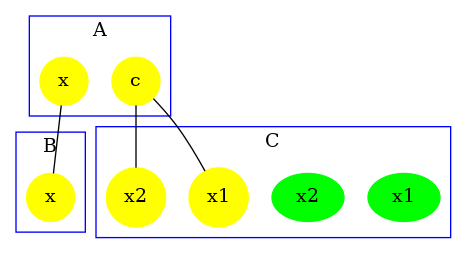Figure 6.17: Contact Map for the abc.ka model deﬁned in Chapter 1.3.

There exist two accuracy levels for the contact map. At low level of accuracy, the inference of the contact map is purely syntactic. The contact map summarizes the bonds that may occur in the right hand side of rules and in the initial bio-molecular species. At high level of accuracy, the rules that are detected dead by the reachability analysis are not taken into account. Hence, only the bonds that may occur in the initial bio-molecular species, and in the rules that have not been proven dead by the static analysis are reported. As a matter of fact, the accuracy of the computation of the contact map at high level of resolution depends on the parameterization of the reachability analysis.

Let us illustrate this on a simple example. The ﬁle contact.ka has the following content:

1%agent: A(x{c,n},z)
2
3A(x{c}[.]) <-> A(x{n}[.]) @ 1,1
4A(x{c}[.]),A(x{c}[.]) -> A(x{c}),A(x{c}) @1
5A(x{n}[.]),A(x{n}[.]) -> A(x{n}),A(x{n}) @1
6A(x{c}),A(x{c}) <-> A(x{n}),A(x{n}) @1,1
7A(z[.]),A(x{c},z[.]),A(x{n}) -> A(z),A(x{n},z),A(x{n}) @1,1
8
9%init: 10 A()

Firstly we compute the low resolution contact map by using the following instruction:

KaSa contact.ka --reset-all --compute-contact-map \
--contact-map-accuracy-level Low

We obtain the contact map that is drawed in Fig. 6.18. We notice that the analysis reports a potential bond between the sites $x$ of any two agents of type $A$ and a potential bond between the sites $z$ of any two agents of type $A$. This is because such bonds occur in the right hand side of some rules of the model.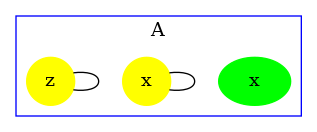Figure 6.18: Low resolution contact map for the model contact.ka

Yet, bonds between two sites $z$ occur only in the last rule which turns out to be dead. Let us use the high resolution contact map to check this property. We use the following instruction:

KaSa contact.ka --reset-all --compute-contact-map \
--contact-map-accuracy-level High

We obtain the contact map that is drawed in Fig. 6.19. We notice that the potential bond between sites $z$ of agents $A$ has disappeared: the result of the analysis has been reﬁned thanks to the constraints infered by the reachability analysis.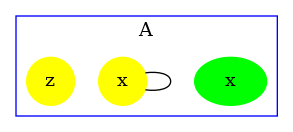Figure 6.19: High resolution contact map for the model contact.ka

The proof that, the last rule of the model in the ﬁle contact.ka is dead relies on the abstract domain, that captures the potential relationships between states of sites in the pairs of agents, that are connected by a bond. Let us compute the high resolution contact map without this abstract domain:

KaSa contact.ka --reset-all --compute-contact-map \
--contact-map-accuracy-level High --no-sites-across-bonds-domain

We obtain the contact map that is given in Fig. 6.20. The analysis has failed in proving that the last rule of the model is dead. As a matter of fact, the potential bond between sites $z$ of agents $A$ has not disappeared.Figure 6.20: High resolution contact map for the model contact.ka when the abstract domain for the relations among the states of sites in pairs of bound agents is disabled.

The contact map may be reﬁned with information about polymers. When the commmand line option –compute-potential-cycles is used, KaSa computes a superset of the bonds the number of occurences of which may not be uniformly bounded in paths within bio-molecular species. These bonds are then displayed in red in the contact map.

The computation of this subset is based on Tarjan’s algorithm for the decomposition of a graph in strongly connected components . For this, we consider the graph of the potential succesion of bonds in bio-molecular species. The nodes of this graph are the oriented version of the potential bonds in the contact map (each potential bond is considered twice). Then there is an edge between two oriented bonds if the target of the ﬁrst bond and the source of the second bond have the same agent type but diﬀerent site names. Hence each edge is associated with a graph that is obtained by merging the graph associated to the source and the target of this edge, that is to say that each edge is associated with a graph made of three agents and two bonds (e.g. see Fig. 6.21). We can notice that every path that may be repeated in bio-molecular species necessarily forms a cycle in this graph. The computation of all the elementary cycles in graph may be exponentially costly. Instead, we compute the strongly connected components which can be done in linear time (with respect to the sum among the number of nodes and the number of edges in the graph). The computation of the strongly connected components is enough, since an edge belongs to a cycle if and only if it belongs to a non trivial strongly connected component.

The ﬁle scc_abc.ka has the following content:

1
2%agent: A(x,y)
3%agent: B(x,y)
4%agent: C(x,y)
5
6A(x[.]),B(y[.]) -> A(x),B(y) @ 1
7B(x[.]),C(y[.]) -> B(x),C(y) @ 1
8C(x[.]),A(y[.]) -> C(x),A(y) @ 1
9
10%init: 10 A()
11%init: 10 B()
12%init: 10 C()

In this example, arbitrary long chains and arbitrary long rings of agents may be formed (the site $x$ of each $A$ is either free or bound to the site $y$ of a $B$; the site $x$ of each $B$ is either free or bound to the site $y$ of a $C$; and the site $x$ of each $C$ is either free or bound to the site $y$ of a $A$).

The graph that is associated to this model is given in Fig. 6.21.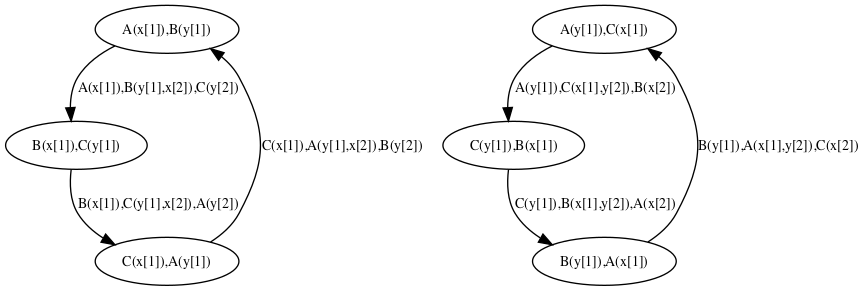Figure 6.21: The graph about potential succession of bonds for the model scc_abc.ka. Each bond in the model is encoded as a node. Each edge in the graph denotes a site-graph made of three agents.

Typing the following command line:

KaSa scc_abc.ka --reset-all --compute-potential-cycles

will generate the contact map that is given Fig. 6.22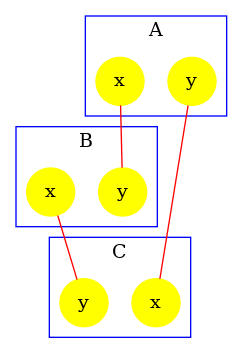Figure 6.22: The contact map for the model scc_abc.ka with information about potential polymers. Every bond is drawed in red, since the analysis cannot bound their number of occurrences in paths in reachable bio-molecular compounds.

The accuracy of the detection of polymers may be tuned in two ways. Firstly, it is parameterized of the accuracy of the contact map. Secondly, it may be reﬁned by the reachability analysis in order to discard the couple of bonds that cannot be present successively in reachable bio-molecular compounds.

The ﬁle scc_relations.ka contains the following model:

1%agent: A(x,y)
2%agent: B(x,y)
3
4A(x[.],y[.]),B(y[.]) -> A(x[.],y),B(y) @1
5A(x[.],y[.]),B(x[.]) -> A(x,y[.]),B(x) @1
6
7%init: 10 A()
8%init: 10 B()

In this model, the sites $x$ and $y$ of a given instance of the agent $A$ cannot be bound simultaneously. This prevents the formation of polymers.

By default, the detection of polymers is using the result of the reachability analysis. Thus, the following instruction:

KaSa --reset-all --compute-potential-cycles scc_relations.ka

produces the contact map that is drawed in Fig. 6.23. By default, the detection of polymers has used the result of the reachability analysis: since the bonds on the site $x$ and $y$ of each instance of the agent $A$ are mutually exclusive, no polymer may be formed.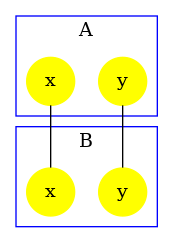Figure 6.23: The contact map for the model scc_relations.ka with information about potential polymers. Since the bonds on the sites of $A$ are mutually exclusive, no polymer may be formed. This is infered by the analysis (no bond is colored in red).

The reﬁnement by the result of the reachability analysis can be disable by using the following instruction:

KaSa --reset-all --compute-potential-cycles \
--polymer-detection-accuracy-level Low scc_relations.ka

In this case, KaSa produces the contact map that is given in Fig. 6.24. The analysis does know that the bonds of the sites of each agent of type $A$ are mutually exclusive. The bonds are drawed in red to warn about the potential formation of polymers of the model (this is a false alarm).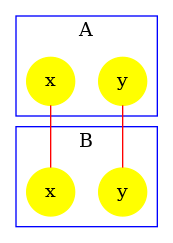Figure 6.24: The contact map for the model scc_relations.ka with information about potential polymers. Since the bonds on the sites of $A$ are mutually exclusive, no polymer may be formed. This is infered by the analysis (no bond is colored in red).

The detection of polymer at high resolution also depends on the accuracy of the reachability analysis. The ﬁle scc_dimer.ka contains the following model:

1%agent: R(x,y,z)
2
3R(x[.]),R(x[.]) -> R(x),R(x) @1
4R(x,y[.],z[.]),R(x,y[.],z[.]) -> R(x,y,z[.]),R(x,y[.],z) @1
5
6%init: 10 R()

In this model, two agents of type $R$ may connect their sites $x$ together. Then, two agents connected via their sites $x$ may establish an asymetric bond between the site $y$ of the ﬁrst one and the site $z$ of the second one. As a consequence, whenever an agent of type $R$ has several sites bound, these sites are necessarily bound to the same instance of the agent $R$. Thus, there can be no polymer.

At low resolution, the detection of polymer fails in exploiting this property, and warns about potential polymers. Indeed, the following instruction:

KaSa --reset-all --compute-potential-cycles scc_dimer.ka \
--polymer-detection-accuracy-level Low

produces the contact map that is drawed in Fig. 6.25.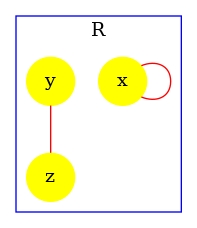Figure 6.25: The contact map for the model scc_dimer.ka with information about potential polymers at low resolution. The analysis fails in proving the lack of polymers (bonds are colored in red).

At high level of resolution, the analysis for the detection of potential polymers successfully prove the lack of polymers. The following instruction:

KaSa --reset-all --compute-potential-cycles scc_dimer.ka

produces the contact map that is given in Fig. 6.26. The analysis has successfully proven the lack of polymers (no bond is colored in red).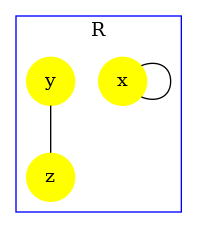Figure 6.26: The contact map for the model scc_dimer.ka with information about potential polymers at high resolution. The analysis successfully proves the lack of polymers (no bond is colored in red).

In order to illustrate that the accuracy of the analysis relies in this example on the capability for the analysis to exploit properties of double bonds. We disable the double bond domain. The following instruction:

KaSa --reset-all --compute-potential-cycles scc_dimer.ka \
--no-double-bonds-domain

produces the contact map that is given in Fig. 6.27. Without the capability to express and prove that whenever an agent of type $R$ is bound twice, it is necessarily bound twice to the same agent instance. The analysis fails in proving the absence of polymer. Thus, it warns about potential polymers, by drawing the edges in red.Figure 6.27: The contact map for the model scc_dimer.ka with information about potential polymers at high resolution but without the analysis of double bonds. The analysis cannot infer the fact that whenever two sites of an agent $R$ are bound, they are necessarily bound to the same instance of an agent. Thus, it cannot infer the lack of polymers. (bonds are colored in red)

### 6.6 Inﬂuence map

The inﬂuence map of a model is an object that may help modelers checking the consistency of the rule set they use.

Typing the following command line:

KaSa abc.ka –reset-all –compute-influence-map

will produce a dot format ﬁle named influence.dot. The name of the output ﬁle and the directory can be changed thanks to the command line options --output-influence-map and --output-directory. The directory is assumed to exist. The ﬁle will be overwritten if it exists. All the options related to the computation of the inﬂuence map can be accessed on the sub-tab Influence map of the graphical interface (see Fig. 6.28). Two formats are available for the output: inﬂuence map can be generated in DOT or HTML format. The format can be choosen thanks to the command line option --influence-map-format.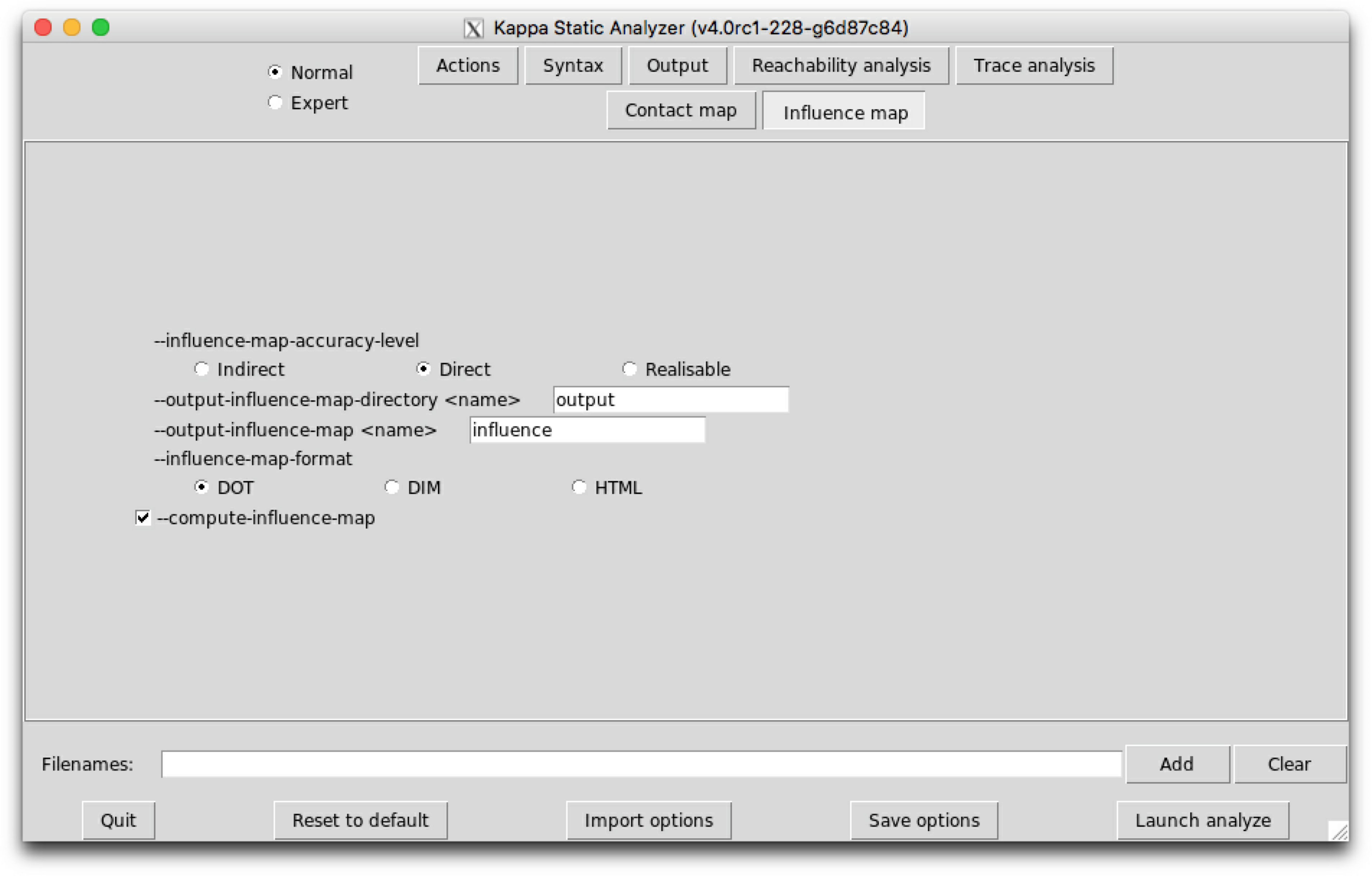Figure 6.28: KaSa  graphical interface - sub-tab Influence map.

Unlike the dynamic inﬂuence network, the inﬂuence map is statically computed and does not depend on kinetic rates nor the quantities in initial conditions. It describes how rules may potentially inﬂuence each other during a simulation. KaSa will produce a dot format ﬁle containing the inﬂuence relation over all rules and observables of the model. The produced graph visualised using a circular rendering1 is given in Figure 6.29. Observables are represented as circular nodes and rules as rectangular nodes. The labels of the nodes are either the label of the rule or of the observable (if available), otherwise they are made of a unique identiﬁer allocated by KaSa followed by the Kappa deﬁnition of the rule/observable. Edges are decorated with the list of embeddings (separated by a semi-colon) allowing the identiﬁcation of agents in both rules’ right hand sides/left hand sides. More precisely, for positive inﬂuences, the notation $\left[i\to j\right]$ denotes a pair of embeddings from the agent number $i$ of the origin's right hand side and from the agent number $j$ of the target's left hand side and the notation $\left[i\star \to j\right]$ denotes a pair of embeddings from an agent attached to the agent number $i$ of the origin's left hand side, which have been freed by side eﬀect and from the agent number $j$ of the target's left hand side; for negative inﬂuences, the notation $\left[i\to j\right]$ denotes a pair of embeddings from the agent number $i$ of the origin's left hand side and from the agent number $j$ of the target's left hand side and the notation $\left[i\star \to j\right]$ denotes a pair of embeddings from an agent attached to the agent number $i$ of the origin's left hand side, which have been freed by side eﬀect and from the agent number $j$ of the target's left hand side; Observables have no inﬂuence, but they can be inﬂuenced by rules, if the rule can increase or decrease their value.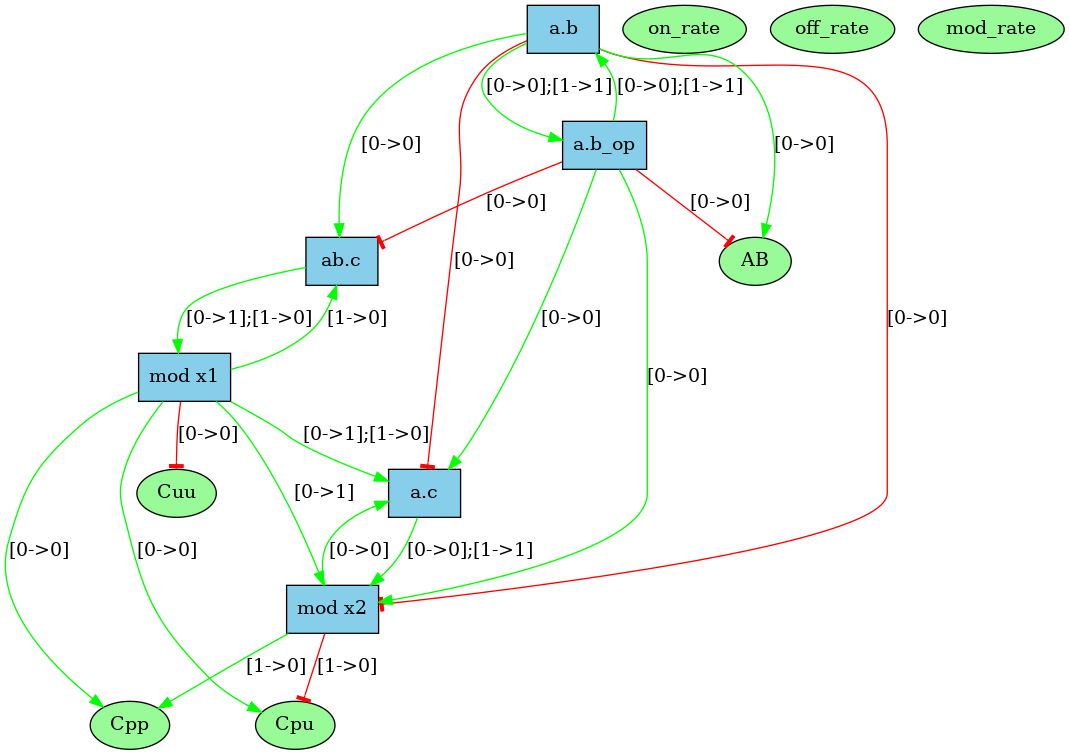Figure 6.29: The inﬂuence map of the abc.ka model deﬁned in Chapter 1.3. Edge labels denote embeddings with the convention that the notation $\left[i\to j\right]$, in a positive inﬂuence, denotes a pair of embeddings from the agent number $i$ of the origin's right hand side and from the agent number $j$ of the target's left hand side; the notation $\left[i\to j\right]$, in negative inﬂuence, denotes a pair of embeddings from the agent number $i$ of the origin's left hand side and from the agent number $j$ of the target's left hand side; the notation $\left[i\star \to j\right]$, whatever the inﬂuence is positive of negative, denotes a pair of embeddings from an agent attached to the agent number $i$ of the origin's left hand side, which have been freed by side eﬀect and from the agent number $j$ of the target's left hand side.

More formally, consider the rules $r:L\to R$ and $s:{L}^{\prime }\to {R}^{\prime }$. One wishes to know whether it is possible that the application of rule $r$ over a graph $G$ creates a new instance of rule $s$ (which is called a positive inﬂuence and that is described by green arrows in the inﬂuence map), or destroy a previous instance of rule $s$ (which is called negative inﬂuence and that is described by red arrows in the inﬂuence map). In Fig. 6.30, we illustrate the construction of positive inﬂuences due to overlap of the left hand side of a rule and the right hand side of another rule on some sites that are modiﬁed by the former one.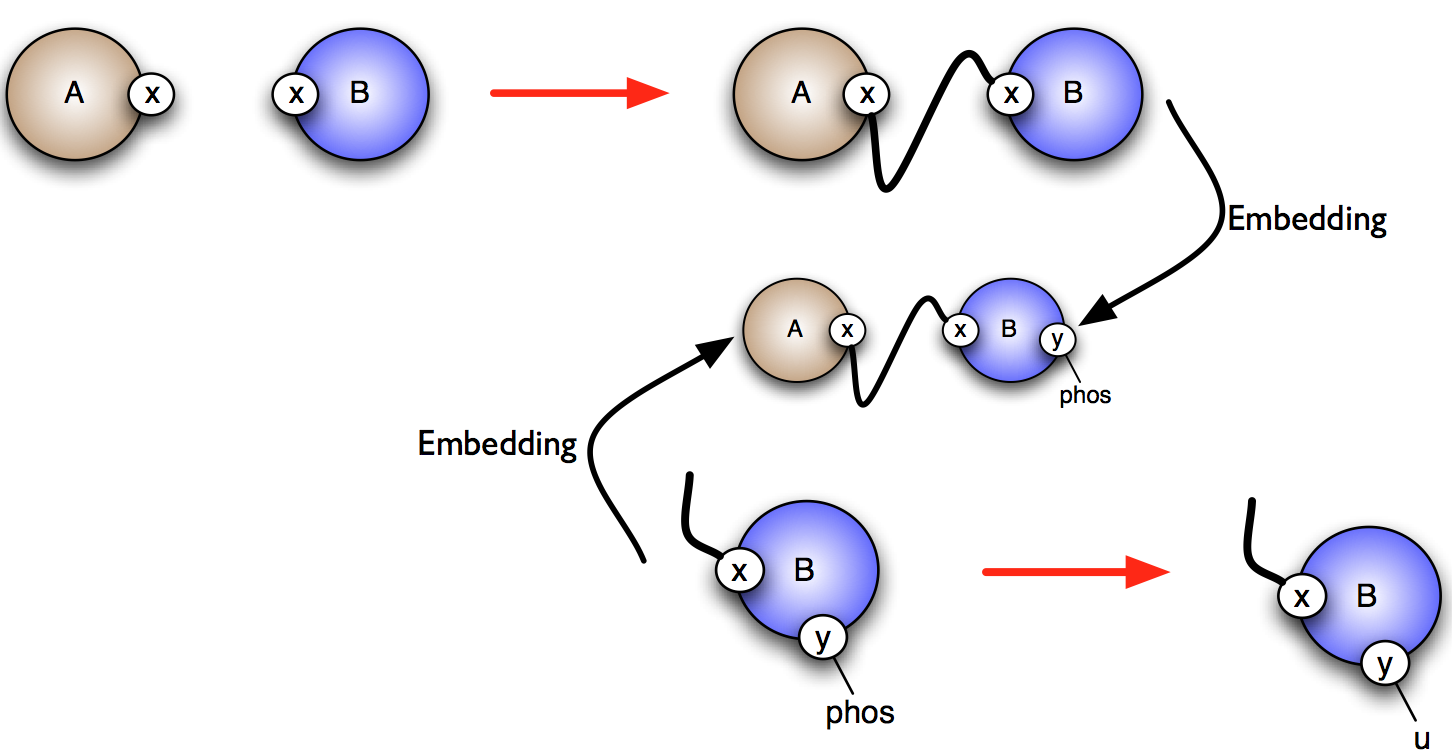Figure 6.30: Computation of the inﬂuence of the top rule on the rule below: the right hand side of the ﬁrst rule embeds in a common term with the left hand side of the second rule. It results that the ﬁrst rule has a positive inﬂuence on the second.

The current implementation has the following limitations:

• Currently, only observables that are deﬁned as patterns are taken into account.
• Not atomic observables which are deﬁned as algebraic expressions are not taken into account yet. The observables are ignored.
• The inﬂuence map does not take into account indirect inﬂuences due to interventions (which could arises when the application of a rule triggers a intervention which would create some agents or increase/decrease the value of some variables).
• Token are not taken into yet. They are currently ignored.
• Positive/negative inﬂuence of time is not taken into account either.

Lastly, KaSa computes an over-approximation of the inﬂuence map. They may show an inﬂuence despite the fact that there can be no actual one. But if it shows no inﬂuence it means that either there are NO such inﬂuence, or that we are in a case that is not covered yet as itemised previously.

Three levels of precision are available: Low, Medium, and High. The level of precision can be changed thanks to the command line option --influence-map-accuracy-level.

At low precision, an inﬂuence is detected if one rule change at least one bit of information (the internal state of a site, the binding state of a site), in favor/defavor of the application of another rule. This abstraction level ignores completely the context of application of rules, and just focuses on modiﬁcations.

At medium precision, the analysis checks that both rules have a common context.

At high precision, the analysis checks that such common context is realizable taking into account the species that have been declared as initial states and the potential introduction of species in interventions. High resolution inﬂuence is parameterized by the accuracy of the reachability analysis. It may happen that a given context is infeasible, but that this is not detected by the analysis.

Let us illustrate these three levels of accuracy by a case study.

We consider the following model.

1  %agent: A(w~u~p,x~u~p,y~u~p,z~u~p)
2
3  A(x~u) -> A(x~p) @1
4  A(x~p,y~u) -> A(x~p,y~p) @1
5  A(y~p,z~u) -> A(y~p,z~p) @1
6  A(x~u,z~p,w~u) -> A(x~u,z~p,w~p) @1
7  A(x~u,z~u) -> A(x~u,z~p) @1
8  A(x~p,w~p) -> A(x~p,w~u) @1
9
10  %init: 10 A()

The low resolution inﬂuence map is given in Fig. 6.31. There is a positive arc (in green) from a rule to another one whenever the application of the former pushes at least one bit of information towards the application of the later; whereas there is a negative arc (in red) from a rule to another one whenever the former pulls at least one bit of information away from the application of the later.

The medium resolution inﬂuence map is given in Fig. 6.32. Every arc corresponding to incompatible contexts has been removed. In our example, these are the arcs from the rule $3$ and the rule $5$ (in both direction). Despite the fact that the rule $3$ may phosphorylate the site w which is required to apply the rule $5$, no instance of the rule $5$ may be applied thanks to the application of the rule $3$ right after, because after the application of the rule $3$ the state of the site $x$ is necessarily unphosphorylated, whereas it has to be phosphorylated for the rule $5$ to be applied. The same kind of explanation holds to remove the arc from the rule $5$ to the rule $3$.

There are some structural invariants in these models. We give in Fig. 6.34 the log of the computation of the high resolution contact map. It turns out that whenever the site y of an agent is phosphorylated, then the site x of this protein is phosphorylated as well. Thus we can deduce that the positive arc from the rules $2$ and $3$, and the negative arcs from the rules $2$ and $4$ (in both direction) are false positive unless we change the set of the species in the initial state or in the interventions. Thus we obtain the high resolution inﬂuence map given in Fig. 6.33.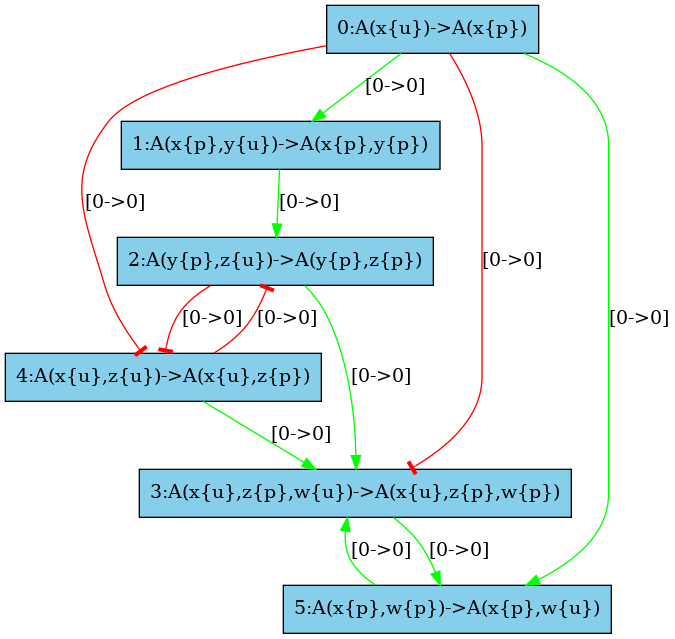Figure 6.31: Low resolution inﬂuence map.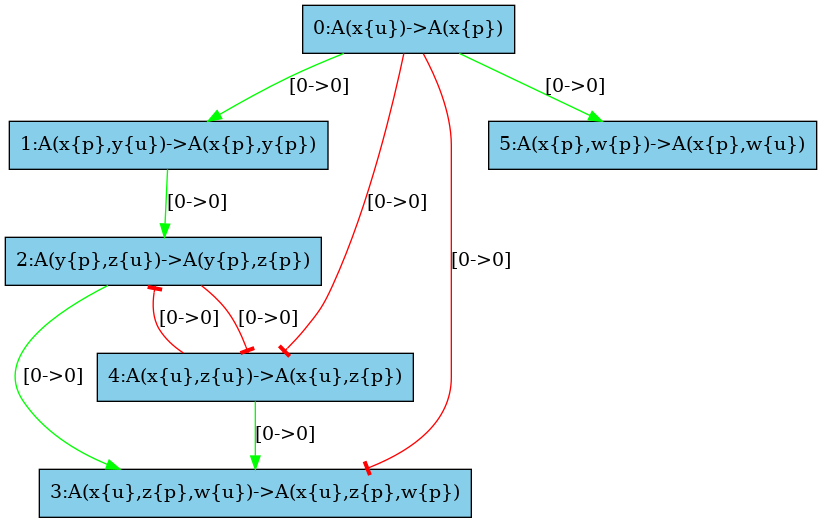Figure 6.32: Medium resolution inﬂuence map.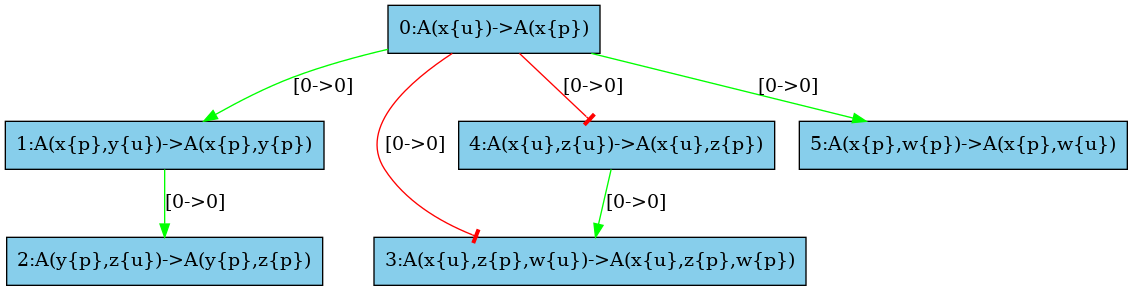Figure 6.33: High resolution inﬂuence map.

Kappa Static Analyzer (v4.1-14-g897fd18) (without Tk
interface)
Analysis launched at 2021/05/07 13:01:44 (GMT+0) on
travis-job-f7a48165-7d3f-4cbd-affb-4526961426f7
Parsing ../kappa/influence.ka...
done
Compiling...
Reachability analysis...
------------------------------------------------------------
every rule may be applied
------------------------------------------------------------
every agent may occur in the model

------------------------------------------------------------
* Non relational properties:
------------------------------------------------------------
A(w) => [ A(w{u}) v A(w{p}) ]
A(x) => [ A(x{u}) v A(x{p}) ]
A(y) => [ A(y{u}) v A(y{p}) ]
A(z) => [ A(z{u}) v A(z{p}) ]

------------------------------------------------------------
* Relational properties:
------------------------------------------------------------
A(w{p}) => A(w{p},z{p})
A(y{p}) => A(x{p},y{p})
------------------------------------------------------------
* Properties in connected agents
------------------------------------------------------------
------------------------------------------------------------
* Properties of pairs of bonds
------------------------------------------------------------
------------------------------------------------------------
* Properties of counters
------------------------------------------------------------
execution finished without any exception

Figure 6.34: Log of the reachability analysis.

## Chapter 7The KaDE ODEs generator

### 7.1 General usage

From a terminal window, KaDE can be invoked by typing the following command line:

• KaDE file_1 ... file_n [option]

where file_i are the input Kappa ﬁles containing the rules, initial conditions and observables (see Chapter 2).

All the options are summarised as follows:

General options
--help            Verbose help
-h               Short help
--version         Show version number
--gui             GUI to select
--(no-)expert     Expert mode (more options)

Data set
-(no-)initial <float>   (default: 0.000000)
Min time of simulation (arbitrary time unit)
-(no-)l <float>   (default: 1.000000)
Limit of the simulation
-(no-)p <float>   (default: 0.010000)
plot period: time interval between points in plot (default: 1.0)
-d <name>   (default: .)
Specifies directory name where output file(s) should be stored
--output <value>
Prefix for file name output

Output
--(no-)output-plot <name>   (default data.csv)
file name for data output
--ode-backend dotnet | maple | mathematica | matlab | octave | sbml
(default: octave)
Select the backend format
-mode batch | interactive
(default: interactive)
either "batch" to never ask anything to the user or "interactive" to
--(no-)constant-propagation    (default: disabled)
propagate constants
--csv-separator <name>   (default:  )
separator symbol in CSV files
--(no-)show-reactions    (default: enabled)
Annotate ODEs by the corresponding chemical reactions
--(no-)smash-reactions    (default: disabled)
Gather identical reactions in the ODEs
-d <name>   (default: .)
Specifies directory name where output file(s) should be stored
--output <value>
Prefix for file name output

Semantics
--(no-)output-plot <name>   (default data.csv)
file name for data output
--rule-rate-convention KaSim | Divide_by_nbr_of_autos_in_lhs | Biochemist
(default: Divide_by_nbr_of_autos_in_lhs)
convention for dividing constant rates (for rules)
--reaction-rate-convention KaSim | Divide_by_nbr_of_autos_in_lhs |
Biochemist
(default: Divide_by_nbr_of_autos_in_lhs)
convention for dividing constant rates (for reactions)
--count Embeddings | Occurrences
(default: Embeddings)
tune whether we count in embeddings or in occurrences
--(no-)truncate <int>   (default: disabled)
truncate the network by discarding species with size greater than the
argument
--with-symmetries None | Backward | Forward
(default: None)
Tune which kind of bisimulation is used to reduce the set of species
-syntax 3 | v3 | V3 | 4 | v4 | V4
(default: 4)
Use explicit notation for free site
-d <name>   (default: .)
Specifies directory name where output file(s) should be stored
--output <value>
Prefix for file name output

Integration settings
-(no-)initial <float>   (default: 0.000000)
Min time of simulation (arbitrary time unit)
-(no-)l <float>   (default: 1.000000)
Limit of the simulation
--(no-)output-plot <name>   (default data.csv)
file name for data output
--(no-)smash-reactions    (default: disabled)
Gather identical reactions in the ODEs
-(no-)p <float>   (default: 0.010000)
plot period: time interval between points in plot (default: 1.0)
--(no-)compute-jacobian    (default: enabled)
Enable/disable the computation of the Jacobian of the ODEs
(not
available yet)
--(no-)nonnegative    (default: disabled)
Enable/disable the correction of negative concentrations in stiff ODE
systems
--initial-step <float>   (default 0.000010)
Initial integration step
--max-step <float>   (default 0.020000)
Maximum integration step
--relative-tolerance <float>   (default 0.001000)
tolerance to relative rounding errors
--absolute-tolerance <float>   (default 0.001000)
tolerance to absolute rounding errors
-d <name>   (default: .)
Specifies directory name where output file(s) should be stored
--output <value>
Prefix for file name output

Model reduction
--with-symmetries None | Backward | Forward
(default: None)
Tune which kind of bisimulation is used to reduce the set of species
--(no-)show-symmetries    (default: disabled)
Display the equivalence relations over the sites
-d <name>   (default: .)
Specifies directory name where output file(s) should be stored
--output <value>
Prefix for file name output

Static analysis
--(no-)views-domain    (default: enabled)
Enable/disable views analysis when detecting symmetric sites
--(no-)double-bonds-domain    (default: enabled)
Enable/disable double bonds analysis when detecting symmetric sites
--(no-)site-across-bonds-domain    (default: enabled)
Enable/disable the analysis of the relation amond the states of sites
in connected agents
-d <name>   (default: .)
Specifies directory name where output file(s) should be stored
--output <value>
Prefix for file name output

Debug mode
Display time advance during numerical integration
--(no-)debug    (default: disabled)
Enable debug mode
--(no-)print-efficiency    (default: disabled)
prompt CPU time and various datas
--(no-)backtrace    (default: disabled)
Backtracing exceptions
--(no-)gluttony    (default: disabled)
Lower gc activity for a faster but memory intensive simulation
-mode batch | interactive
(default: interactive)
either "batch" to never ask anything to the user or "interactive" to
-d <name>   (default: .)
Specifies directory name where output file(s) should be stored
--output <value>
Prefix for file name output

(79 options)

Orders in option matter, since they can be used to toggle on/oﬀ some functionalities or to assign a value to some environment variables. The options are interpreted from left to right.

### 7.2 Graphical interface

#### 7.2.1 Launching the interface

The graphical interface can be launched by typing the following command line:

without any option.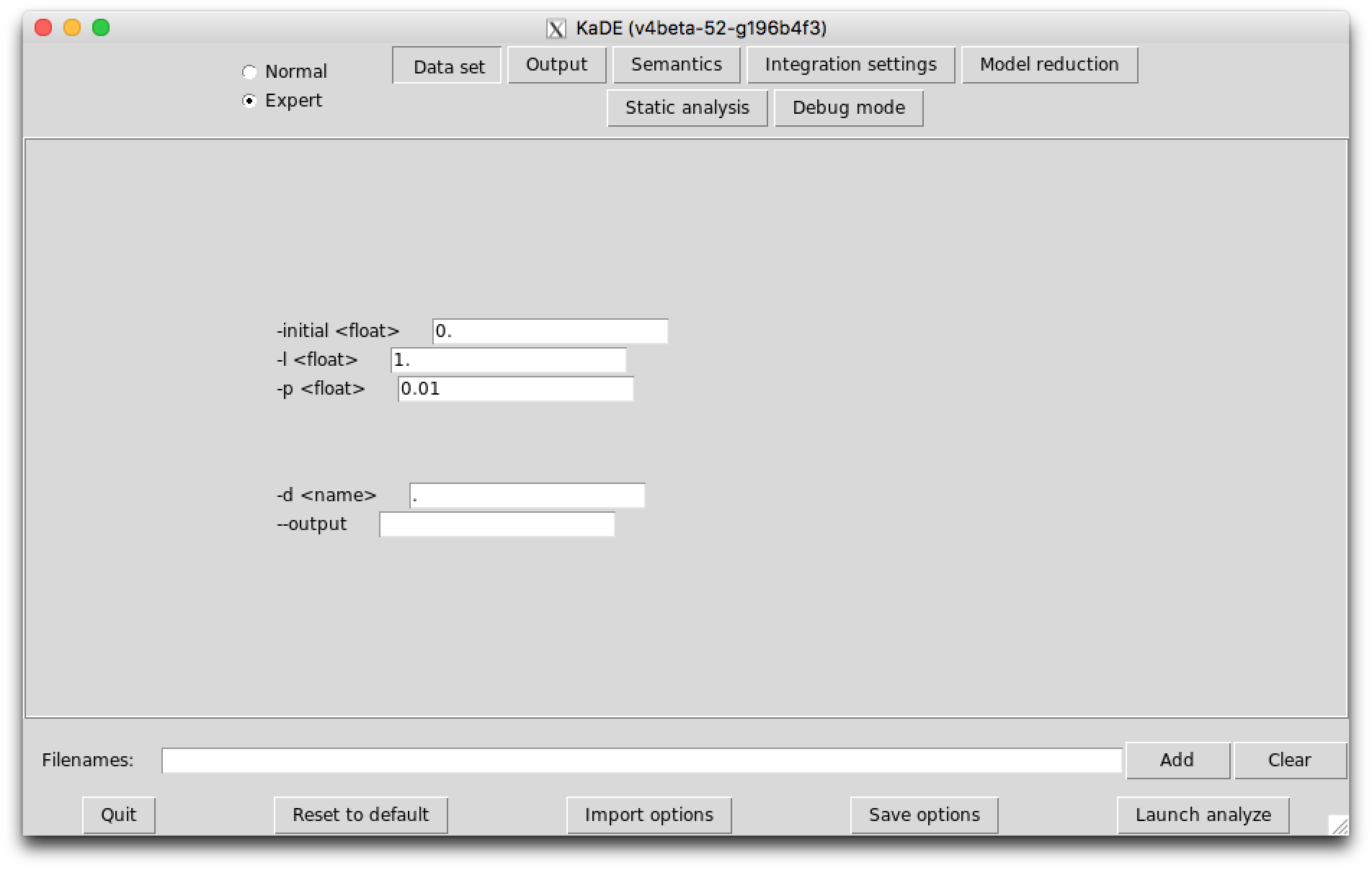Figure 7.1: KaDE  graphical interface - sub-tab Data set.

#### 7.2.2 The areas of interest

There are ﬁve diﬀerent areas of importance in the graphical interface:

1. On the top left of the window, a button allows for the selection between the Normal and the Expert mode (other modes may be available if activated at compilation). In expert mode, more options are available in the graphical interface.
2. On the top center/right, some button allows for the selection of the tab. There are currently seven sub-tabs available: Data set, Output, Semantics, Integration settings, Model reduction, Static analysis, Debug mode. The last two tabs are availanle only in expert mode (which can be selected on the top-left of the window).
3. Center: The options of the selected sub-tab are displayed and can be tuned.

Contextual help is provided when the mouse is hovered over an element. The interface will store the options that are checked or ﬁlled and the order in which they have been selected. When launched, the analysis interprets these options in the order they have been entered. Some options appear in several sub-tabs. They denote the same option and share the same value.

4. File selector: The ﬁle selector can be used to upload as many Kappa ﬁles as desired. The button ’Clear’ can be used to reset the selection of ﬁles.
5. Bottom: Some buttons are available. The button ’Quit’ can be used to leave the interface. The button ’Reset to default’ tunes all the options to their default value. The button ’Import options’ can be used to restore the value of the options as saved during a previous session of the graphical interfaces. The button ’Save options’ can be used to save the value of the options for a further session. The button ’Launch analyze’ launch KaDE with the current options.

Importantly, options are saved automatically under various occasions. Thus, it is possible to restore the value of the options before the last reset, before the last quit, or before the last analysis.

Two ﬁelds deﬁne the repository and the name of the output:

• The ﬁeld -d sets the repository where output ﬁle are written. KaDE will create this repository, if it does not exist.
• The ﬁeld --output sets the name of the output ﬁle. The following extension will be added automatically according to the choice of the backend format: ".net" in DOTNET format, ".mws" in Maple, ".nb" in Mathematica, ".m" in Matlab and Octave, and ".xml" in SBML. When the output ﬁle already exists, KaDE will ask for conﬁrmation before overwritting it, unless the tool is set in batch mode (e.g. see Sect. 7.2.6).

Both ﬁelds may be modiﬁed from every sub-tab.

#### 7.2.3 The sub-tab Data set

The sub-tab Data set (see Fig. 7.1) contains the options to tune the time range for the numerical integration and the frequency of sample plots. These ﬁelds are used only in Maple, Mathematica, Matlab, and Octave backend formats. They are ignored in SBML and DOTNET backend formats.

The following options are available:

• The ﬁeld --initial deﬁnes the starting date of the simulation.
• The ﬁeld -l deﬁnes the ﬁnal date of the simulation.
• The ﬁeld -p deﬁnes the time interval between consecutive plots.

#### 7.2.4 The sub-tab Output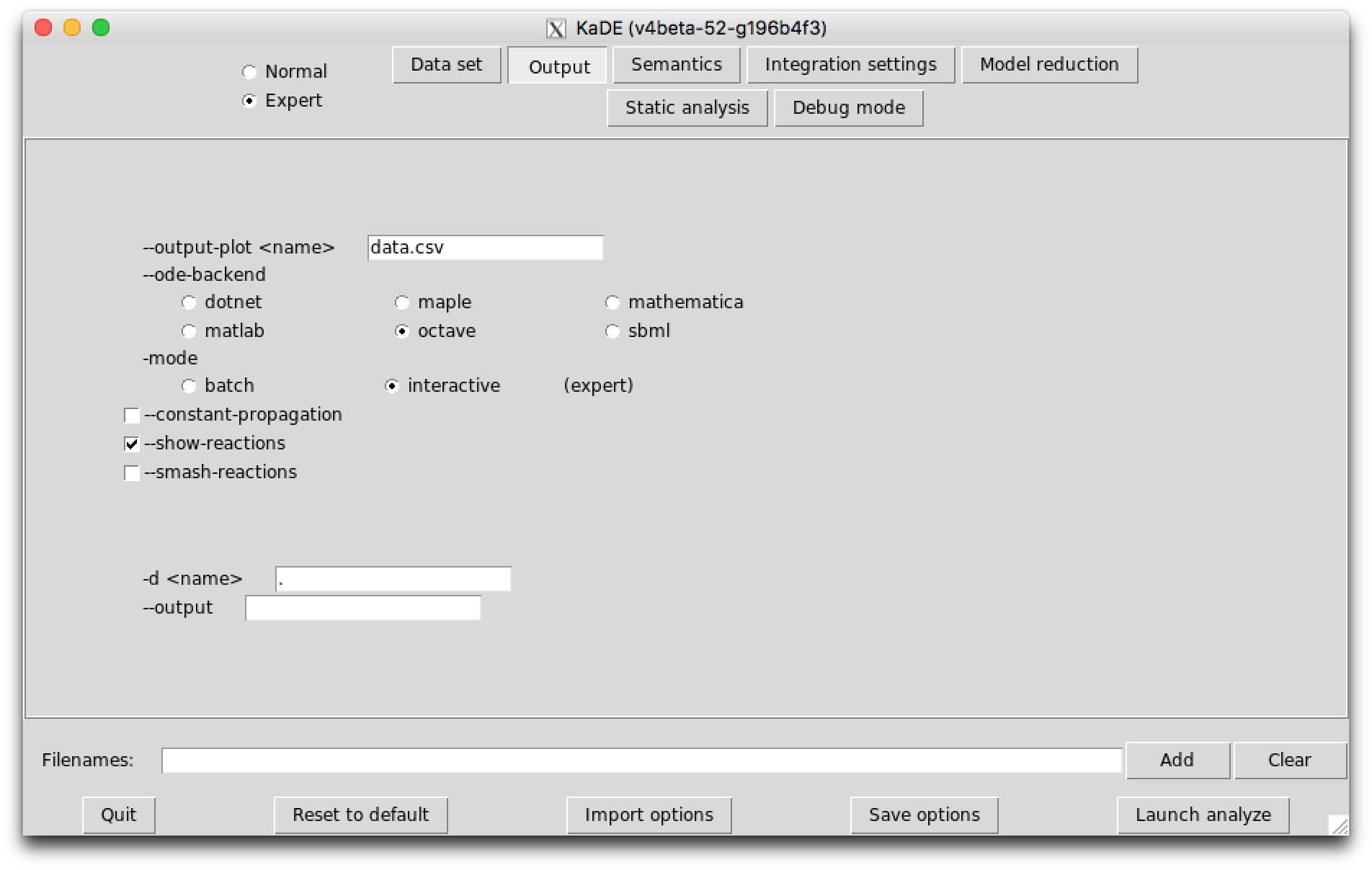Figure 7.2: KaDE  graphical interface - sub-tab Output.

The sub-tab Output (see Fig. 7.2) contains the names of the output ﬁles and their format.

The following options are available:

• The ﬁeld --ode-backend allows for the choice among the diﬀerent backend formats among DOTNET, Maple, Mathematica, Matlab, Octave and SBML.
• The ﬁeld --output-plot sets the name of the ﬁle where the plots will be dumped when it applies. Only the Maple, Mathematica, Matlab and Octave dump plots. The option is ignored in the other backend formats.
• The option --(no)-constant-propagation switches on/oﬀ constant propagation.
• The option --(no)-show-reactions annotates each expression in the output ﬁle, with the Kappa rule and the reaction that have generated this contribution.
• The option --(no)-smash-reactions identiﬁes multiple occurrences of a same reaction in the reaction network, and sums up their contribution into a single reaction.

#### 7.2.5 The sub-tab Integration settings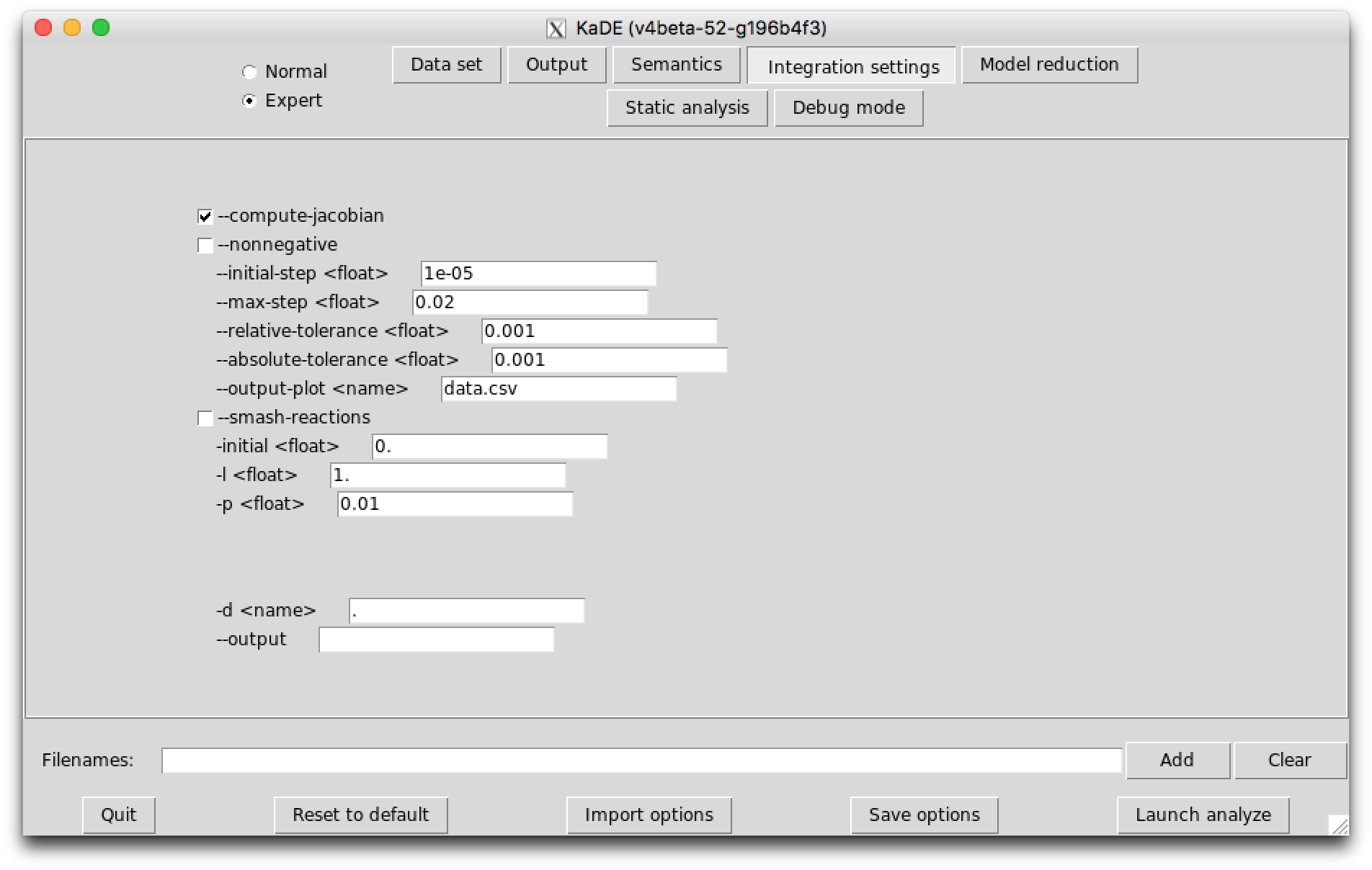Figure 7.3: KaDE  graphical interface - sub-tab Integration settings.

The sub-tab Integration settings (see Fig. 7.3) contains the parameters to guide the numerical integration engine. Except the option --smash-reaction, these options are ignored in the SBML and the DOTNET backend format.

The following options are available:

• The option --compute-jacobian switches on the computation of the Jacobian of the system of ordinary diﬀerential equations. The Jacobian is computed symbolically. It speeds up the numerical integration. The computation of the Jacobian is implemented only in two backend formats: Matlab and Octave. Other formats will ignore this option.
• The option --non-negative can be used to force concentration to have a non negative value during numerical integration. The computation of the Jacobian is implemented only in two backend formats: Matlab and Octave. Other formats will ignore this option.
• The ﬁeld --initial-step tunes the initial integration step. This ﬁeld is used only in Maple, Mathematica, Matlab, and Octave backend formats. It is ignored in SBML and DOTNET backend formats.
• The ﬁeld --max-step tunes the maximal size of integration steps. This ﬁeld is used only in Maple, Mathematica, Matlab, and Octave backend formats. It is ignored in SBML and DOTNET backend formats.
• The ﬁeld --relative-tolerance deﬁnes the relative numerical error tolerance in integration engines. This means that numerical errors cannot go beyond a bound that is proportional to the concentration of the bio-molecular species. This ﬁeld sets the value of the proportionality coeﬃcient. This ﬁeld is used only in Maple, Mathematica, Matlab, and Octave backend formats. It is ignored in SBML and DOTNET backend formats.
• The ﬁeld --absolute-tolerance deﬁnes the absolute numerical error tolerance in integration engines. This means that numerical errors cannot go beyond the value of that ﬁeld. This ﬁeld is used only in Maple, Mathematica, Matlab, and Octave backend formats. It is ignored in SBML and DOTNET backend formats.
• The ﬁeld --output-plot deﬁned the csv ﬁle where the values of the sample plots will be printed. This ﬁeld is used only in Maple, Mathematica, Matlab, and Octave backend formats. It is ignored in SBML and DOTNET backend formats.
• The option --smash-reaction gathers isomorphic reactions before generating the network or the system of ordinary diﬀerential equations (while summing their rates). This option is used in every backend format.
• The ﬁeld --initial deﬁnes the starting date of the simulation. This ﬁeld is used only in Maple, Mathematica, Matlab, and Octave backend formats. It is ignored in SBML and DOTNET backend formats.
• The ﬁeld -l deﬁnes the ﬁnal date of the simulation. This ﬁeld is used only in Maple, Mathematica, Matlab, and Octave backend formats. It is ignored in SBML and DOTNET backend formats.
• The ﬁeld -p deﬁnes the time interval between two plots. This ﬁeld is used only in Maple, Mathematica, Matlab, and Octave backend formats. It is ignored in SBML and DOTNET backend formats.

#### 7.2.6 The sub-tab Debug

In expert mode, the last sub-tab provides options to tune the amount of debugging information that is displayed.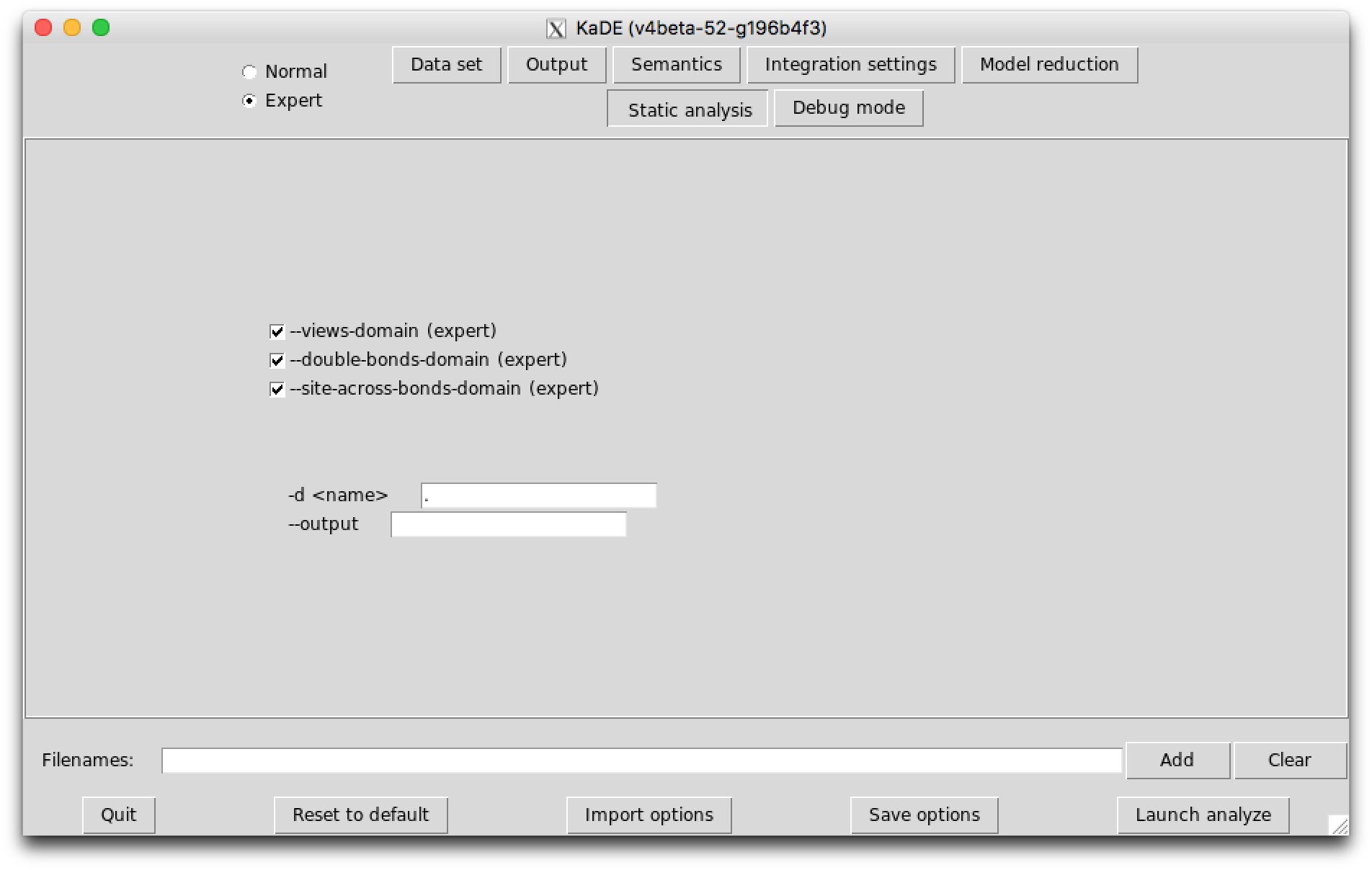Figure 7.4: KaDE  graphical interface - sub-tab 6_debug_mode.

The following options are available:

• The option --(no)-show-time-advance includes some instructions in the output ﬁle, so as to track the progress during numerical integration in the Maple, Mathematica, Matlab, and Octave backend. This option is ignored in the other backend format.
• The ﬁeld --(no)-print-efficiency provides some information about the CPU time that has been used to generate the set of reactions and about the size of the initial and reduced models.
• The option --(no)-backtrace provides more/less information about internal exceptions.
• The option --(no)-glutonny tunes the parameters of the garbage collector.
• The ﬁeld -mode allows for the choice among batch and interactive mode. In interactive mode, KaDE always asks permission to overwrite ﬁles. In batch mode, KaDE may overwrite output ﬁles, without permission.

### 7.3 Diﬀerential semantics

#### 7.3.1 From rules to reactions

In Kappa, rules may be more and less reﬁned , according to their preconditions.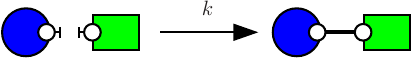Figure 7.5: Proteins K and S may bind.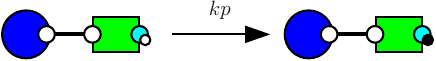Figure 7.6: The protein K may activate the protein S.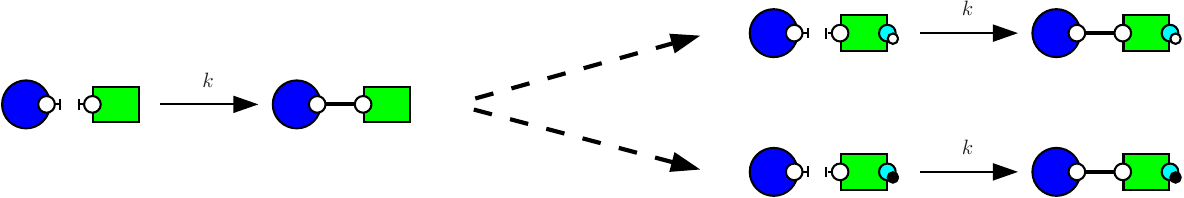Figure 7.7: From rules to reactions. The rule in Fig. 7.5 is reﬁned into two reactions according to whether the site r of the protein S is phosphorylated or not.

Consider the following two rules:

1bind  K(r),S(l) -> K(r!1),S(l!1) @k
2phos  K(r!1),S(l!1,r~u) -> K(r!1),S(l!1,r~p) @kp

The ﬁrst rule (e.g. see Fig. 7.5) stipulates that proteins of type K and proteins of type S may bind via their respective right and left sites. The second rule (e.g. see Fig. 7.6) stipulates that a protein S bound to a protein K may be activated (on its right site).

It is worth noticing that the ﬁrst rule may be applied in two diﬀerent contexts, according to whether or not the right site of the protein on the right is already phosphorylated, or not. It follows the reﬁnement that is depicted in Fig. 7.7.

In general, each rule may be reﬁned into a (potentially inﬁnite) multi-set of reactions over fully speciﬁed site-graphs. Each connected component in a reaction denotes an instance of a bio-molecular species. For every bio-molecular species $S$, a reaction ${R}_{1}+\dots +{R}_{m}\to {P}_{1}+\dots +{P}_{n}$ gives the following contribution to the derivative of the concentration of $S$,

 $\frac{\mathrm{d}\left[S\right]}{\mathrm{d}t}\stackrel{+}{=}\sum _{r}\gamma \left(r\right)\cdot \left[r,R\right]\cdot \Delta \left(R,S\right)\cdot \left[{R}_{1}\right]\cdot \dots \cdot \left[{R}_{m}\right]$

where:

1. $\left[r,R\right]$ is the number of diﬀerent ways to induce the reaction $R$ from the rule $r$;
2. $\gamma \left(r\right)$ is the corrected rate of the rule $r$ (a fraction of the rate of the rule $r$ is taken according to a choice among three conventions deﬁning how automorphisms are taken into account);
3. and $\Delta \left(R,S\right)$ is the diﬀerence between the number of occurrences of the species $S$ in the sequence ${P}_{1},\dots ,{P}_{n}$ and the one in the sequence ${R}_{1},\dots ,{R}_{m}$.

This deﬁnes the ODE semantics of Kappa models.

#### 7.3.2 Semantics convention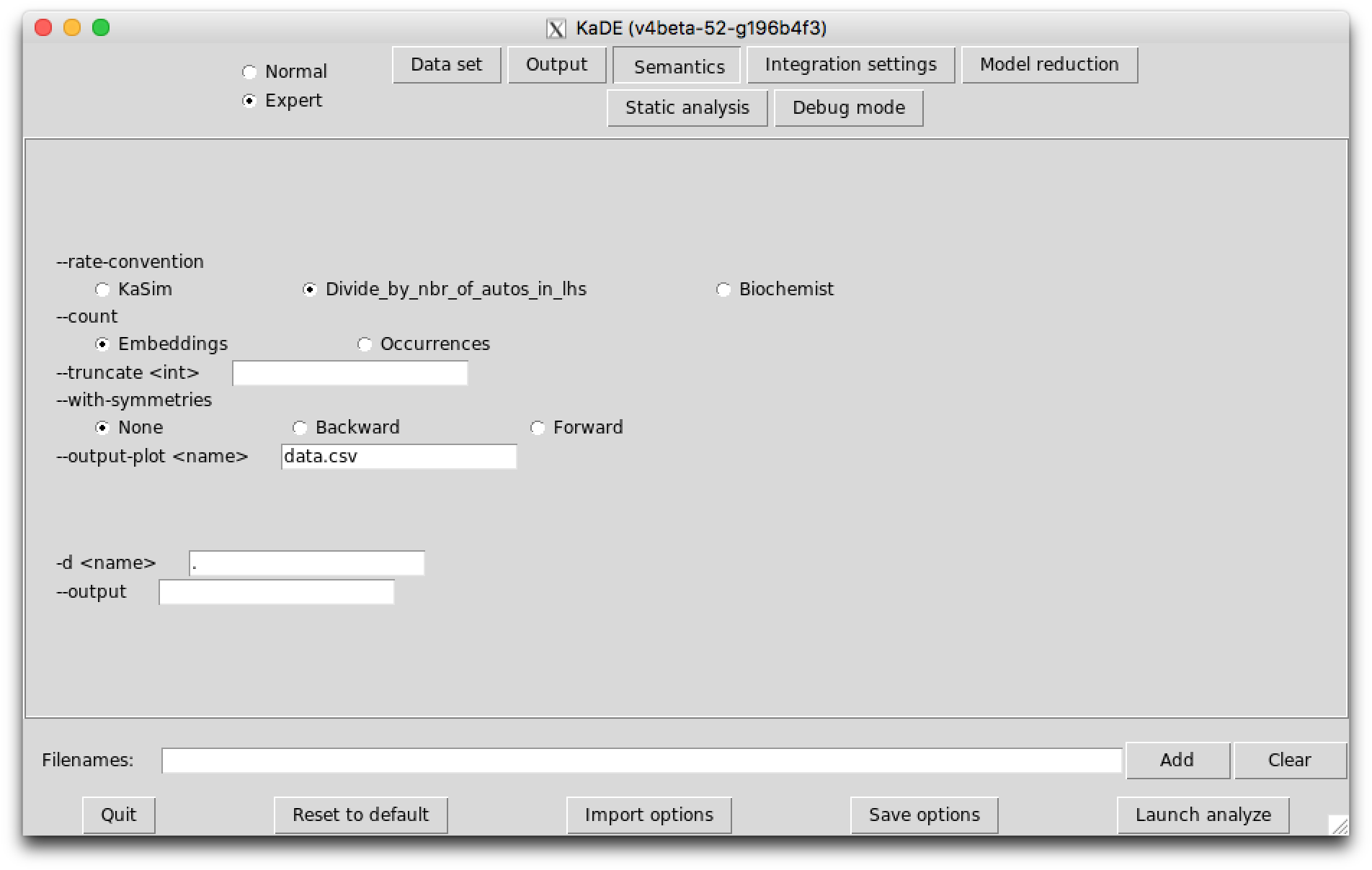Figure 7.8: KaDE  graphical interface - sub-tab 2_semantics.

##### Rate constant conventions

The options in the sub-tab Semantics allow for the choice among several possibilities for the meaning of the ODEs variables and for the meaning of the rate constants.

KaDE corrects the rate constant of each according to a convention about the number of automorphisms in this rule. KaDE oﬀers the choice among three conventions:

1. with the convention KaSim, there is no correction, as it is done in the simulator;
2. with the convention Divide_by_the_number_of_autos_in_lhs, each rate constant is divided by the number of automorphisms in the lhs of the rule, as it was done in previous version of the simulator;
3. with the convention Biochemist, each rate constant is divided by the number of the automorphisms in the lhs, that also induces an automorphism over the agents that are preserved in the rhs of the rule (more formally, an automorphism in the lhs of a rule is taken into account only if its restriction to the part that is common to the lhs and the rhs of the rule, can be extended to an automorphism of the rhs of the rule). In particular, only automorphisms that map degraded proteins, if any, to degraded proteins, are considered. Indeed this convention account for the automorphisms of the left hand side of rules which identiﬁes the agents that cannot be ditinguished from a mechanistic point of view.

Consider the following rule:

1A(u,r~u),A(u,r~u),A(u,r~u),A(u,r~u) -> A(u!1,r~u),A(u!1,r~u),A(u,r~u),A(u,r~p) @ k

which is depicted as follows: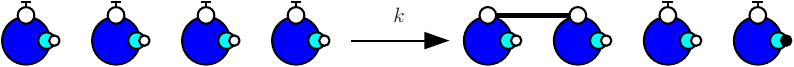with the ﬁrst convention (that is the one of the simulator), rates of rules are not corrected, hence the eﬀective rate of this rule is $k$; with the second one, rates are divided by the number of automorphisms in the left hand side of rules (here $4!$ that is to say $24$); the third convention accounts only for the permutations among the agents that are undistinguishable from a mechanistic point of view: rates are divided by the number of automorphisms in the left hand side of rules that also induce automorphisms of the right hand side (here $2$).

##### Ambiguous molecularity

The diﬀerential semantics is deﬁned as the limit of the stochastic semantics when the temperature diverges toward the inﬁnity. Thus a rate constant stands indeed for a function mapping the temperature to an eﬀective rate constant the expression of which depends on the arity of the reaction. Even if the unary rate and the binary rate of a rule may be equal for a given temperature, it is never the case at all temperatures. Another insight is that rate constants for a unary reaction and rate constants for a binary reaction are not expressed in the same physical units.

To account for this, KaDE never applies a binary rule in a unary context, unless two rate constants have been provided explicitly.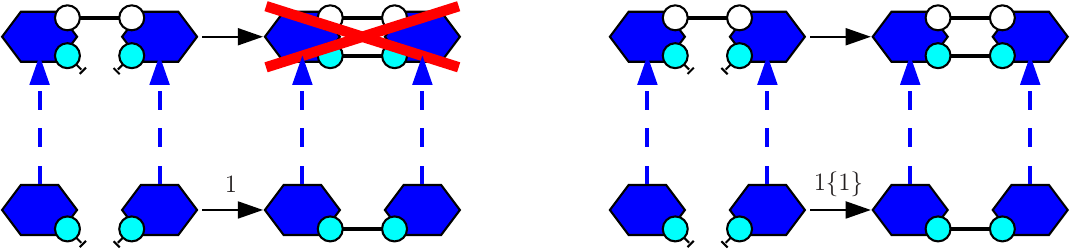Figure 7.9: The rule A(x),A(x) -> A(x!1),A(x!1) may bind two agents that are already in the same connected component, only if a second rate constant is given explictly.

Let us give an example. We consider the agent type A with two binding sites x and y. We assume that two sites x (in diﬀerent As) may be bound pair-wise and that two sites y (in diﬀerent As) may be bound pair-wise. Both following rules:

1A(x),A(x) -> A(x!1),A(x!1) @ 1
2A(x),A(x) -> A(x!1),A(x!1) @ 1{0}

will behave the same: they both apply to the following mixture

1A(x,y),A(x,y)

but not to the following one:

1A(x,y!2),A(x,y!2)

So as to allow the rule to form a bond within the two agents of the dimer, the following rule:

1A(x),A(x) -> A(x!1),A(x!1) @ 1{1}

This way, when a rule is given with one rate constant only, the connected components in its left hand side have to be reﬁned into pair-wisely disjoint bio-molecular species. When a rule is binary and when it is given with two rate constants, it may be apply in a unary context (which consists in embedding its left hand side into a single bio-molecular species) with the second rate constant, or in a binary context (which consists in embedding each connected component in its left hand side into two disjoint bio-molecular species (which may be two isomorphic copies of the same species)).

##### Embeddings VS occurrences

In a Kappa ﬁle, a pattern may denote two diﬀerent quantities. At initialisation, a pattern denotes an occurence of a bio-molecular species. In an algebraic expression (which includes functional rates, stoichiometric coeﬃcients for tokens, observables), a pattern denotes a number of embeddings from this pattern to a mixture.

Formally, we deﬁne the number (resp. the concentration) of embeddings from a pattern to a mixture (resp. to a concentration function) as the sum, for each bio-molecular species, of the number of occurrences (resp. concentration) of that bio-molecular species and the number of occurrences of the pattern in that bio-molecular species. We also deﬁne the number (resp. the concentration) of occurrences of a pattern in a mixture (resp. in a concentration function) as the quotient between the number (resp. the concentration) of embeddings from this pattern to a mixture (resp. to a concentration function) and the number of automorphisms in this pattern.

To illustrate this, we assume that the ﬁle occ.ka contains the following code:

1%agent: A(x)
2%agent: D()
3
4%init: 10 A(x),A(x)
5
6. -> D() @1
7
8%obs: dimer |A(x),A(x)|

We use the following command line to get the value of the observable:

• KaSim occ.ka -l 1 -p 0.5 -o occ.csv

The ﬁle csv contains the following data set:

# Output of KaSim ’-mode batch ’-i ’../kappa/occ.ka ’-l ’1’ ’-p ’0.5’ ’-d ’../generated_img/’ ’-o occ.csv -seed 326572406
# "uuid" : "185566741"
"[T]","dimer"
0.,20
0.5,20
1.,20

We notice that KaSim indicates $20$ for the quantities of dimers. This accounts for the fact that each dimer satisﬁes two automorphisms.

The command-line option --count [Embeddings | Occurrences] changes the meaning of the variables that occur in the diﬀerential equations. The choice has no impact on the quantities that are plot. Introduced bio-molecular species are always introduced in concentration of occurrences, and patterns in algebraic expressions always denote concentration of embeddings. It just changes the meaning of the variables that are used internally in the diﬀerential equations.

##### Polymers

In case of polymerisation, the size of the bio-molecular species is potentially unbounded and there may be an inﬁnite amount of diﬀerential equations (this is the case even if there is no agent synthesis, since the initial state is given in concentration, and not in occurrence number). In such a case, KaDE will not terminate. Yet it is possible to truncate the system of ordinary diﬀerential equations: the command-line option --truncate speciﬁes an upper bound to the number of agents in the bio-molecular species. Each reaction that would involve a larger bio-molecular species is discarded.

For instance, we can consider the model poly.ka that is deﬁned as follows:

1  %agent: A(l,r)
2  %init: 10 A()
3  A(r), A(l) -> A(r!1),A(l!1) @ 1

The command line:

will not terminate, wheareas the following one:

will.

##### Equivalent sites

In BNGL, the interface of a given agent may include several occurrence of a given site name. These sites have exactly the same capabilities of interaction. This feature provides a convenient syntactic construction to describe models even more compactly, at the cost of having to deal with more complex structures (detecting embeddings between such site-graphs may be very diﬃcult).

In Kappa, the interface of every agent is made of pair-wisely distinct site names. Yet it may happen that some sites have exactly the same capabilities of interaction. KaDE can infer this property and use it to derive a more compact system of diﬀerential equations .

Consider the following model:

1%agent: A(x,y)
2%init: 10 A()
3
4A(x,y),A(x,y) -> A(x!1,y),A(x!1,y) @1
5A(x,y),A(x,y) -> A(x,y!1),A(x,y!1) @1
6A(x,y),A(x,y) -> A(x!1,y),A(x,y!1) @2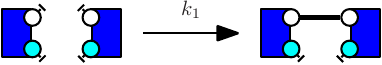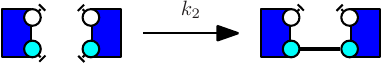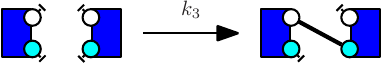Figure 7.10: Sites are equivalent, if the corrected rates of the third rule is twice the corrected rate of each other rule.

The rules are depicted in Fig. 7.10. We notice that each rule may be obtained from one another by swapping the sites in agents. So we say that sites are equivalent in this set of rules. Equivalent sites may be used to induce forward and backward bisimulations  over the stochastic and the diﬀerential semantics of Kappa.

Let us consider two site names x and y in the signature of an agent type A. A set of rules is symmetric with respect to x and y, if for every two rules that may be obtained one from the other one by permuting the sites x and y in some agents of type A, their corrected rates is inversely proportional to their number of automorphisms. In our example, the set of rules is symmetric with respect to x and y only with the convention in which constant rates are kept as they are given, without any corrective factor. A valuation from bio-molecular species to real numbers is symmetric with respect to x and y, if for every two bio-molecular species that can be obtained one from the other one by permuting the sites x and y in some agents of type A, the image of the two bio-molecular species by the valuation is inversely proportional to their number of automorphisms. Lastly an expression over bio-molecular species is symmetric with respect to x and y, if and only if it takes the same values for every two symmetric valuations.

If the set of rules and the initial state of the model are symmetric with respect to two sites, ignoring the diﬀerence among these two sites in bio-molecular species induces a backward bisimulation (that is to say, the state of the system remains symmetric at every time ). If the set of rules and every algebraic expression that occurs in rates or stoichiometric coeﬃcients are symmetric, then ignoring the diﬀerence between these two sites in bio-molecular species induces a forward bisimulation (we can deﬁne the ODEs directly over the equivalence-classes of bio-molecular species ).

The following option is available:

• The command line option --with-symmetries [ None | Backward | Forward ] sets which kind of bisimulation is used to reduce the system of ordinary diﬀerential equations.

#### 7.3.3 The sub-tab Model reduction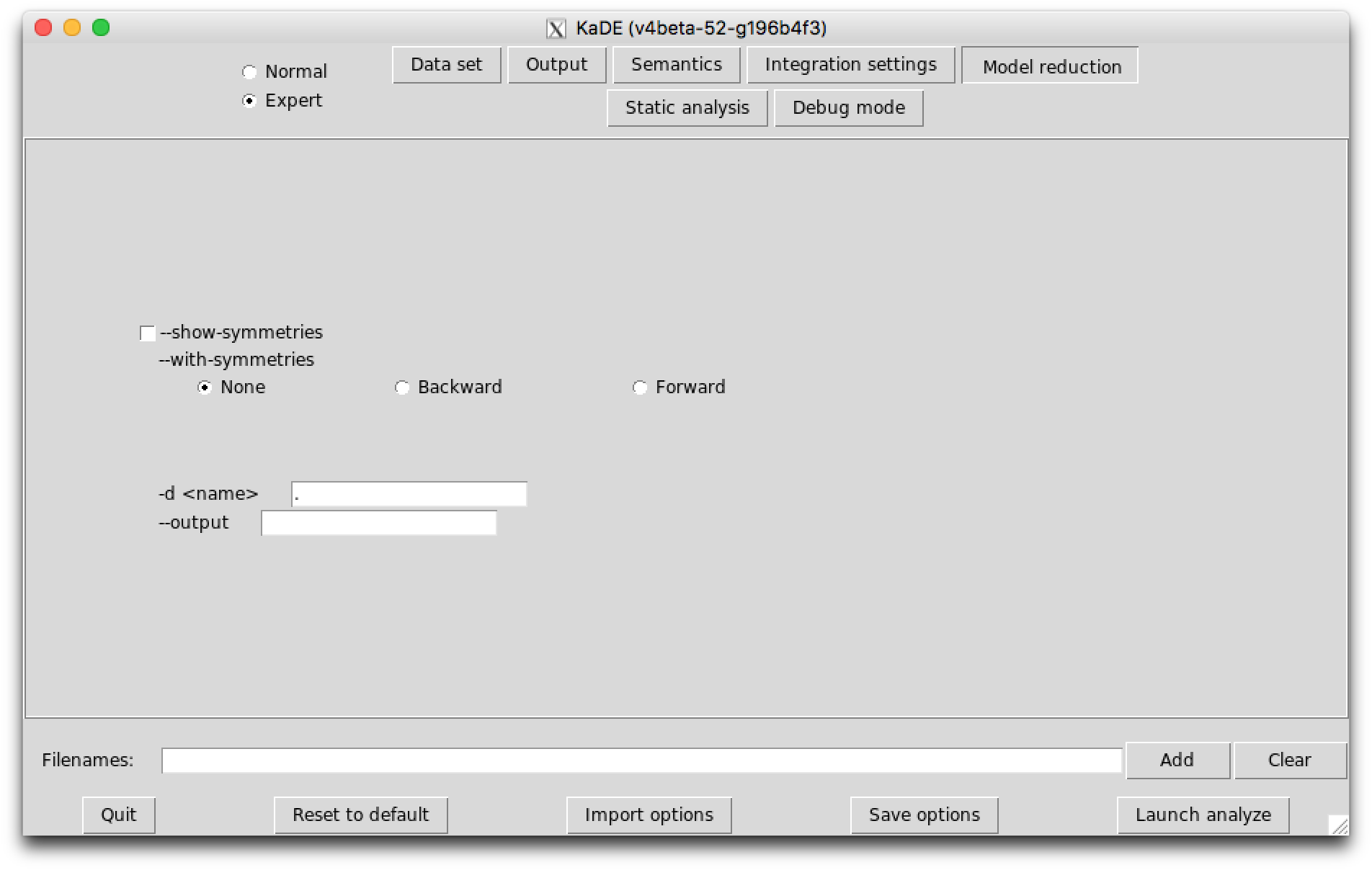Figure 7.11: KaDE  graphical interface - sub-tab Model reduction.

The following options are available:

• The option --show-symmetries itemizes the sites that are equivalent with respect to the rules of the models, with respect to the rules of the model and the initial state (backward bisimulation), and with respect to the rules of the models and the algebraic expressions (forward bisimulation).
• The command line option --with-symmetries [ None | Backward | Forward ] sets which kind of bisimulation is used to reduce the system of ordinary diﬀerential equations.
##### The sub-tab Static analysis (Expert)Figure 7.12: KaDE  graphical interface - sub-tab Static analysis.

The inference of equivalent sites is more precise when dead rules are discarded. Dead rules can be computed thanks to KaSa. The sub-tab Static analysis allows the end-user to switch on/switch oﬀ abstract domains in order to tune the trade-oﬀ between accuracy and eﬃciency of static analysis.

The following options are available:

• The option --(no-)views-domain can be used to switch on/oﬀ the views domains.
• The option --(no-)double-bonds-domain can be used to switch on/oﬀ the analysis of potential double bonds between proteins.
• The option --(no-)site-across-bonds-domain can be used to switch on/oﬀ the analysis of the relations among the states of the sites in connected proteins.

### 7.4 Tutorial

Now we explain further how to use KaDE to deal with the example of Sect. 7.3.2. The model is described in the following Kappa ﬁle:

1//sym.ka
2
3%agent: A(x y)
4
5%init: 100 A()
6
7%var: k 1
8%obs: asym |A(x),A(y)|
9
10A(x[.] y[.]),A(x[.] y[.]) -> A(x y[.]),A(x y[.]) @k
11A(x[.] y[.]),A(x[.] y[.]) -> A(x y[.]),A(x[.] y) @k
12A(x[.] y[.]),A(x[.] y[.]) -> A(x[.] y),A(x[.] y) @k
13
14//We use the third convention (consider only the automorphisms in the lhs
15//that are preserved in the rhs). There are two of them in the first and
16//in the third rule. Only one in the second one. Hence the rate of the first
17//and third are divided by 2.

The rules of this models are depicted as follows:We denote as ${\gamma }_{1}$, ${\gamma }_{2}$, and ${\gamma }_{3}$ the corrected rate constants of these rules. With the third convention, which roughly speaking consists in dividing rates per the number of automorphisms in the left hand side of rules, that are preserved in the right hand side, we have:

• ${\gamma }_{1}=\frac{{k}_{1}}{2}$;
• ${\gamma }_{2}=\frac{{k}_{2}}{2}$;
• ${\gamma }_{3}={k}_{3}$.

Indeed, in the third rule, the mechanism makes a diﬀerence among the two agents. The non trivial automorphism in the left hand side is not preserved in the right hand side.

Rules $1$ and $2$ have two automorphisms, whereas rule $3$ has only one. As a consequence, the rules are symmetric with respect to both sites if and only $2\cdot \frac{{k}_{1}}{2}=2\cdot \frac{{k}_{2}}{2}={k}_{3}$, that is to say ${k}_{1}={k}_{2}={k}_{3}$.

We use the following command line to generate the ODE semantics in Octave:

By default, equivalent sites are not analysed and the Octave backend is used.

The result is dumped in the ﬁle ’ode.m’, which is editable. Moreover, each instruction is annotated with some information referring to the Kappa ﬁle. Variables are annotated by the corresponding name in the Kappa ﬁle. Initial species are annotated by Kappa expressions describing the corresponding bio-molecular species.

Integration parameters are deﬁned as follows:

• grep -n -m 1 ’tinit=’ ode.m –after-context 8

19:tinit=0;
20-tend=1;
21-initialstep=1e-05;
22-maxstep=0.02;
23-reltol=0.001;
24-abstol=0.001;
25-period=0.01;
26-nonnegative=false;
27-

The variable nodevar gives the number of variables:

• grep -n -m 1 ’nodevar=’ ode.m

29:nodevar=5;

The function ode_init deﬁnes the list of variables and their initial concentration:

• grep -n -m 1 ’function Init’ ode.m –after-context 11

176:function Init=ode_init()
177-
178-global nodevar
179-global init
180-Init=zeros(nodevar,1);
181-
182-Init(1) = init(1); % A(x[.],y[.])
183-Init(2) = init(2); % A(x,y[.]), A(x,y[.])
184-Init(3) = init(3); % A(x,y[.]), A(x[.],y)
185-Init(4) = init(4); % A(x[.],y), A(x[.],y)
186-Init(5) = init(5); % t
187-end

We notice that four variables encode the concentration of bio-molecular species and a special one accounts for time advance.

The function dydt deﬁnes the diﬀerential equations:

• grep -n -m 1 ’function dydt’ ode.m –after-context 38

190:function dydt=ode_aux(t,y)
191-
192-global nodevar
193-global max_stoc_coef
194-global var
195-global k
196-global kd
197-global kun
198-global kdun
199-global stoc
200-
201-var(2)=y(3); % asym
202-
203-
204-dydt=zeros(nodevar,1);
205-
206-% rule    : A(x[.],y[.]), A(x[.],y[.]) -> A(x[.],y), A(x[.],y)
207-% reaction: A(x[.],y[.]) + A(x[.],y[.]) -> A(x[.],y).A(x[.],y)
208-
209-dydt(1)=dydt(1)-1/2*k(3)*y(1)*y(1);
210-dydt(1)=dydt(1)-1/2*k(3)*y(1)*y(1);
211-dydt(4)=dydt(4)+2/2*k(3)*y(1)*y(1);
212-
213-% rule    : A(x[.],y[.]), A(x[.],y[.]) -> A(x,y[.]), A(x[.],y)
214-% reaction: A(x[.],y[.]) + A(x[.],y[.]) -> A(x,y[.]).A(x[.],y)
215-
216-dydt(1)=dydt(1)-1/2*k(2)*y(1)*y(1);
217-dydt(1)=dydt(1)-1/2*k(2)*y(1)*y(1);
218-dydt(3)=dydt(3)+1/2*k(2)*y(1)*y(1);
219-
220-% rule    : A(x[.],y[.]), A(x[.],y[.]) -> A(x,y[.]), A(x,y[.])
221-% reaction: A(x[.],y[.]) + A(x[.],y[.]) -> A(x,y[.]).A(x,y[.])
222-
223-dydt(1)=dydt(1)-1/2*k(1)*y(1)*y(1);
224-dydt(1)=dydt(1)-1/2*k(1)*y(1)*y(1);
225-dydt(2)=dydt(2)+2/2*k(1)*y(1)*y(1);
226-dydt(5)=1;
227-
228-end

The function obs deﬁnes the observables:

• grep -n -m 1 ’function obs’ ode.m –after-context 12

288:function obs=ode_obs(y)
289-
290-global nobs
291-global var
292-obs=zeros(nobs,1);
293-
294-t = y(5);
295-var(2)=y(3); % asym
296-
297-obs(1)=t; % [T]
298-obs(2)=var(2); % asym
299-
300-end

The function jac provides the Jacobian (we omit it).

We now wonder whether the sites are equivalent or not. We use the following command line:

• KaDE –rate-convention Biochemist sym.ka –show-symmetries

The status of equivalent sites is described in the log:

Symmetries:
In rules:
************
Agent: A
-Equivalence classes of sites for bindings states:
{x,y}
-Equivalence classes of sites (both):
{x,y}
************
In rules and initial states:
************
Agent: A
-Equivalence classes of sites for bindings states:
{x,y}
-Equivalence classes of sites (both):
{x,y}

The set of rules and the initial state are symmetric with respect to the pair of sites. This is not the case of the observable. Thus, only backward bisimulation may be used to reduce the system. Indeed, if we ignore the diﬀerence between sites x and y, we can no longer express the concentration of asymmetric dimers. This excludes forward bisimulation. Backward bisimulations may still be used since the concentration of each species can be computed by from the overall concentration of its equivalence class, since the concentration of two equivalent species are always inversely proportional to their number of automorphisms.

The command line:

• KaDe –rate-convention Biochemist sym.ka –with-symmetries Forward –output ode_with_fwd_sym –output-plot data_fwd.csv

gives the same model, without any reduction.

The command line:

• KaDe –rate-convention Biochemist sym.ka –with-symmetries Backward –output ode_with_bwd_sym –output-plot data_bwd.csv

reduces the system by ignoring the diﬀerence between sites x and y. This is done by replacing in each reaction product, every species by an arbitrary representative of its equivalence class, and in each algebraic expression, each species concentration by the product of its the concentration of its representative times the relative weight of this species in its equivalence class (which is constant and inversely proportional to its number of automorphisms.)

We notice that there are only $3$ variables remaining:

• grep -n -m 1 ’%% variables’ ode_with_bwd_sym.m

29:nodevar=3;
• grep -n -m 1 ’function Init’ ode_with_bwd_sym.m –after-context 9

176:function Init=ode_init()
177-
178-global nodevar
179-global init
180-Init=zeros(nodevar,1);
181-
182-Init(1) = init(1); % A(x[.],y[.])
183-Init(2) = init(2); % A(x[.],y), A(x[.],y)
184-Init(3) = init(3); % t
185-end
186-
187-

one for time advance, one for free As, and one for dimers (no matter which sites are bound). KaDE has gathered the three kinds of dimers into a single equivalence class (no matter with sites are bound). For instance, in the following:

• grep –after-context 6 -n -m 1 ’rule : A(x,y), A(x,y) -> A(x!1,y), A(x,y!1)’ ode_with_bwd_sym.m

the production of an asymmetric dimer, is replaced with the production of a dimer in which the bond is on both sites y.

Now we explain how the values of the obervavles are computed. The code for the function obs is the following one:

• grep -n -m 1 ’function obs’ ode_with_bwd_sym.m –after-context 12

286:function obs=ode_obs(y)
287-
288-global nobs
289-global var
290-obs=zeros(nobs,1);
291-
292-t = y(3);
293-var(2)=y(2)/4; % asym
294-
295-obs(1)=t; % [T]
296-obs(2)=var(2); % asym
297-
298-end

We are interested in asymmetric dimers only. We notice that their concentration is obtained by dividing the overall quantity of dimers by $4$. To understand why, we shall have a closer look at the meaning of each variable. As indicated here:

• grep -n -m 1 ’%% variables’ ode_with_bwd_sym.m

15:%% variables (init(i),y(i)) denote numbers of embeddings

the convention is to count in number of embeddings. Thus the total number of dimers is $\frac{y\left(2\right)}{2}$. Then half of them only is an asymmetric dimer, which gives $\frac{y\left(2\right)}{4}$.

Let us check the soundness of our tools, by integrating our three ODEs systems.

Firsly we deﬁne the ﬁle plot.gplot as follows:

set xlabel Asymmetricdimerconcentration
set ylabel CPUtime(s.)
set datafile separator ,
set title Initialmodel
set term png
set output plot.png
set xrange [0:1]
set yrange [0.0:40.]
set output plot.png
plot data.csv using 1:2 w l

the ﬁle plot_fwd.gplot as follows:

set xlabel Asymmetricdimerconcentration
set ylabel CPUtime(s.)
set datafile separator ,
set title reducedmodel(fwd)
set term png
set output plot_fwd.png
set xrange [0:1]
set yrange [0.0:40.]
set output plot_fwd.png
plot data_fwd.csv using 1:2 w l

and the ﬁle plot_bwd.gplot as follows:

set xlabel Asymmetricdimerconcentration
set ylabel CPUtime(s.)
set datafile separator ,
set term png
set title Reducedmodel(bwd)
set output plot_bwd.png
set xrange [0:1]
set yrange [0.0:40.]
set output plot_bwd.png
plot data.csv using 1:2 w l

Then we can integrate our three diﬀerential systems and plot their respective solutions thanks to the following command lines:

• octave ode.m
• octave ode_with_fwd_sym.m
• octave ode_with_bwd_sym.m
• gnuplot plot.gplot
• gnuplot plot_fwd.gplot
• gnuplot plot_bwd.gplot

We obtain the following plots: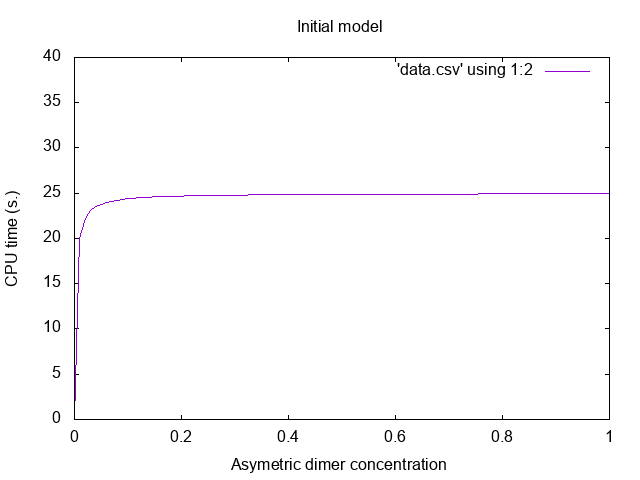Figure 7.13: Initial model (without reduction).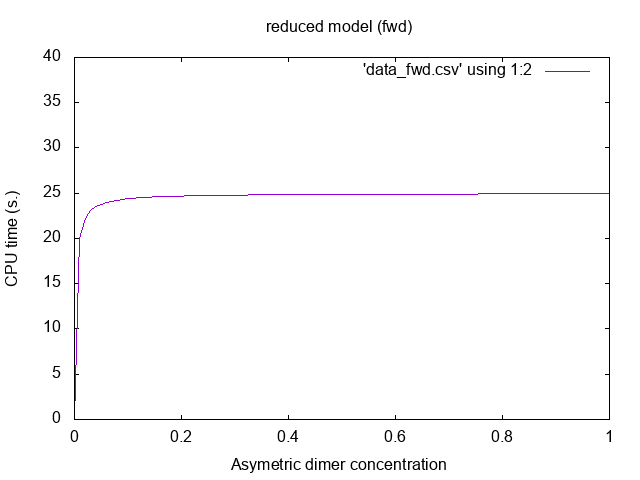Figure 7.14: Reduced model (fwd bisimulation).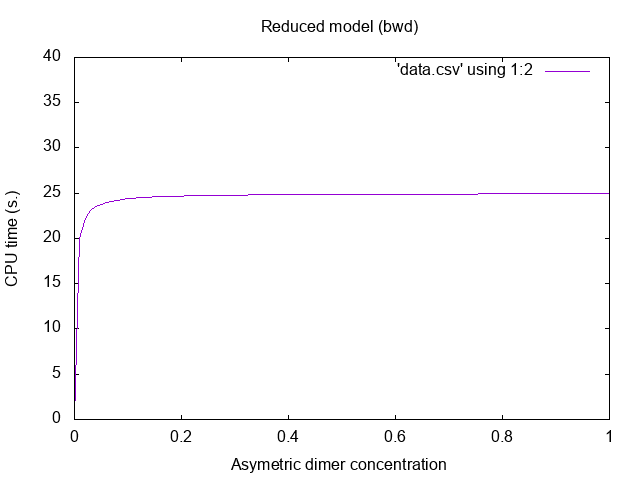Figure 7.15: Reduced model (bwd bisimulation).

### Simulation hangs after a while

If the progress bar seems stalled, it does not necessarily mean that the simulation is blocked. In particular when a simulation is triggered with a time limit (-l option of the command line) it might only indicate that the bio clock is stalled while computation events still occur. Recall that the average (bio) time one has to wait in order to apply a rule is $1∕A$, where $A$ is the sum of all the rule activities (which is equal to the number of instances that a rule has, times its kinetic rate). Whenever the number of occurrences of a rule grows too fast (if new agents are created during the simulation for instance), or if the kinetic rate of a rule is deﬁned by a function that grows rapidly, the average time increment might tend to 0 and if it remains so for a while, it will block the progress bar whose advance is proportional to the bio time [T].

In order to make sure that KaSim is not incorrectly blocked you may wish to plot the event clock against time clock using the observable %obs: 'events' [E] or run the simulation using an event limit (-e option of the command line) instead of a time limit.

### What do null events mean, why do I have any?

Using null events is a way for KaSim to compensate for some over approximation it is doing, in order to deal with large simulations more eﬃciently. They usually do not impact signiﬁcantly the performances of the simulator, unless the model contains rules using the special notation to deal with ambiguous molecularity (see Section 2.5.4). With pure Kappa rules, the ratio $r$ of null event over productive ones (that you can track using the observable %obs: 'r' [E-]/[E]) should tend to 0 when models have a lot of agents.

### No data points are generated

Make sure you have %obs or %plot instructions in your KF. Also make sure to use a reasonable value for the -p option in the command line to tell KaSim how often you wish to have points on your curves.

### Too many instances of an observable

The value of a Kappa expression $E$ is equal to the number of embeddings it has in the current mixture $M$. Embeddings are maps from agents in $E$ to agents in $M$. If $E$ has symmetries then every permutation of $E$ will be counted as a new embedding. For instance let $E=$A(x),A(x) and let $M=$A(x,y{p}[.]),A(x,y{u}[.]). KaSim will count two instances of $E$ in $M$: the one mapping the ﬁrst A of $E$ to the ﬁrst A of $M$ and the one mapping the ﬁrst A of $E$ to the second A of $M$.

### Value nan in the data ﬁle at the end of the simulation

The value nan means "Not a Number". It is generated when a plotted variable is inﬁnite. Make sure this variable is not divided by zero at some point.

## Bibliography

   Peter Buchholz. Exact and ordinary lumpability in ﬁnite Markov chains. Journal of Applied Probability, 31(1):59–75, 1994.

   Peter Buchholz. Bisimulation relations for weighted automata. Theoretical Computer Science, 393(1-3):109–123, 2008.

   Ferdinanda Camporesi and Jérôme Feret. Formal reduction for rule-based models. In Postproceedings of the Twenty-seventh Conference on the Mathematical Foundations of Programming Semantics, MFPS XXVII, volume 276C of Electonic Notes in Theoretical Computer Science, pages 31–61, Pittsburg, USA, 25–28 May 2011. Elsevier Science Publishers.

   Ferdinanda Camporesi, JÃľrÃťme Feret, Heinz Koeppl, and Tatjana Petrov. Combining model reductions. Electronic Notes in Theoretical Computer Science, 265:73 – 96, 2010.

   P. Cousot and R. Cousot. Static determination of dynamic properties of programs. In Proceedings of the Second International Symposium on Programming, pages 106–130. Dunod, Paris, France, 1976.

   Patrick Cousot, Radhia Cousot, Jérôme Feret, Laurent Mauborgne, Antoine Miné, David Monniaux, and Xavier Rival. Combination of abstractions in the Astrée static analyzer. In M. Okada and I. Satoh, editors, Eleventh Annual Asian Computing Science Conference (ASIAN’06), pages 1–24, Tokyo, Japan, LNCS 4435, December 6–8 2007. Springer, Berlin.

   Vincent Danos, Jérôme Féret, Walter Fontana, and Russell Harmer. Rule based modelling , symmetries, reﬁnements. In Proc. FMSB, volume 5054 of LNCS, 2008.

   Vincent Danos, Jérôme Feret, Walter Fontana, Russell Harmer, Jonathan Hayman, Jean Krivine, Chris Thompson-Walsh, and Glynn Winskel. Graphs, rewriting and pathway reconstruction for rule-based models. In Schloss Dagstuhl Leibniz-Zentrum fuer Informatik, editor, FSTTCS 2012 - IARCS Annual Conference on Foundations of Software Technology and Theoretical Computer Science, volume 18 of LIPIcs, pages 276–288, 2012.

   Vincent Danos, Jérôme Feret, Walter Fontana, Russell Harmer, and Jean Krivine. Rule-based modelling and model perturbation. Trans. Computational Systems Biology, 11:116–137, 2009.

   Vincent Danos, Jérôme Feret, Walter Fontana, and Jean Krivine. Scalable simulation of cellular signaling networks. In Proc. APLAS’07, volume 4807 of LNCS, pages 139–157, 2007.

   Vincent Danos, Jérôme Feret, Walter Fontana, and Jean Krivine. Abstract interpretation of cellular signalling networks. In Francesco Logozzo, Doron A. Peled, and Lenore D. Zuck, editors, Proceedings of the Ninth International Conference on Veriﬁcation, Model Checking and Abstract Interpretation, VMCAI$\phantom{\rule{0.3em}{0ex}}$’2008, volume 4905 of Lecture Notes in Computer Science, pages 83–97, San Francisco, USA, 7–9 January 2008. Springer, Berlin, Germany.

   Vincent Danos, Jérôme Feret, Walter Fontanta, Russ Harmer, and Jean Krivine. Rule based modeling of biological signaling. In Luís Caires and Vasco Thudichum Vasconcelos, editors, Proceedings of CONCUR 2007, volume 4703 of LNCS, pages 17–41. Springer, 2007.

   Vincent Danos and Cosimo Laneve. Formal molecular biology. Theoretical Computer Science, 325, 2004.

   James R. Faeder, Mickael L. Blinov, and William S. Hlavacek. Rule based modeling of biochemical networks. Complexity, pages 22–41, 2005.

   Lisbeth Fajstrup, Eric Goubault, and Martin Raußen. Detecting deadlocks in concurrent systems. In Proc. CONCUR ’98, volume 1466 of LNCS, 1998.

   Jérôme Feret. Occurrence counting analysis for the pi-calculus. Electronic Notes in Theoretical Computer Science, 39.(2), 2001. Workshop on GEometry and Topology in COncurrency theory, PennState, USA, August 21, 2000.

   Jérôme Feret. Reachability analysis of biological signalling pathways by abstract interpretation. In T.E. Simos, editor, Proceedings of the International Conference of Computational Methods in Sciences and Engineering, ICCMSE$\phantom{\rule{0.3em}{0ex}}$’2007, Corfu, Greece, number 963.(2) in American Institute of Physics Conference Proceedings, pages 619–622, Corfu, Greece, 25–30 September 2007. American Institute of Physics.

   Jerome Feret. An algebraic approach for inferring and using symmetries in rule-based models. Electronic Notes in Theoretical Computer Science, 316:45 – 65, 2015. 5th International Workshop on Static Analysis and Systems Biology (SASB 2014).

   Jérôme Feret, Heinz Koeppl, and Tatjana Petrov. Stochastic fragments: A framework for the exact reduction of the stochastic semantics of rule-based models. International Journal of Software and Informatics, 7(4):527 – 604, 2013.

   Jérôme Feret and Kim Quyên Lý. Reachability analysis via orthogonal sets of patterns. In Seventeenth International Workshop on Static Analysis and Systems Biology (SASB’16), ENTCS. elsevier. to appear.

   Daniel T. Gillespie. A general method for numerically simulating the stochastic time evolution of coupled chemical reactions. Journal of Computational Physics, 22(4):403–434, 1976.

   Daniel T. Gillespie. Exact stochastic simulation of coupled chemical reactions. Journal of Physical Chemistry, 81(25):2340–2361, 1977.

   Michael Karr. Aﬃne relationships among variables of a program. Acta Informatica, 1976.

   Peter Kreyßig. Chemical Organisation Theory Beyond Classical Models: Discrete Dynamics and Rule-based Models. PhD thesis, 2015.

   R. E. Tarjan. Depth ﬁrst search and linear graph algorithms. SIAM Journal of Computing, 1:146–160, 1972.

## Index

activity, 24, 31, 39
agent signature, 18
agent signature, 9, 14, 18, 23, 25
algebraic expression, 24
algebraic expression, 15, 17, 20, 26, 28

bi-directional rule, 17
boolean expression, 26

causal ﬂow, 37, 38
causality, 37
chemical notation, 17

data ﬁle, 15
data ﬁle, 15, 114
declaration, 9, 11, 15, 17, 23, 25, 28, 29, 37, 39, 41
default state, 18
DIN, 39
don’t care don’t write, 18, 25
don’t care don’t write, 13, 18
dynamic inﬂuence network, 39

edit rules, 19
eﬀect, 26, 27
embedding, 114
event, 16, 31

graph, 31

hybrid rules, 23

initial condition, 11, 24
internal state, 14
intervention, 11

Kappa ﬁle, 27
Kappa ﬁle, 9, 11, 14, 15, 17, 24, 34, 41, 42, 114
kinetic rate, 15, 20
deterministic rate constant, 20
stochastic rate constant, 20

left hand side, 17

mixture, 22, 24, 27, 29, 31, 41, 114

null event, 16, 113

rate, 31
right hand side, 17
rule, 11, 17